# RS Aggarwal Solutions for Class 10 Maths Chapter 3 Linear Equations in Two Variables Exercise 3A

RS Aggarwal Solutions for Class 10 Maths Chapter 3 Linear Equations in Two Variables, contains solutions for exercise 3A questions. These solutions are designed to make the students of 10th Class prepared for their board exams, the experts at BYJU’S have formulated these solutions based on CBSE syllabus. RS Aggarwal solutions on exercise 3a is all about solving simultaneous linear equations using graphical method. Students can download the RS Aggarwal Solutions of Class 10 mathematics to understand the concept.

## Download PDF of RS Aggarwal Solutions for Class 10 Maths Chapter 3 Linear Equations in Two Variables Exercise 3A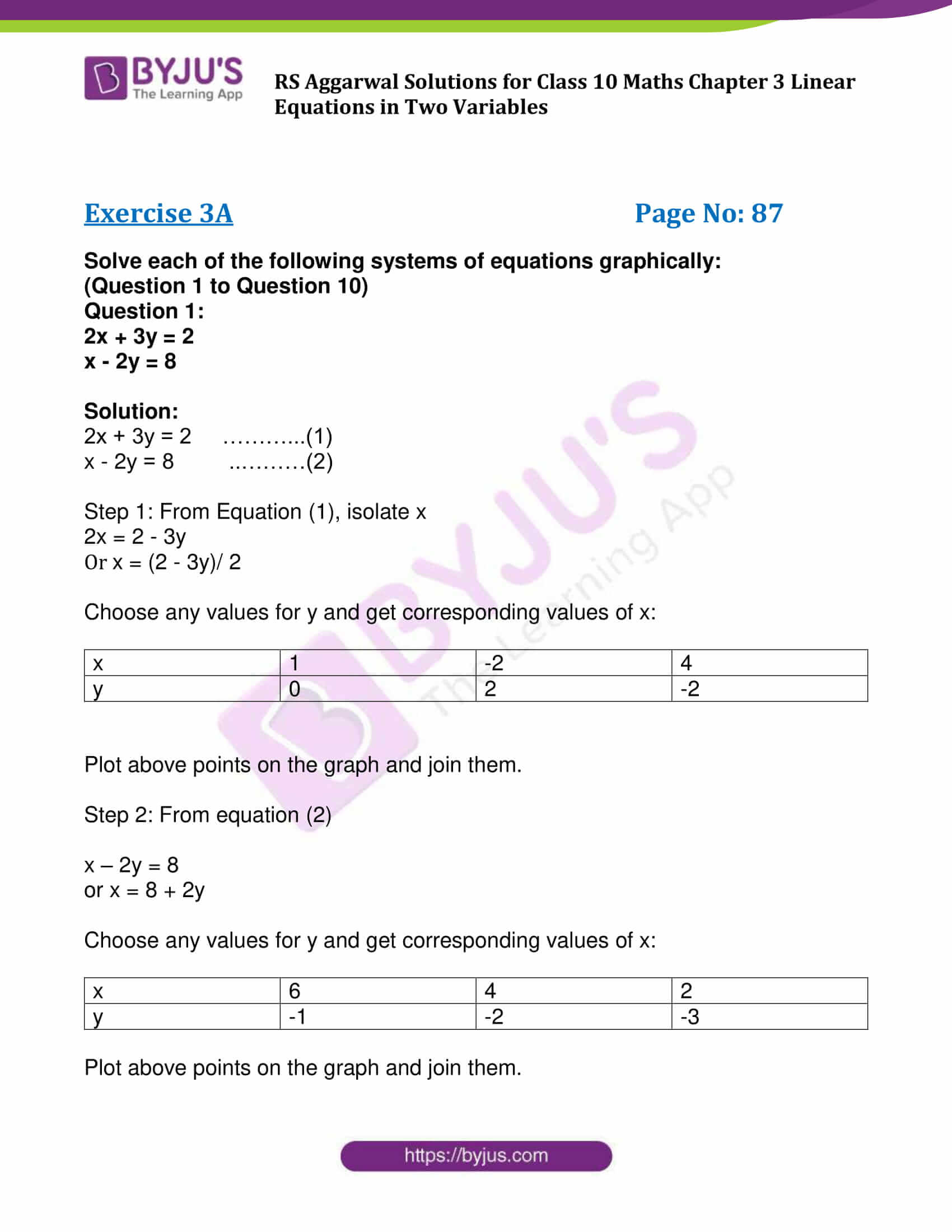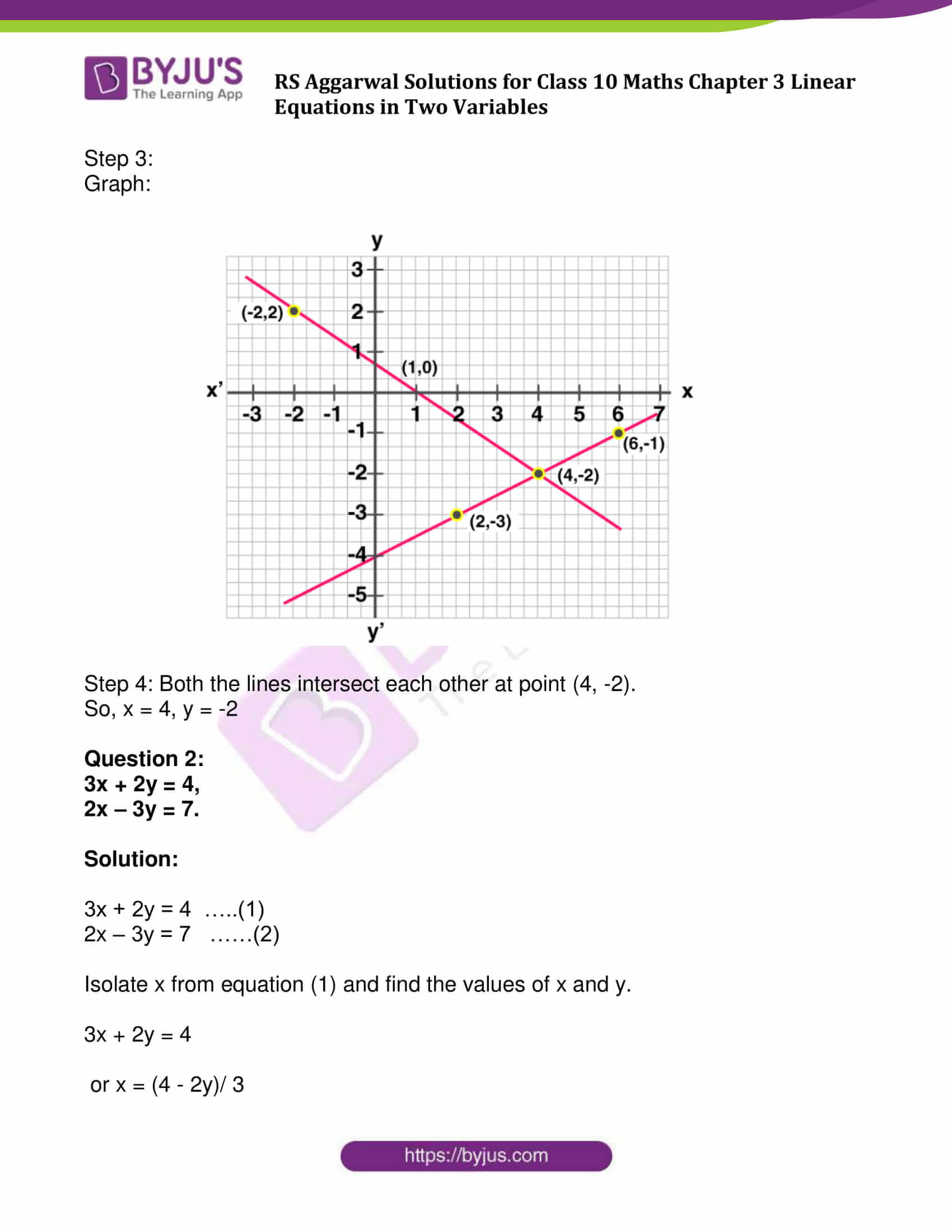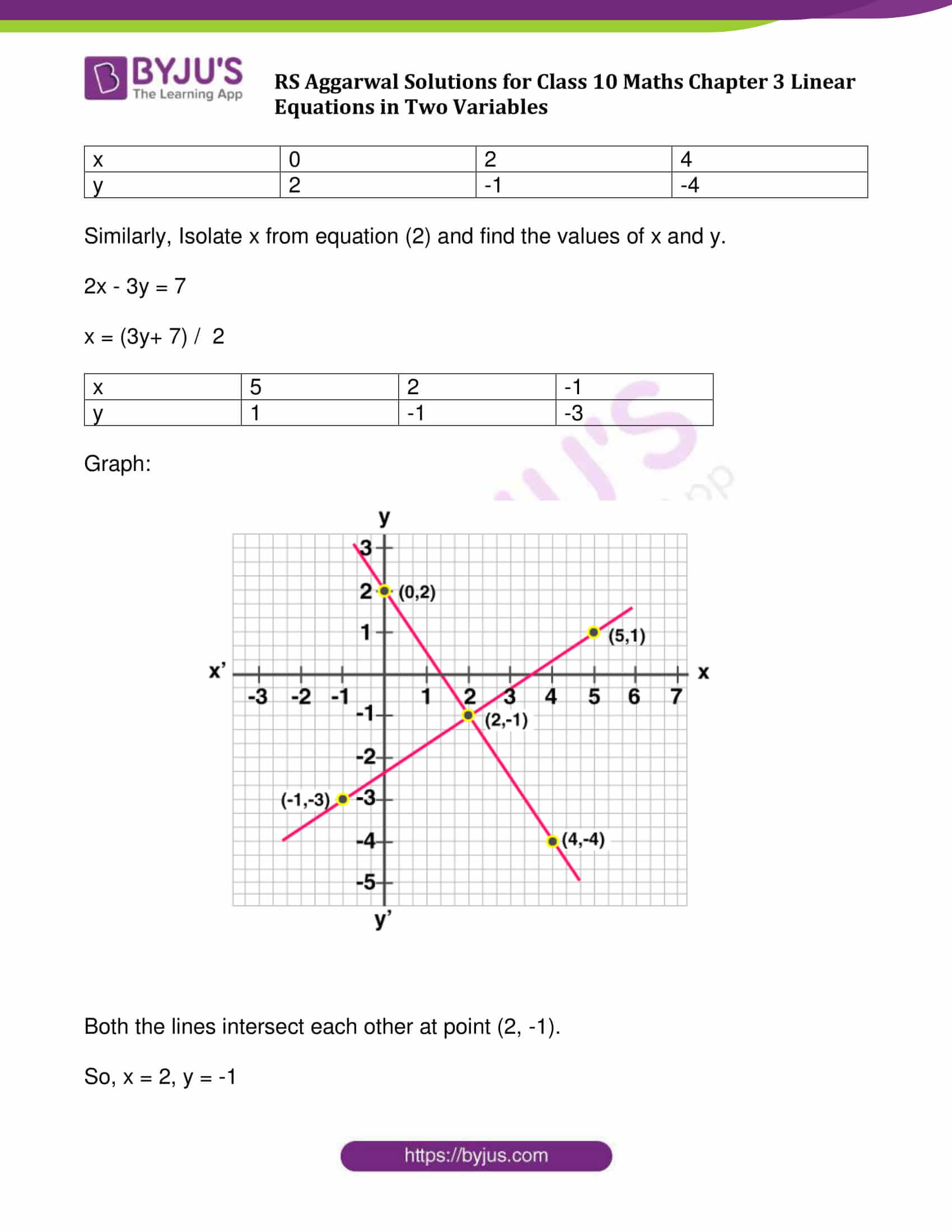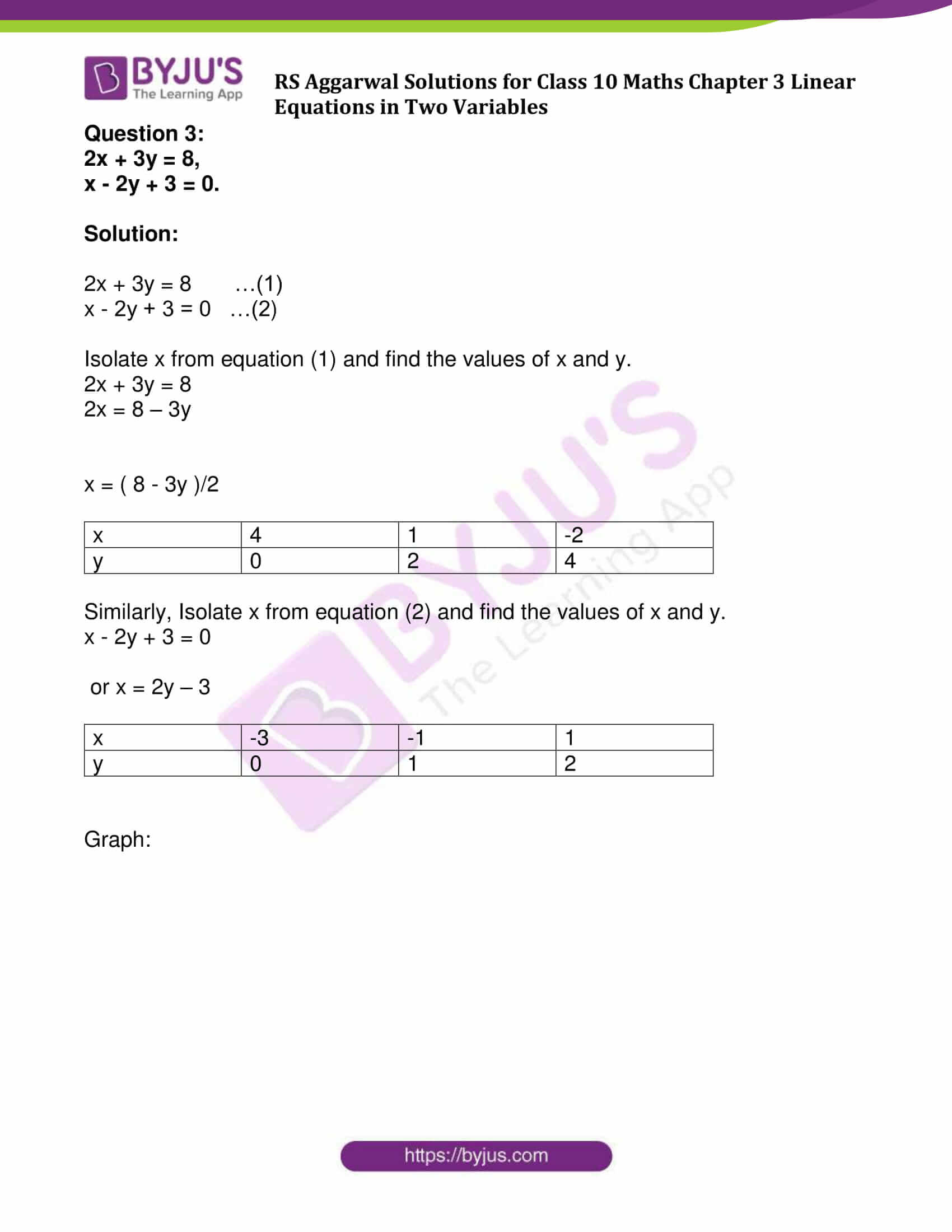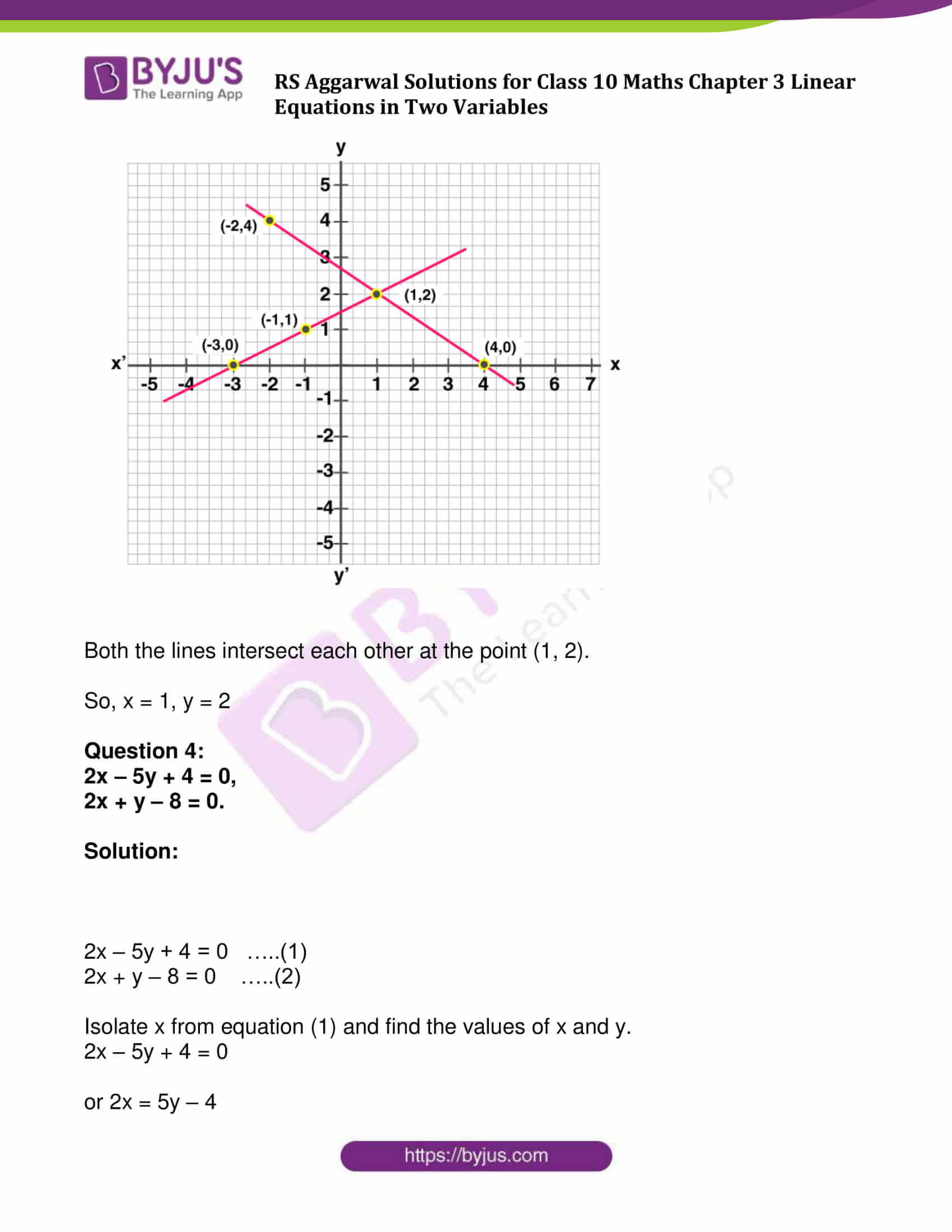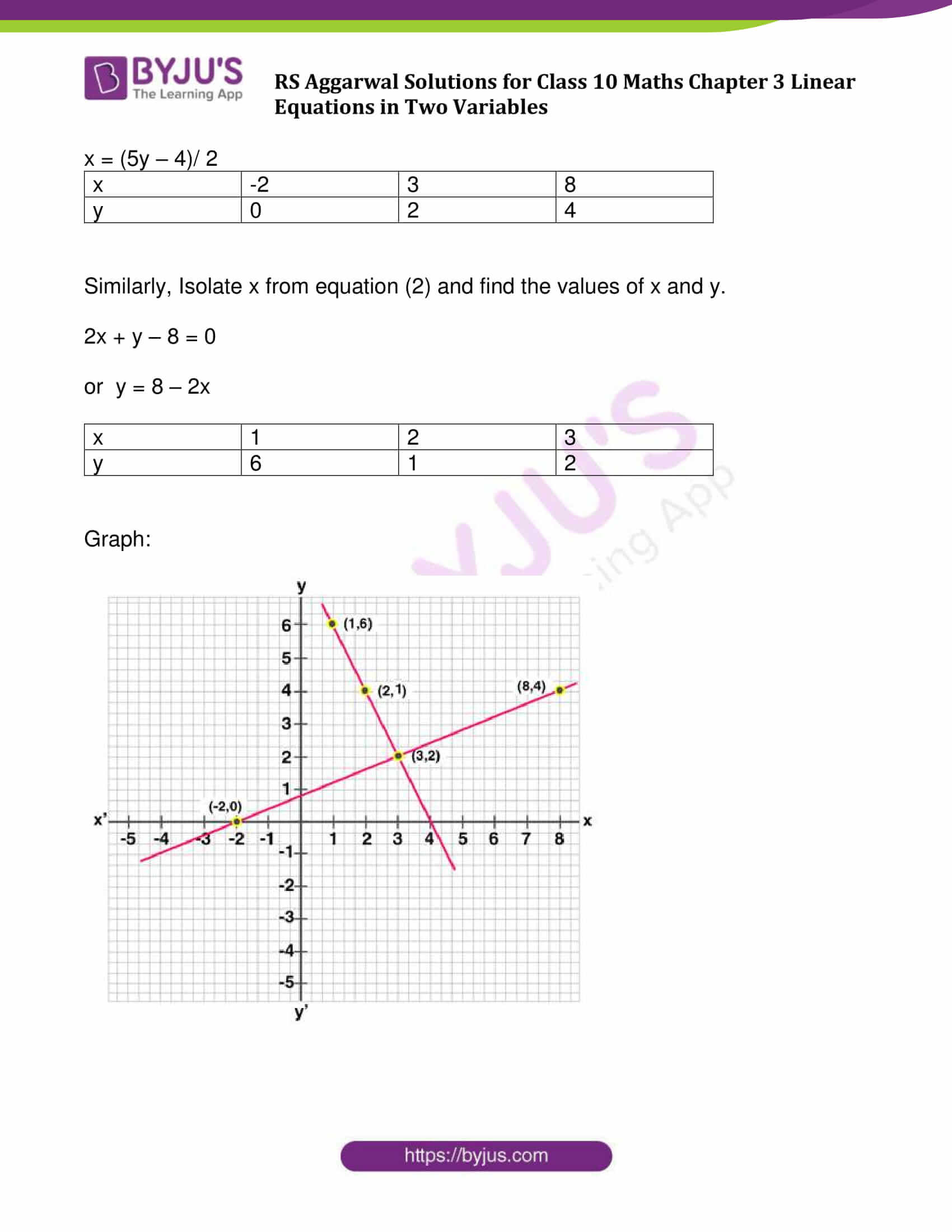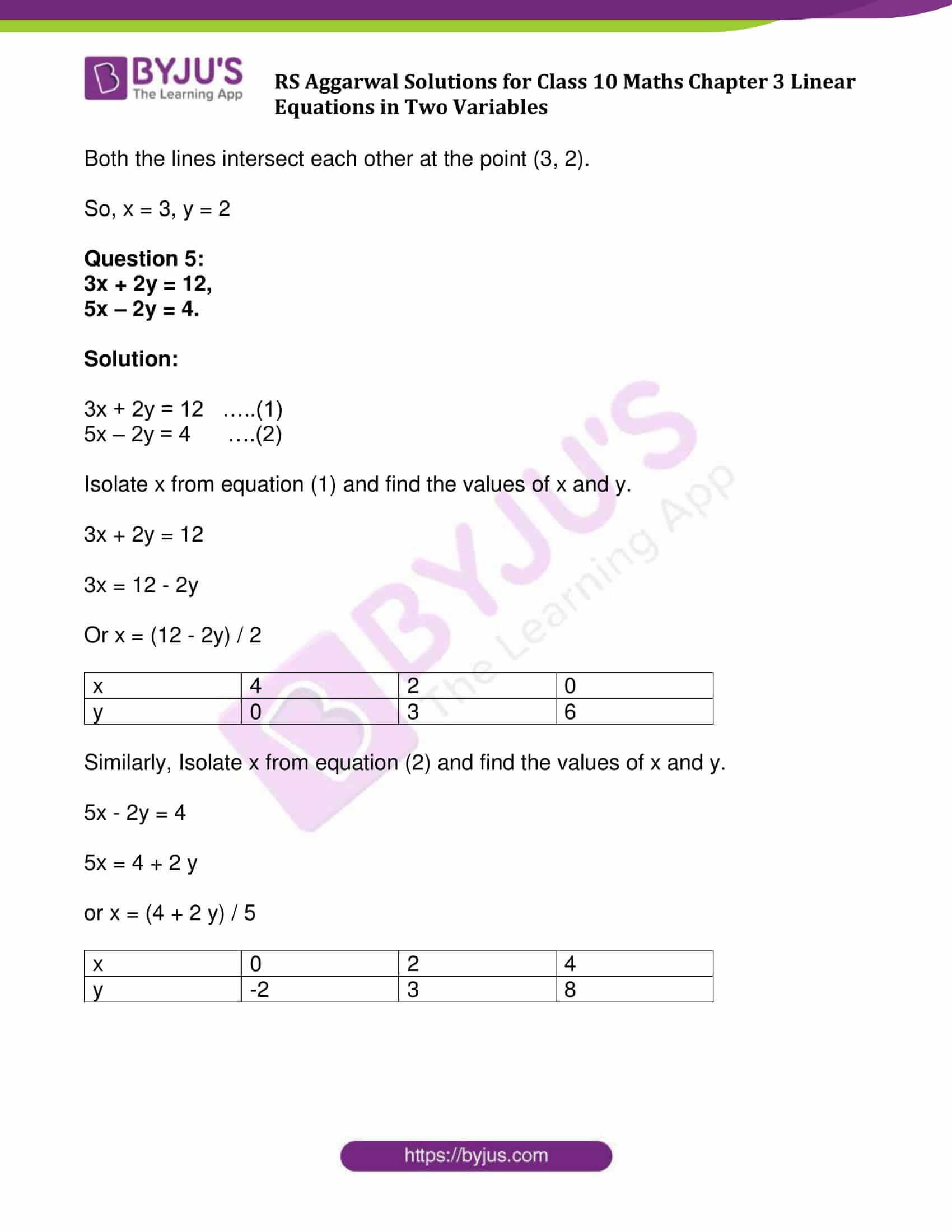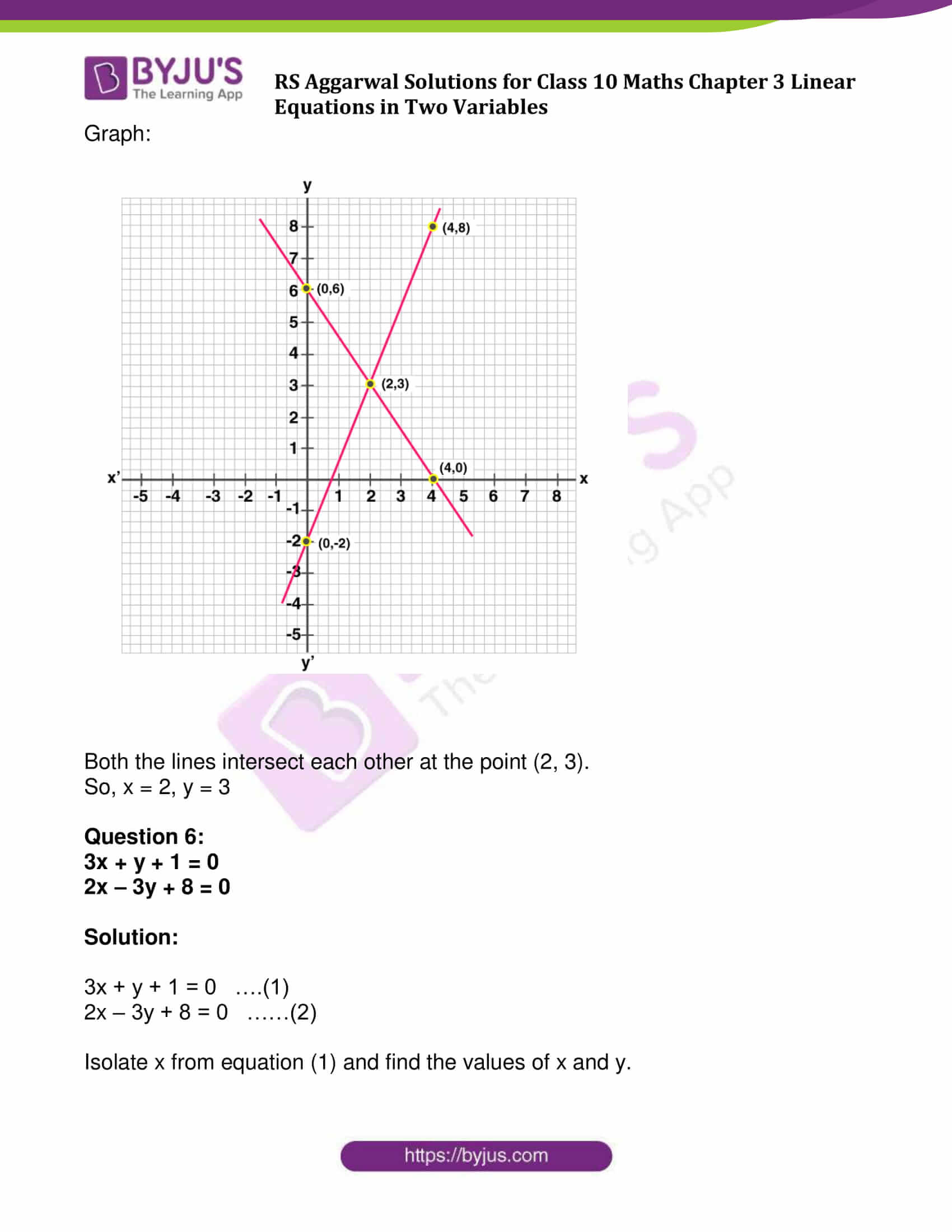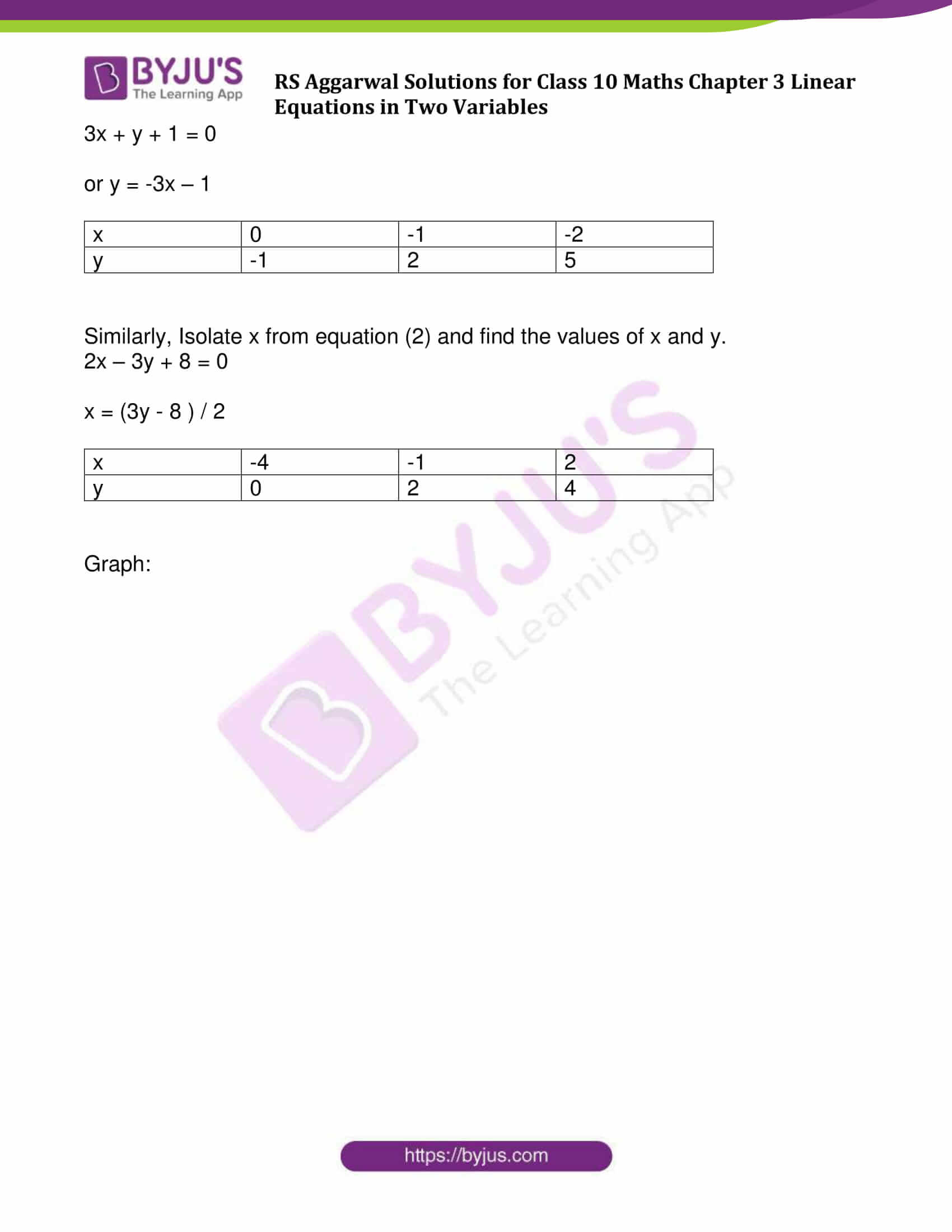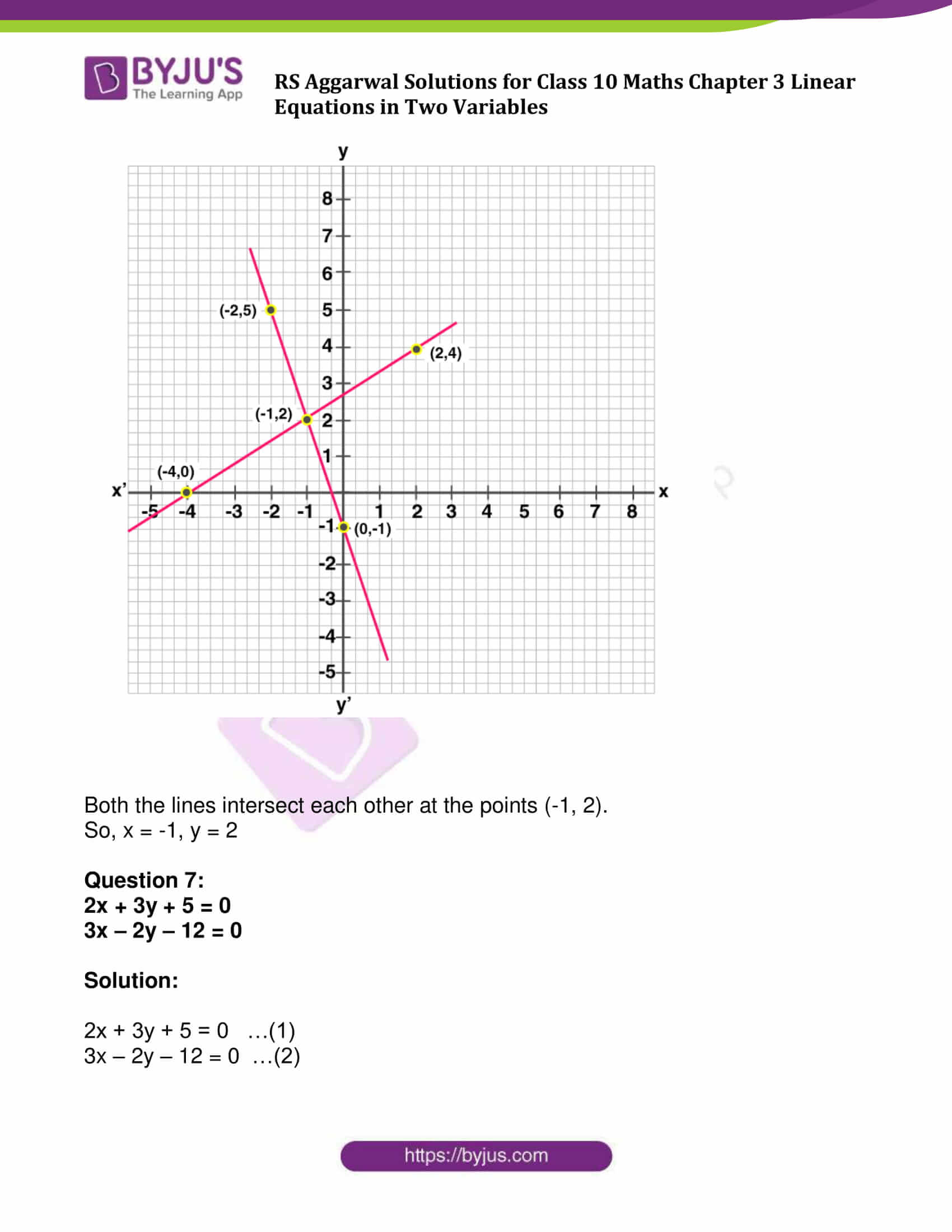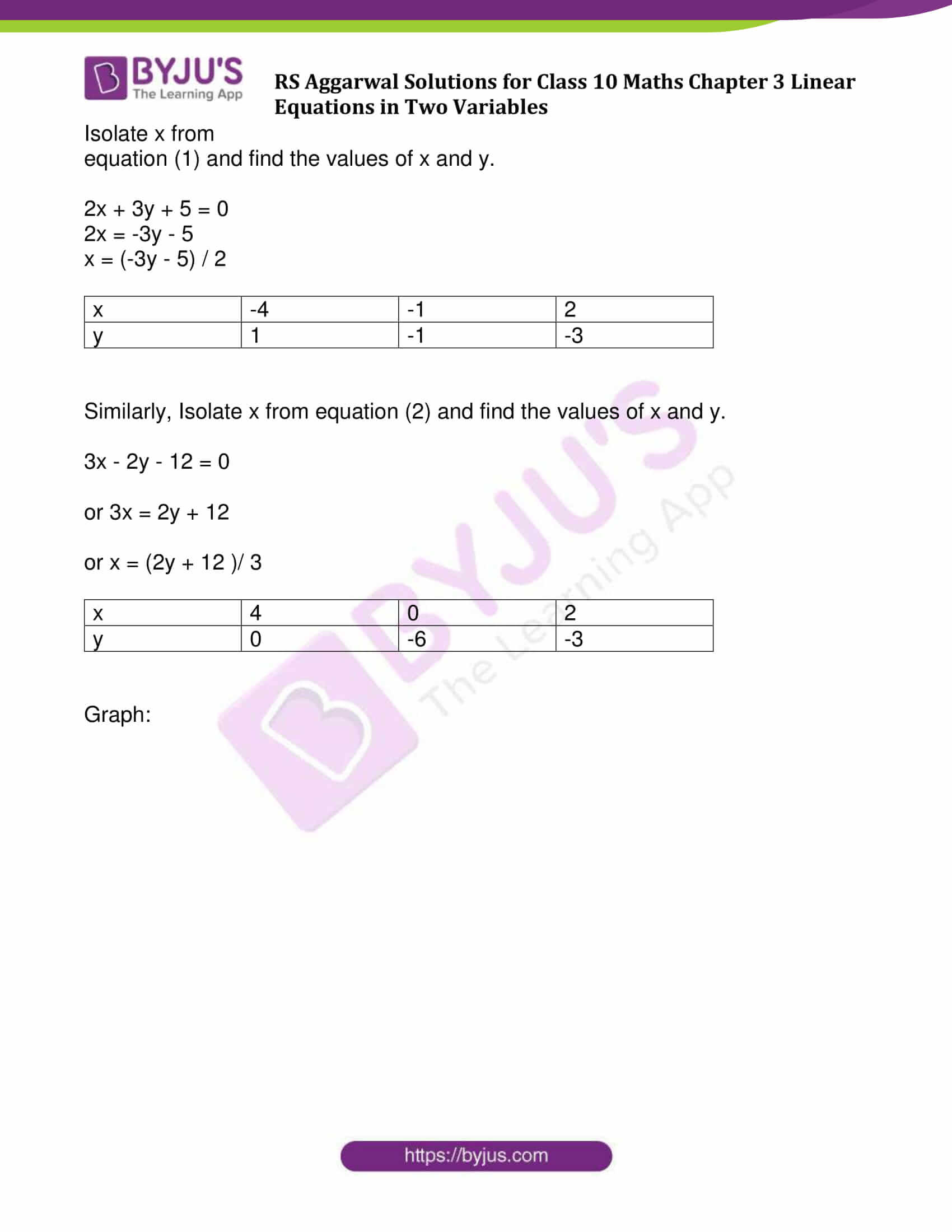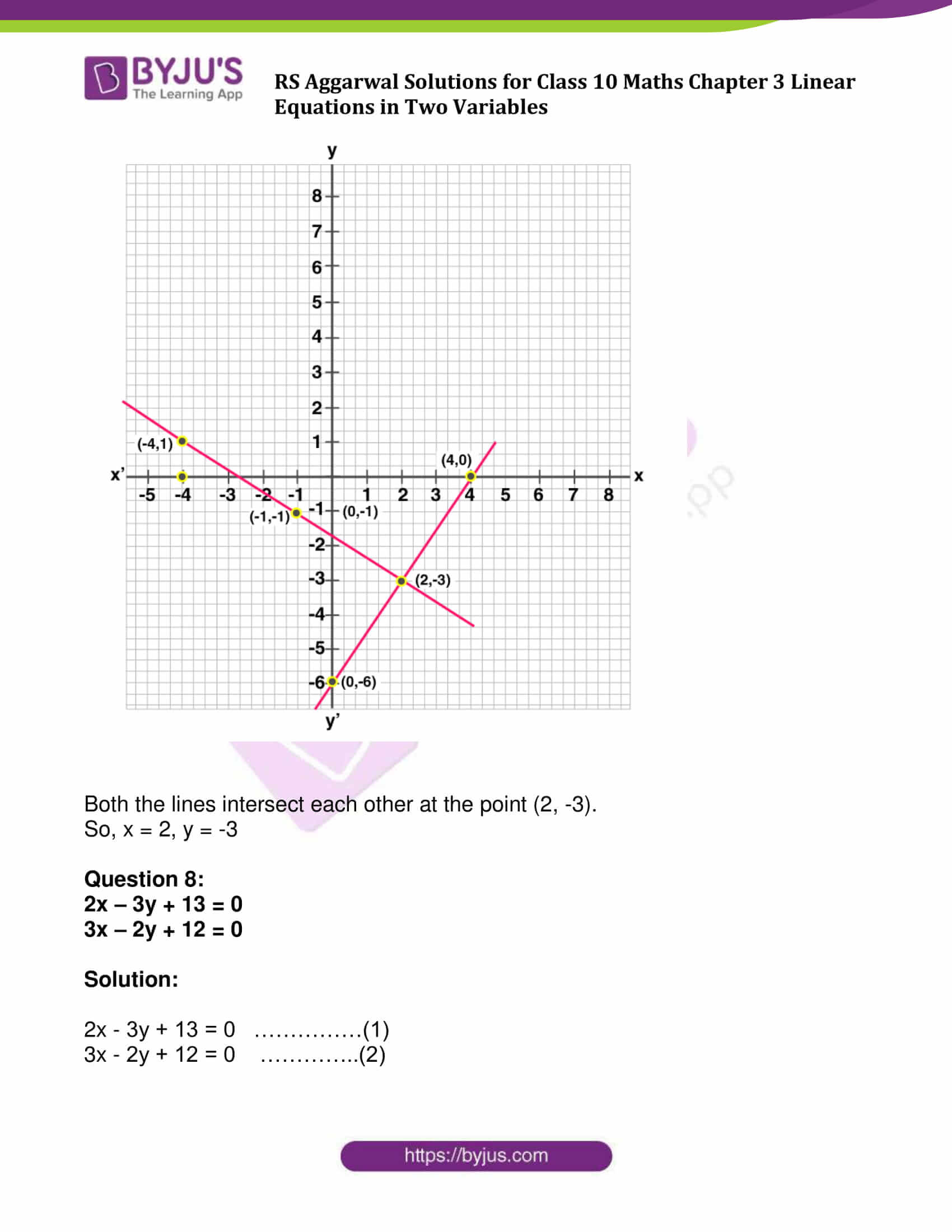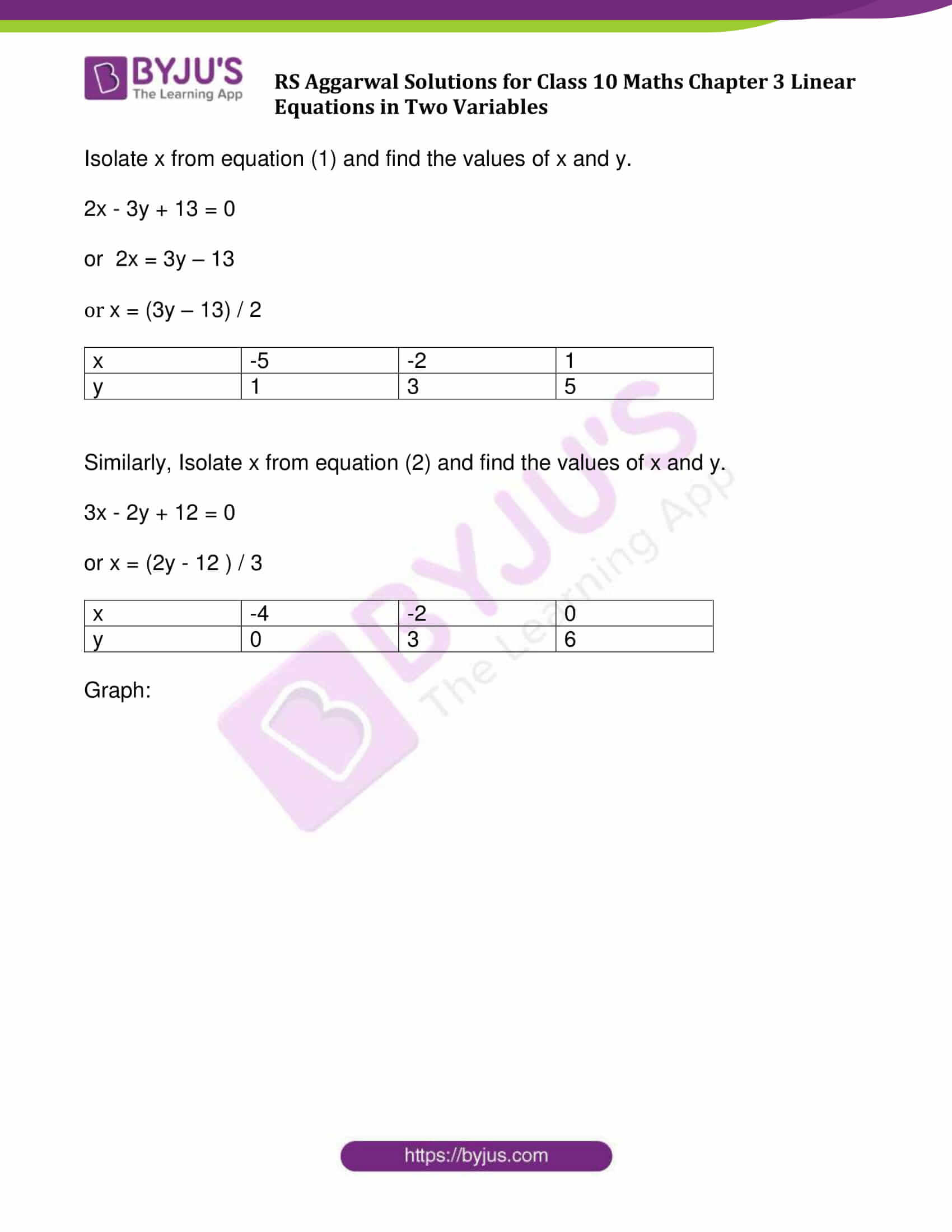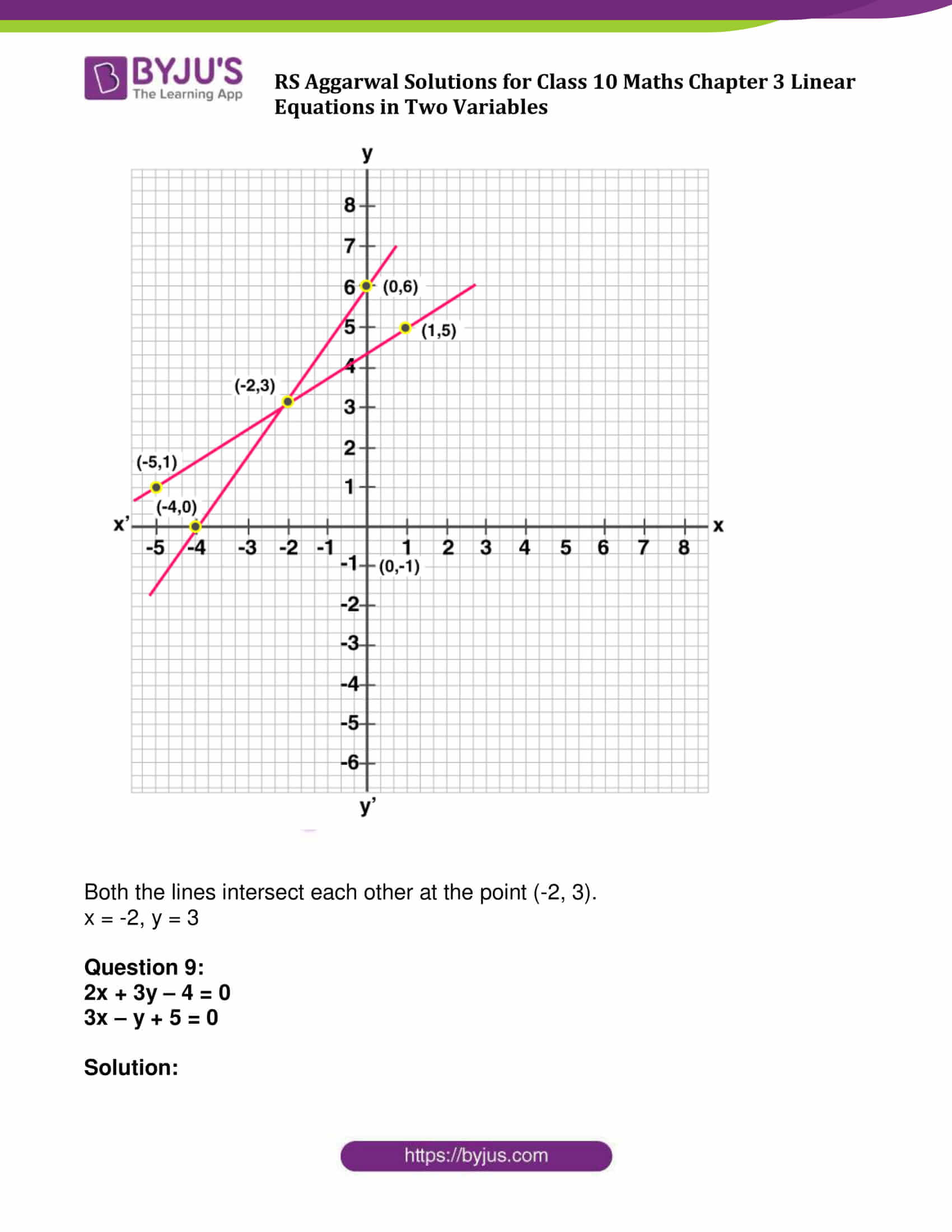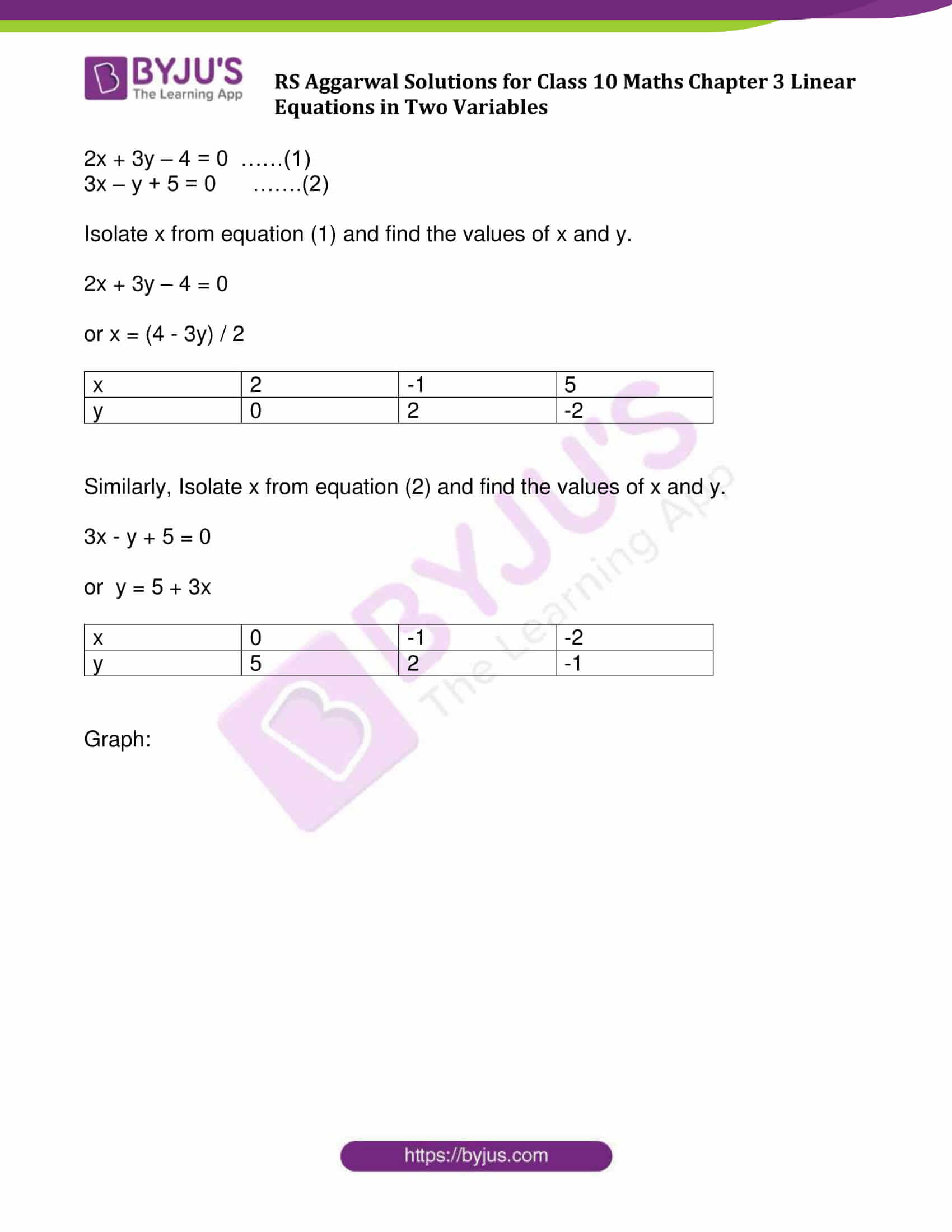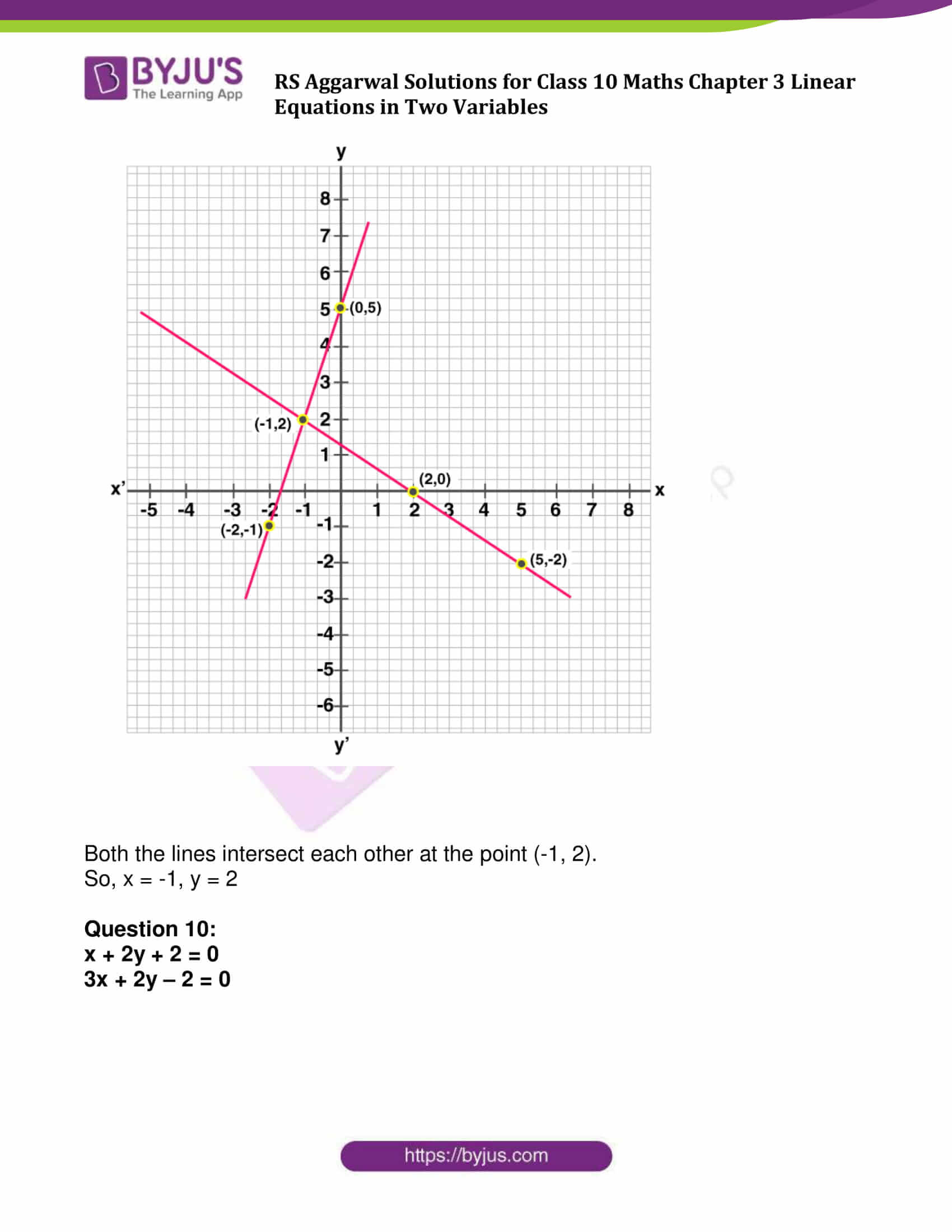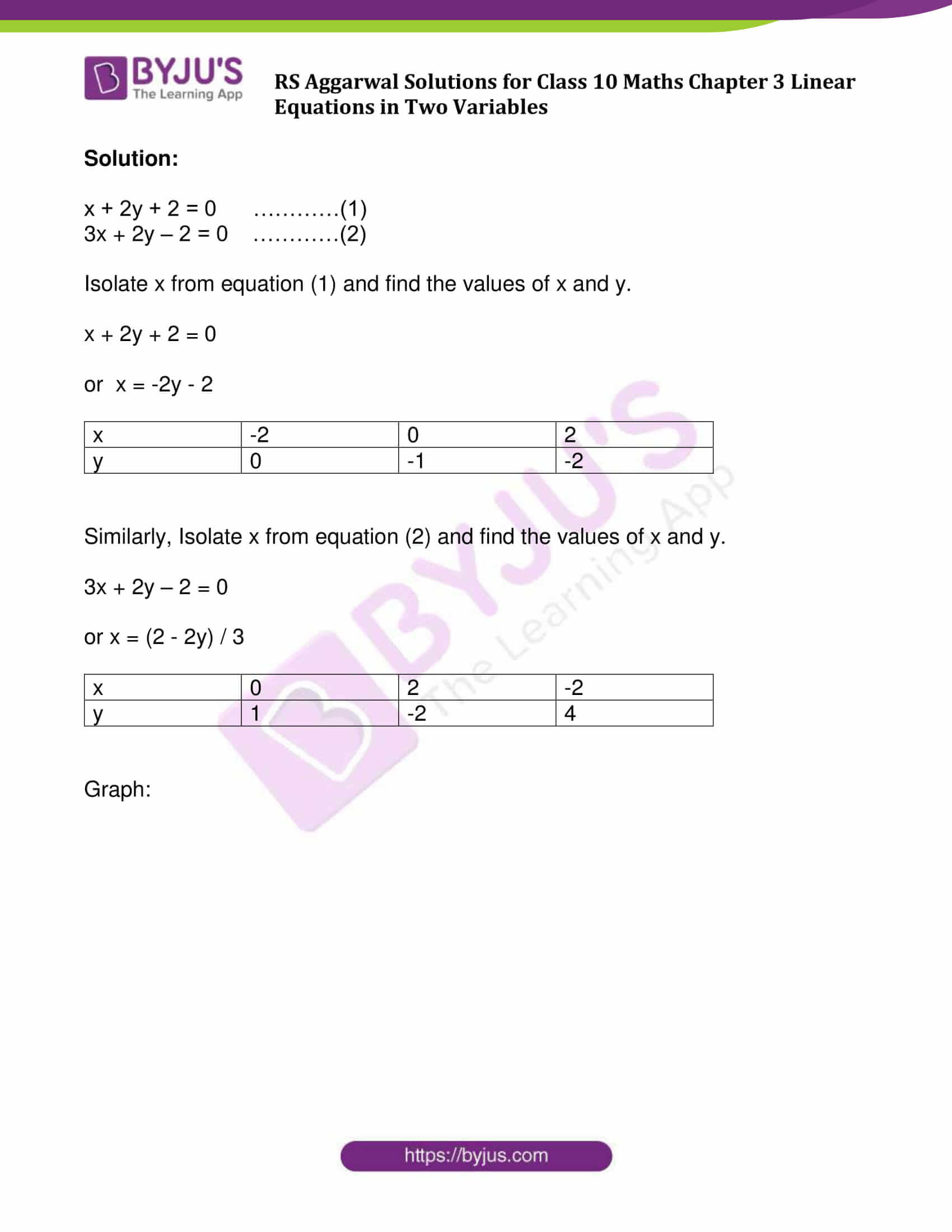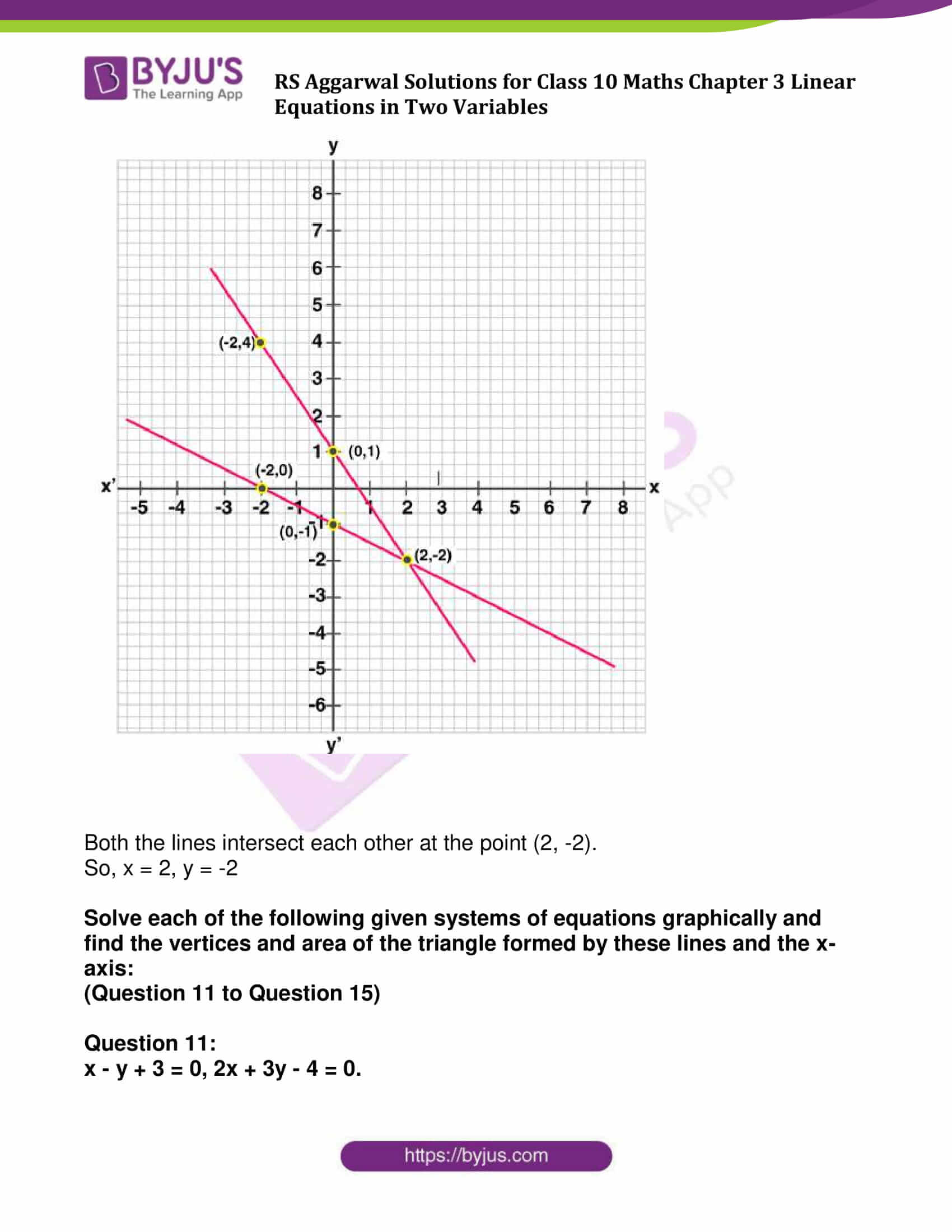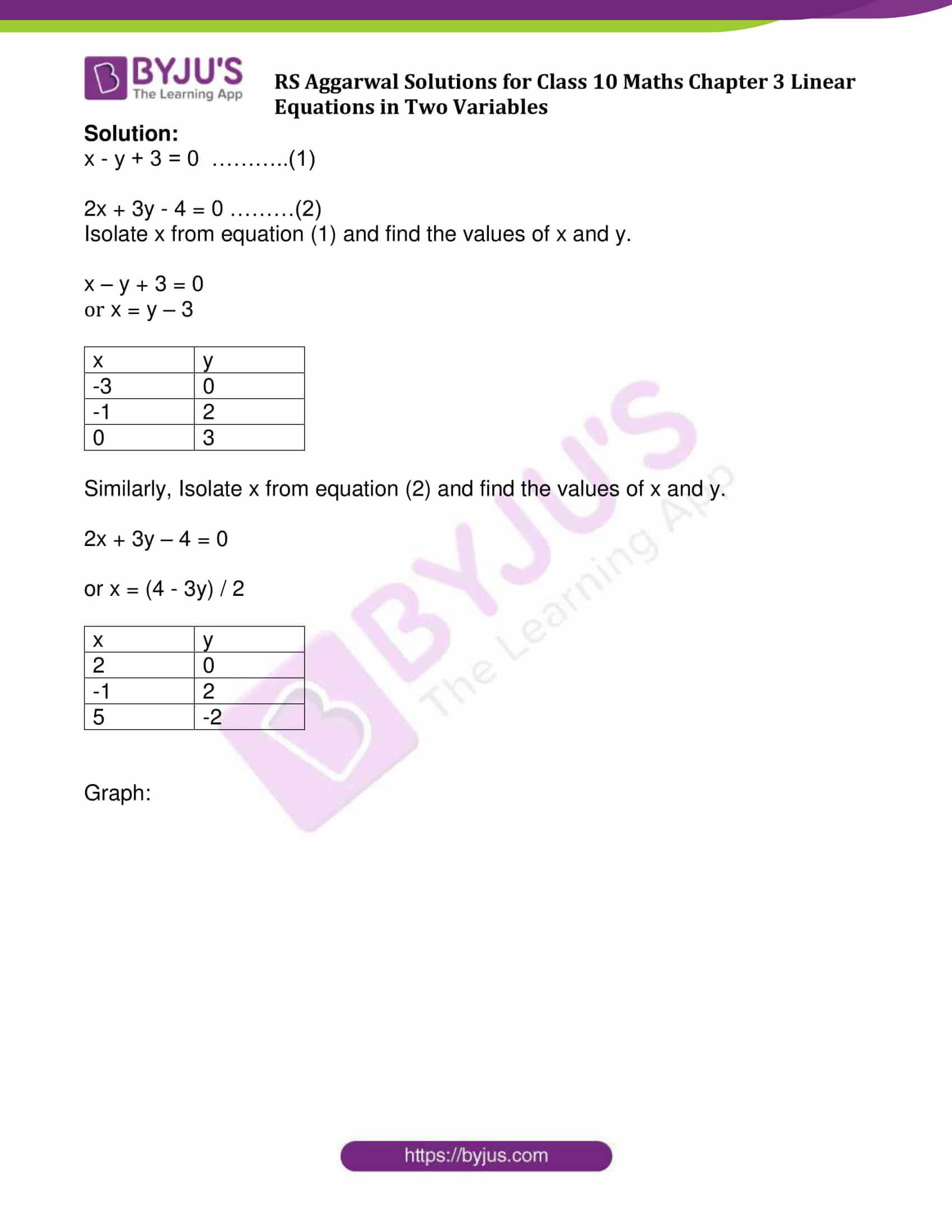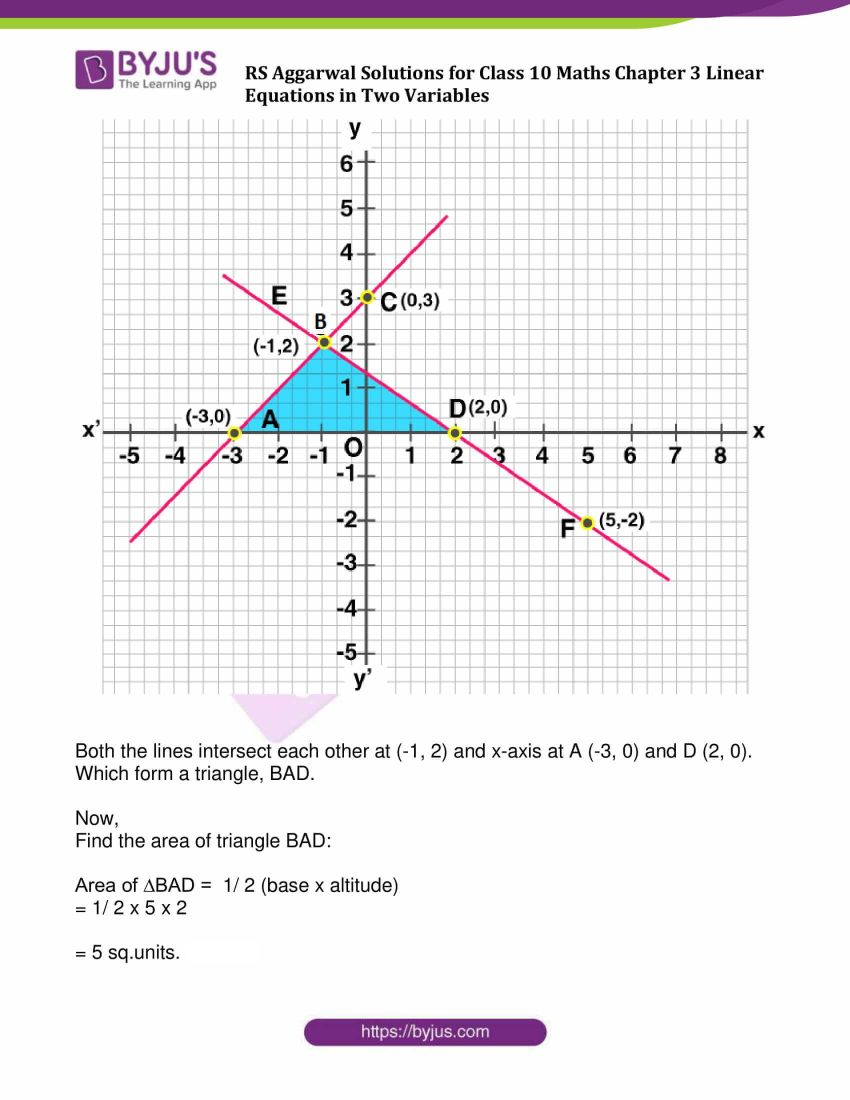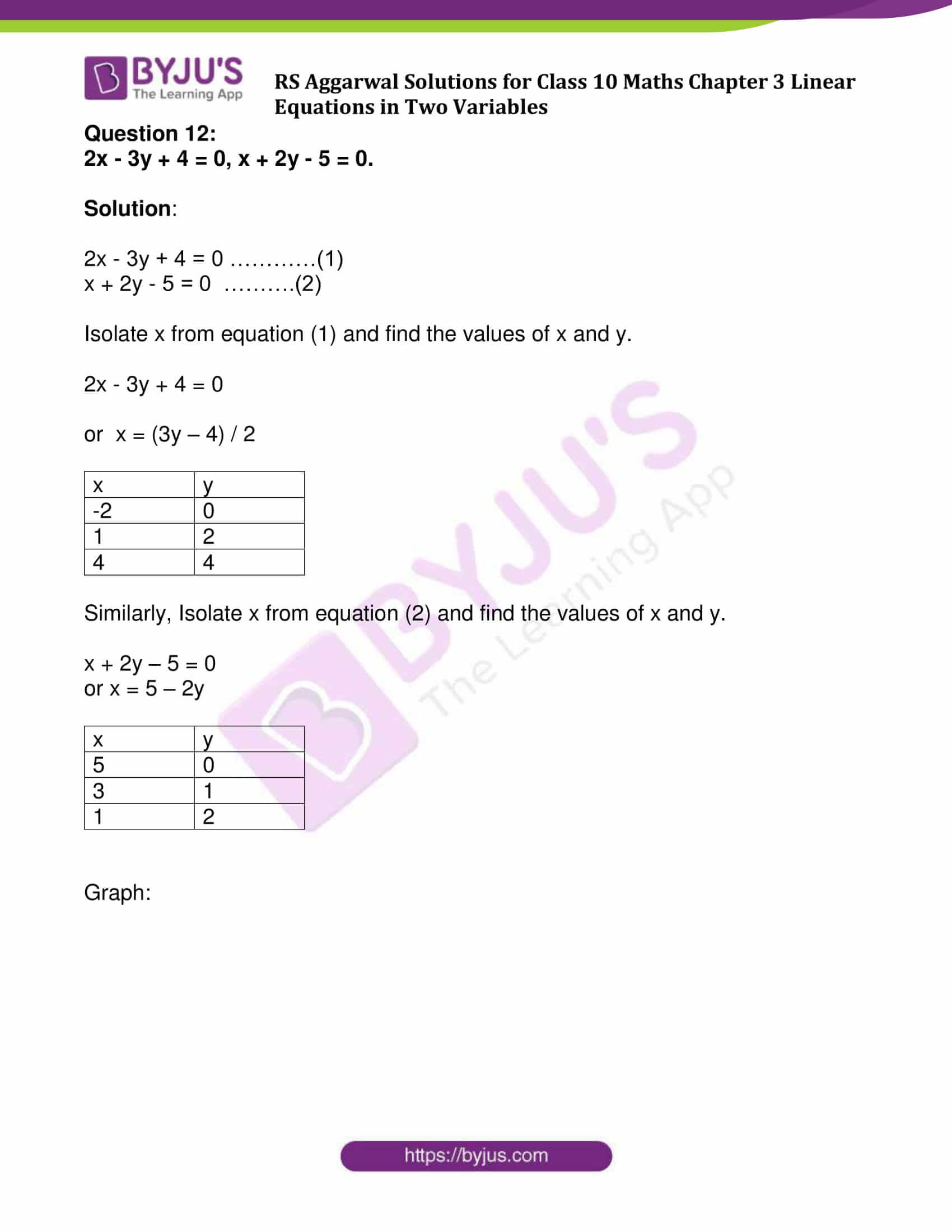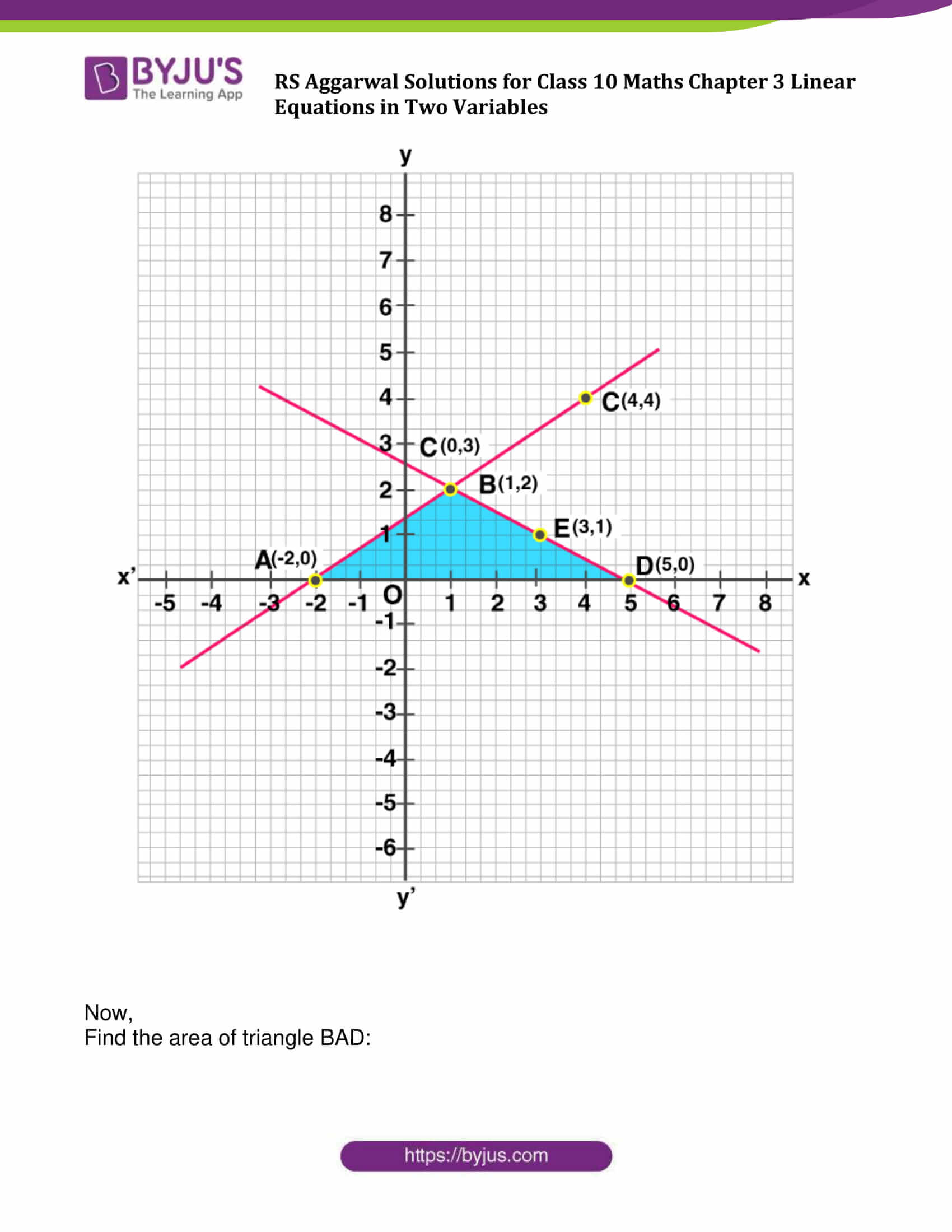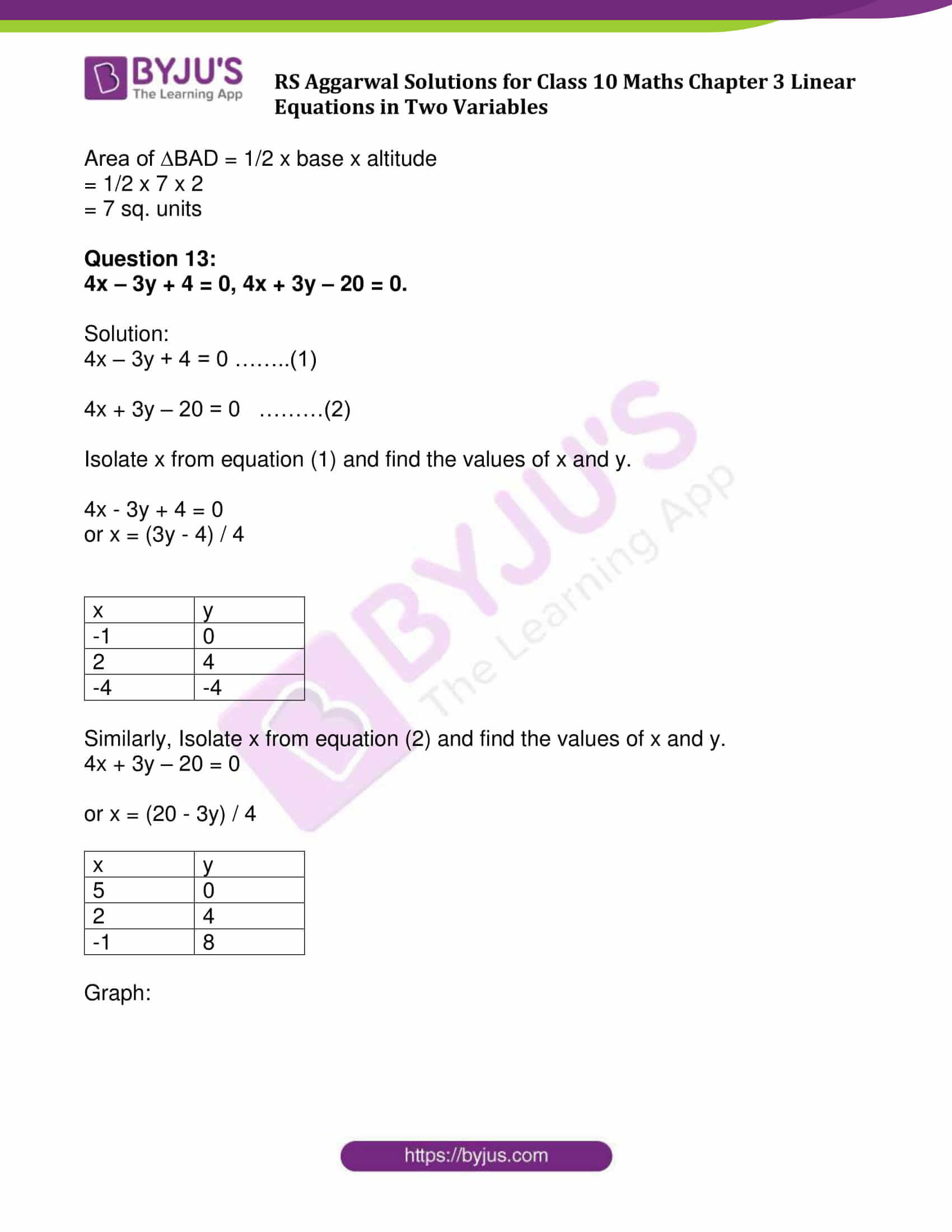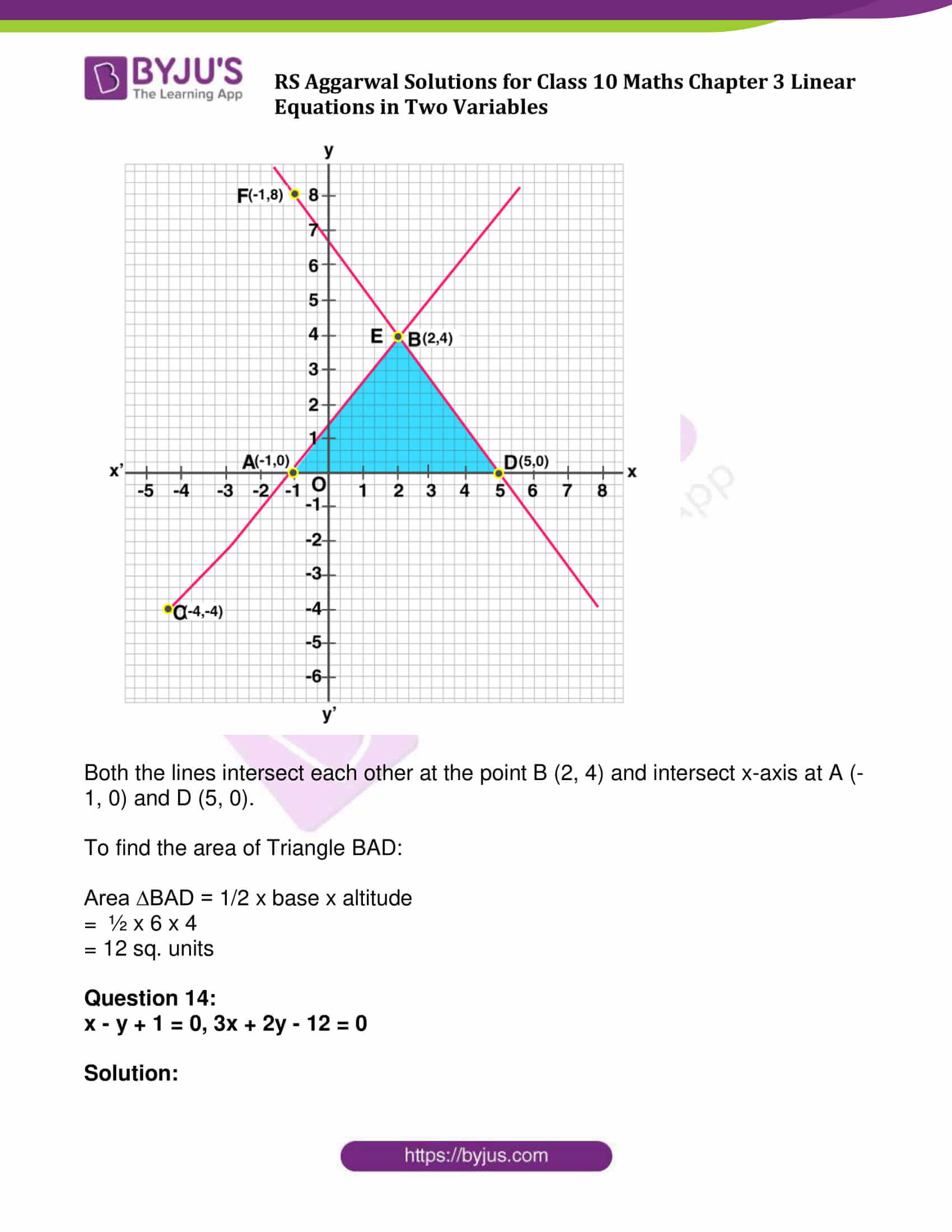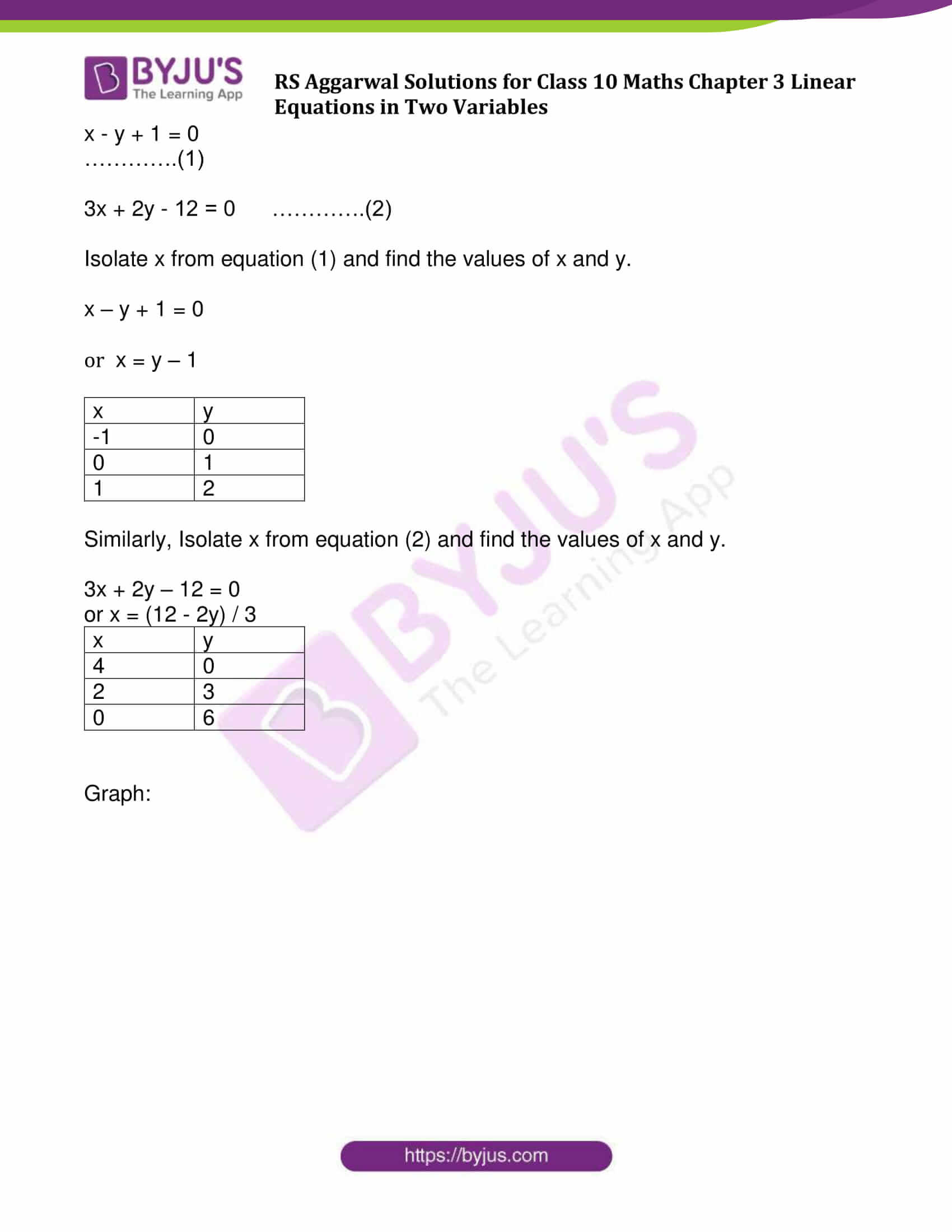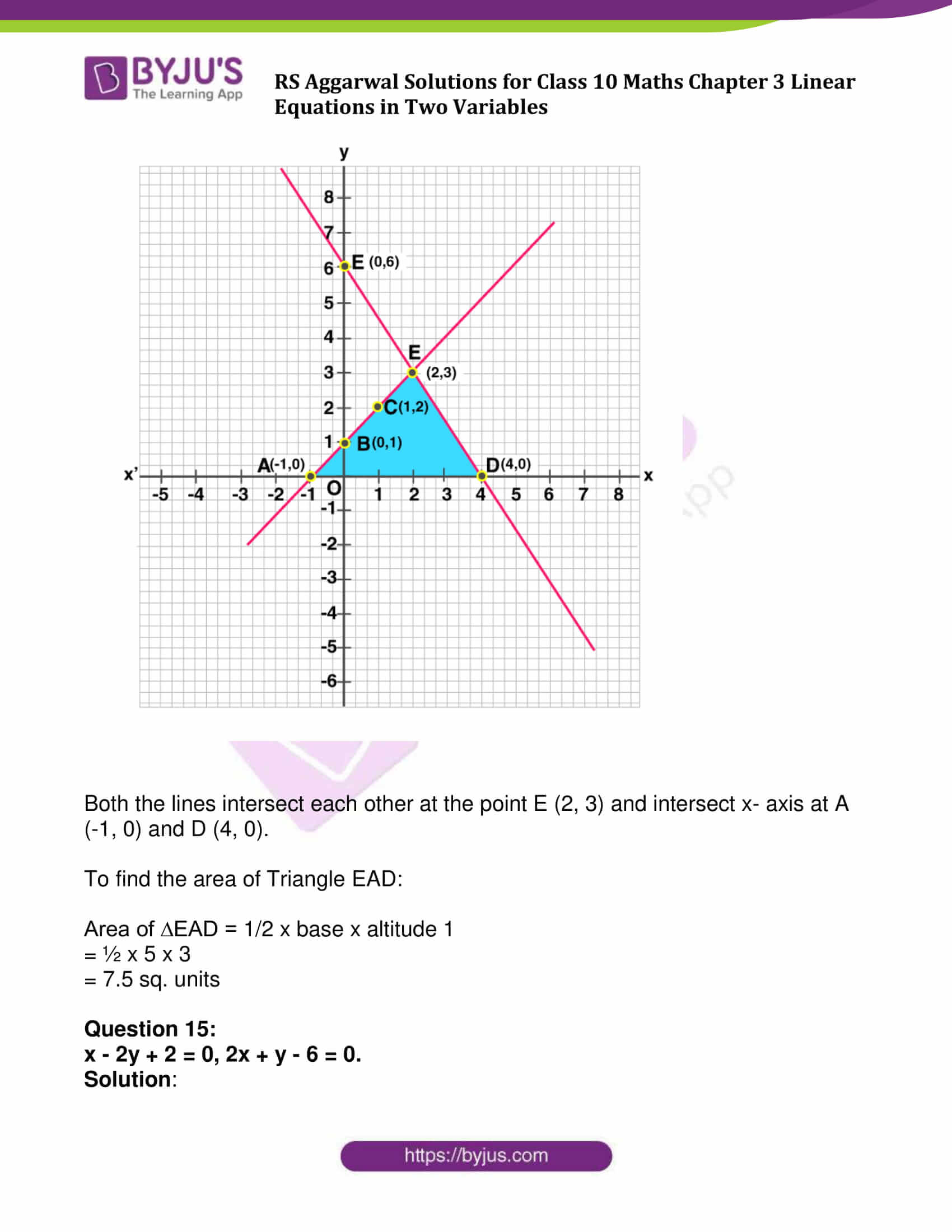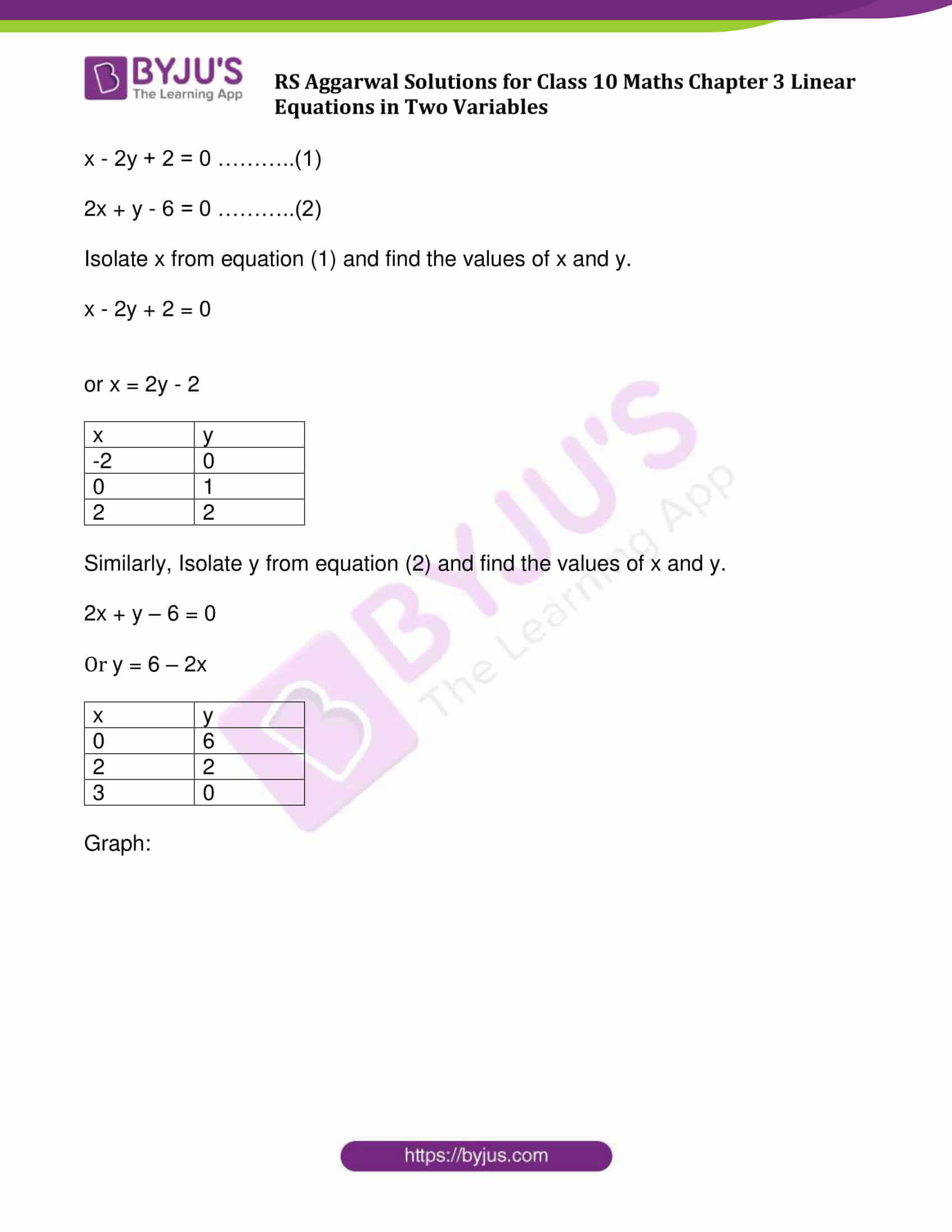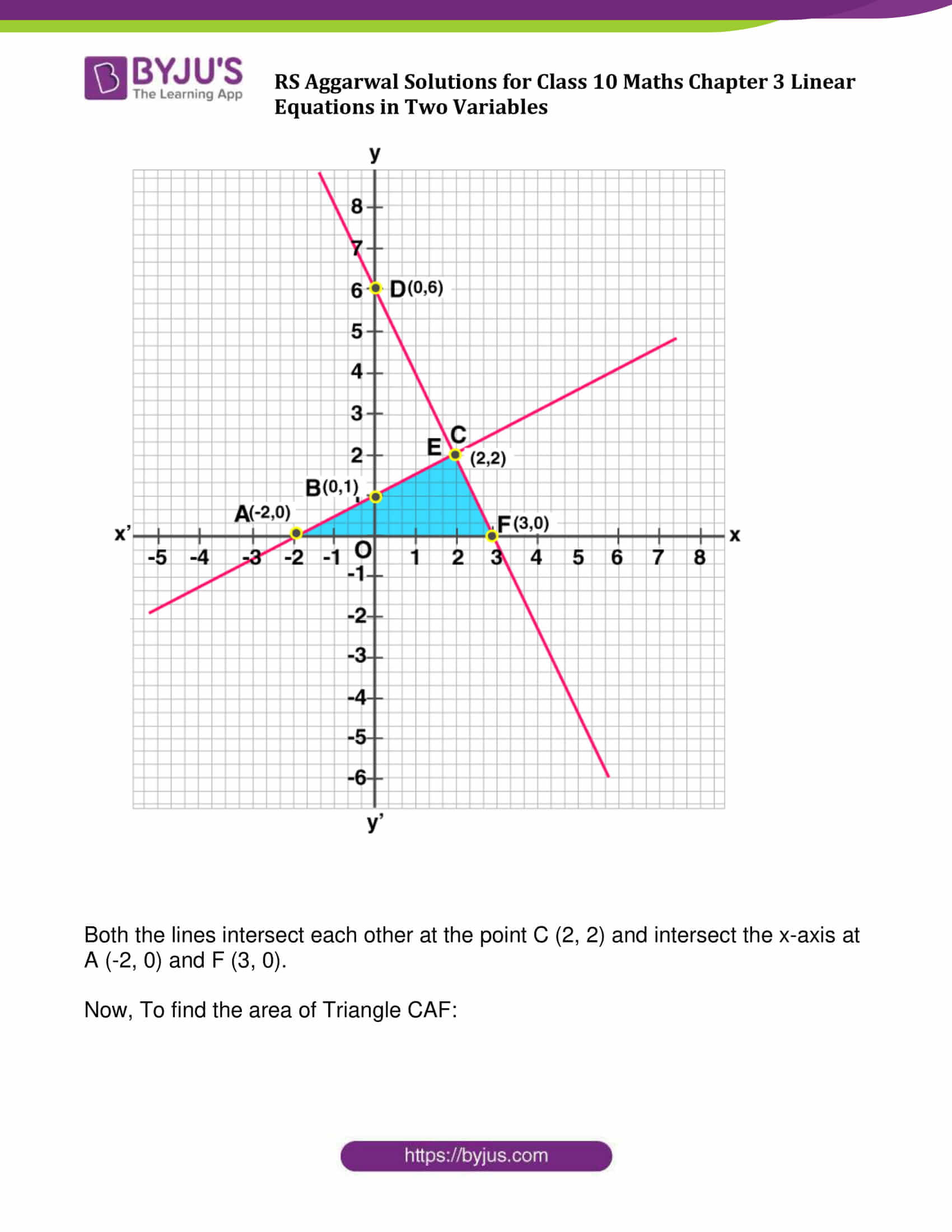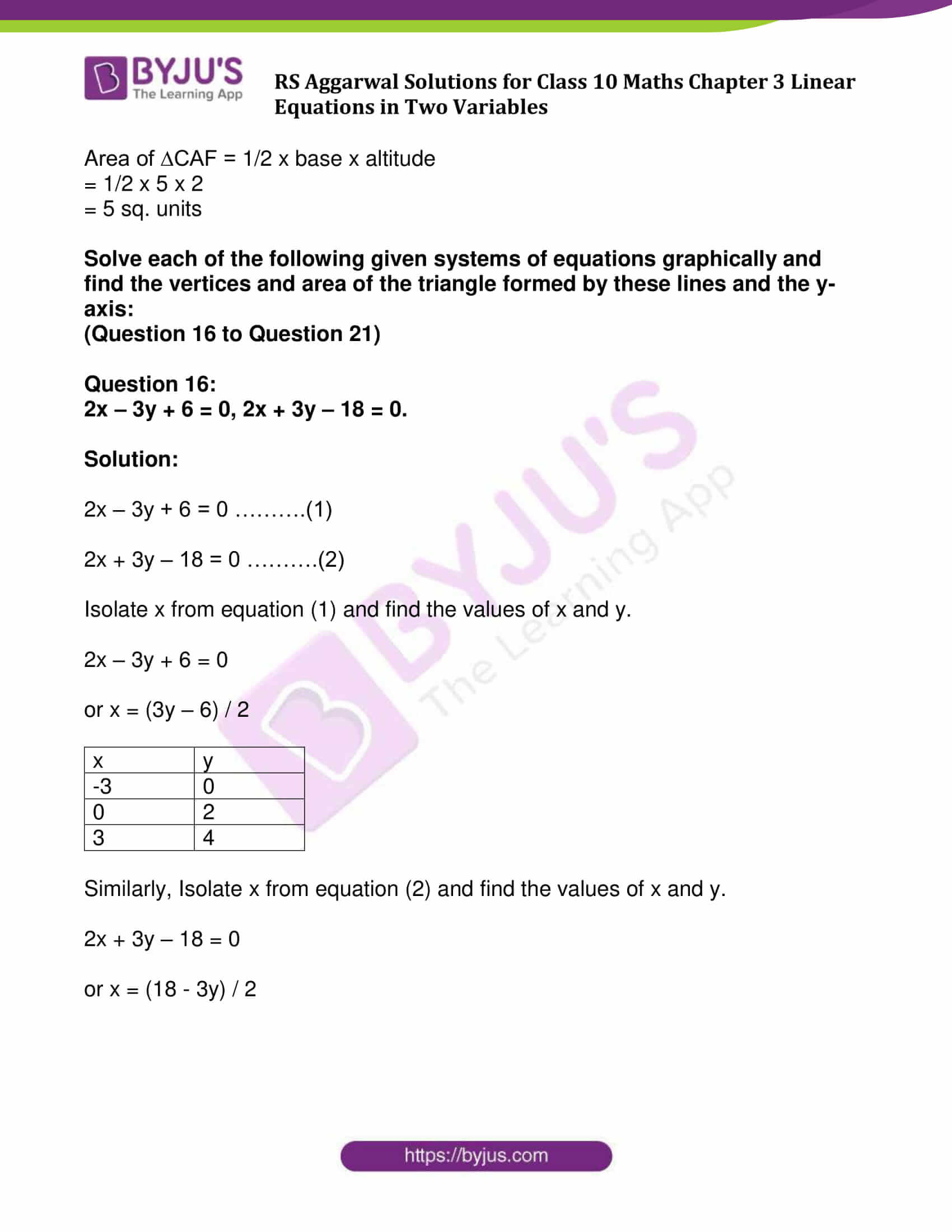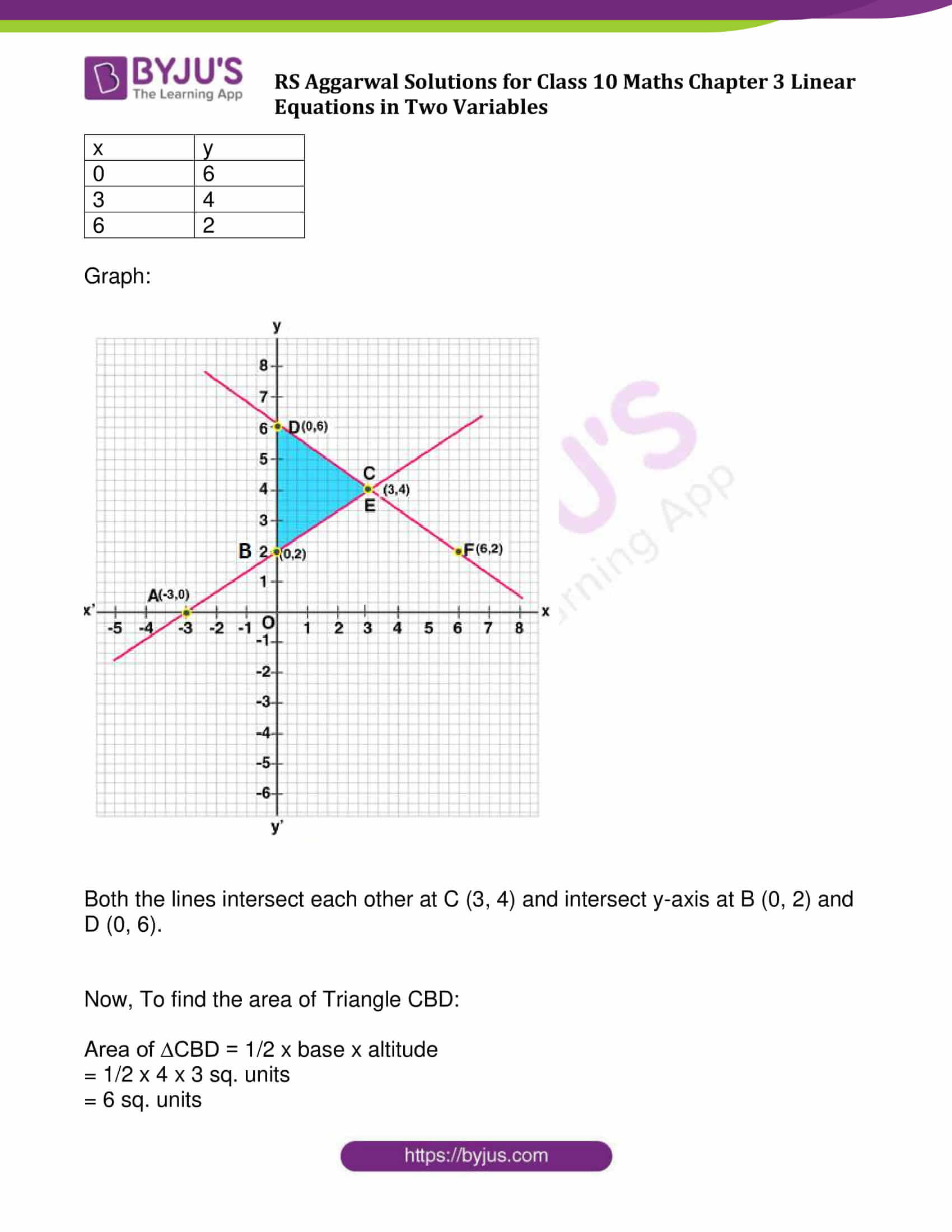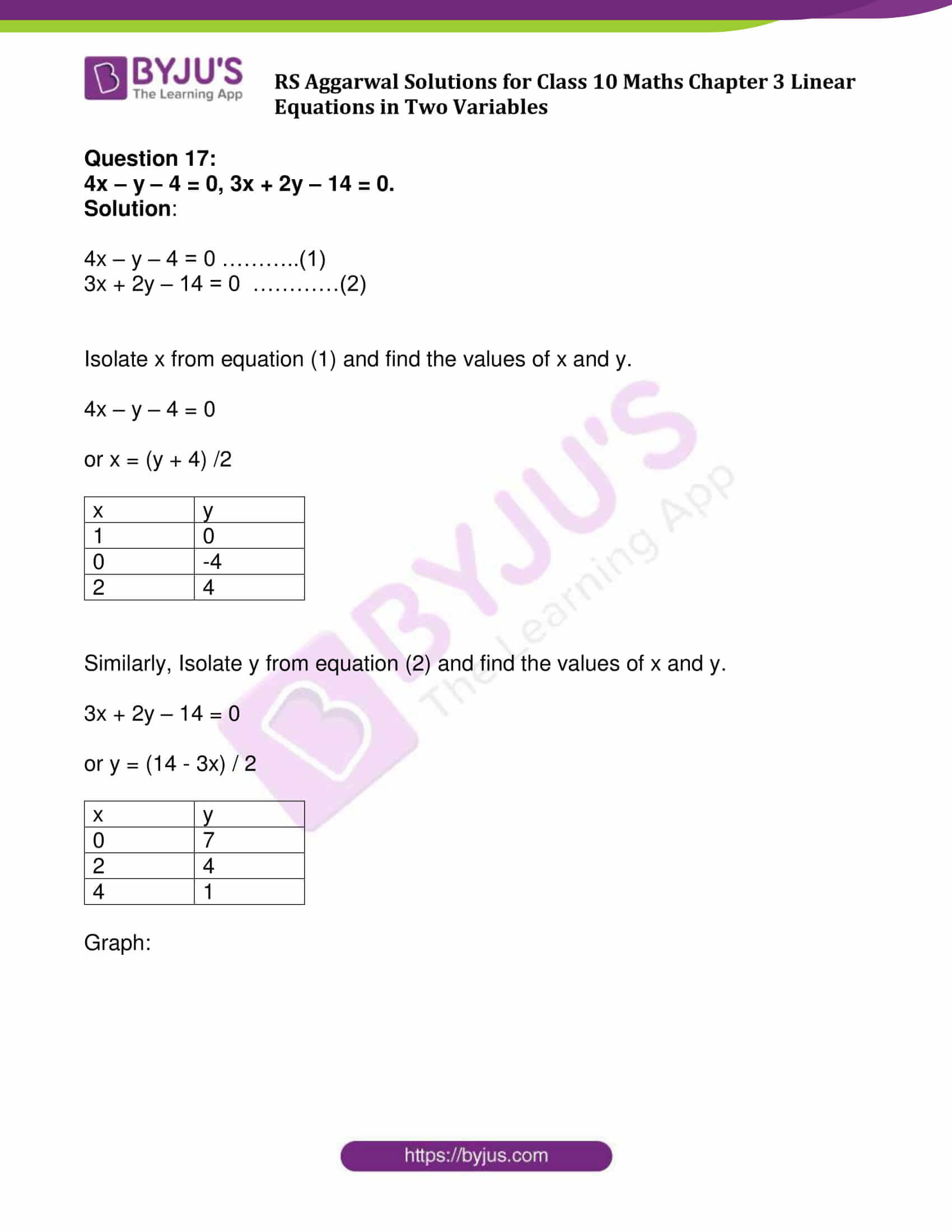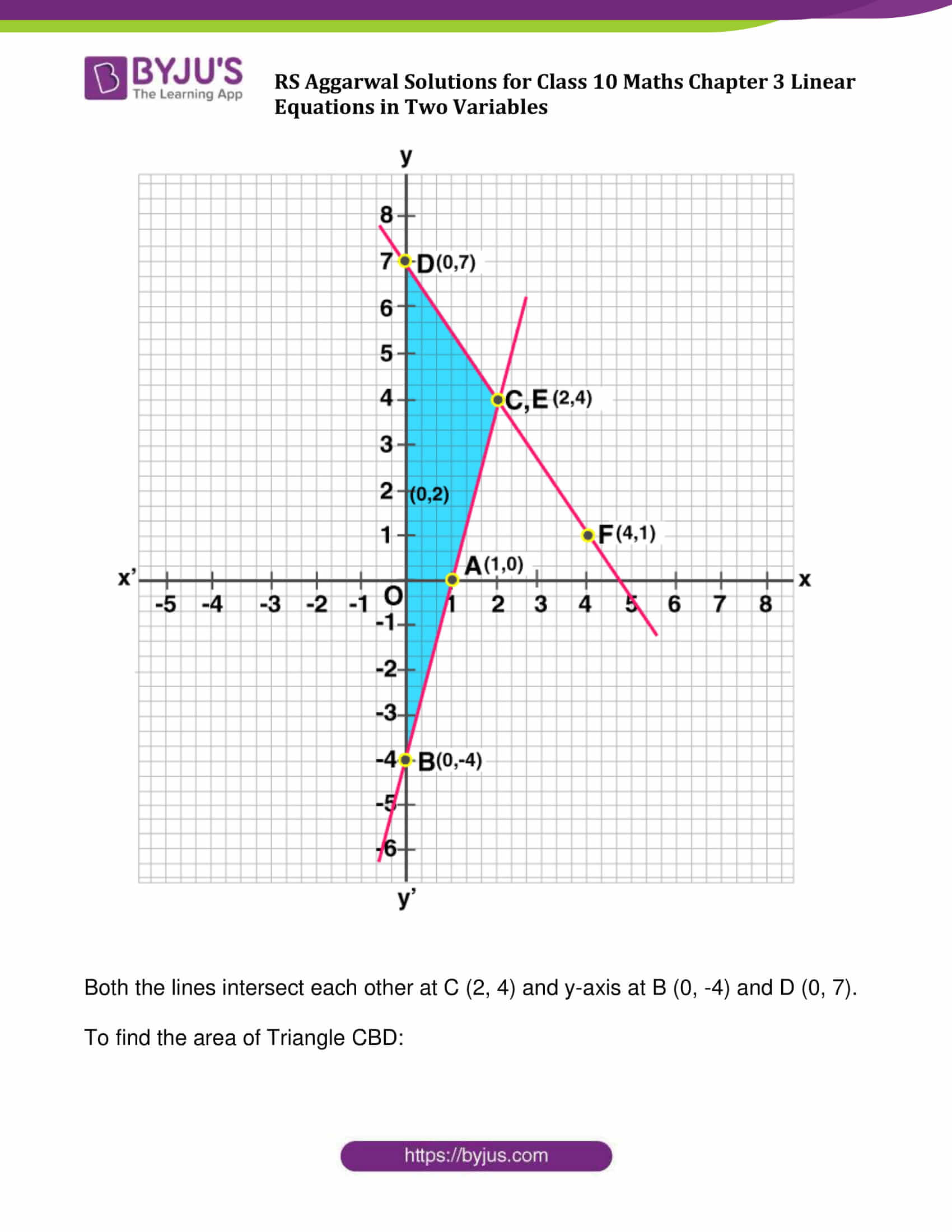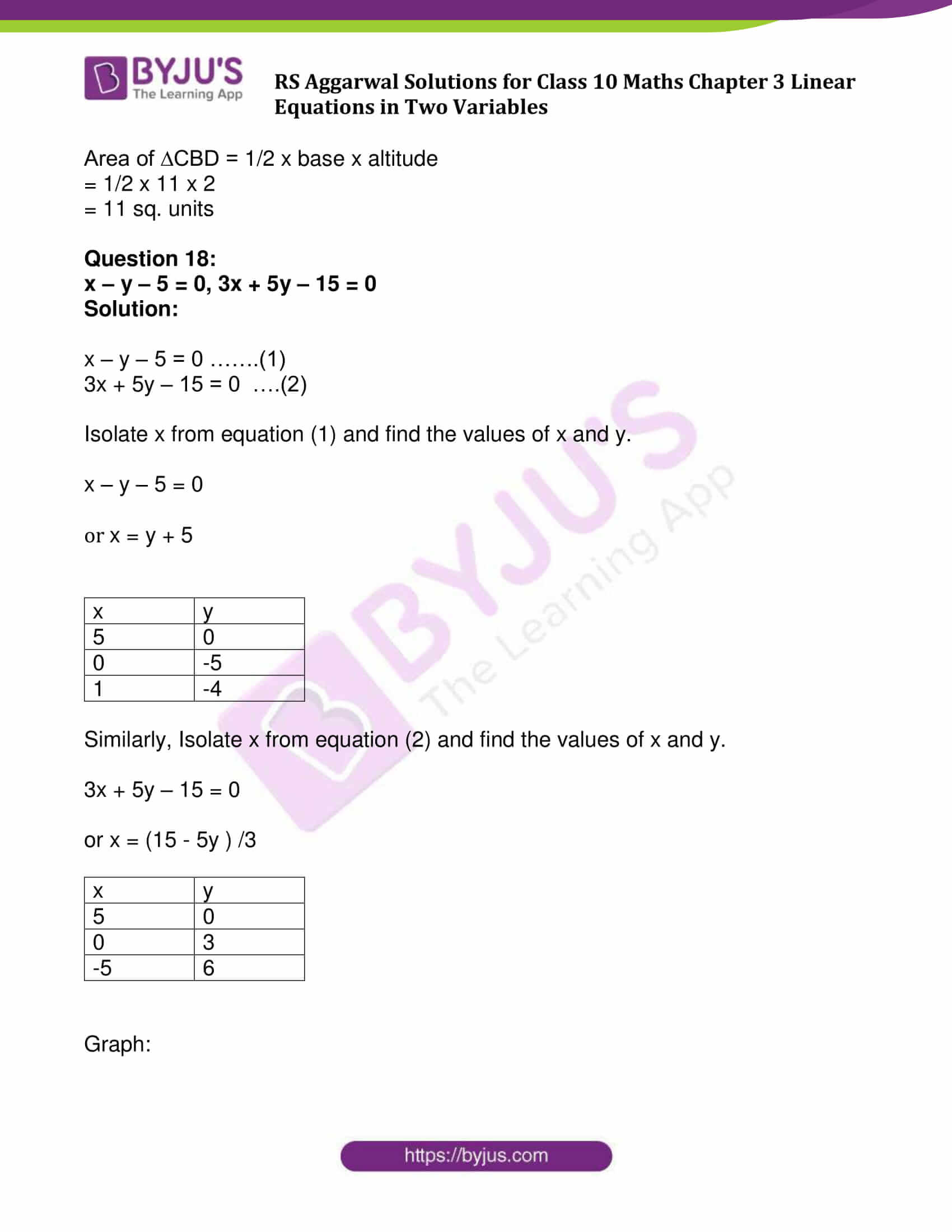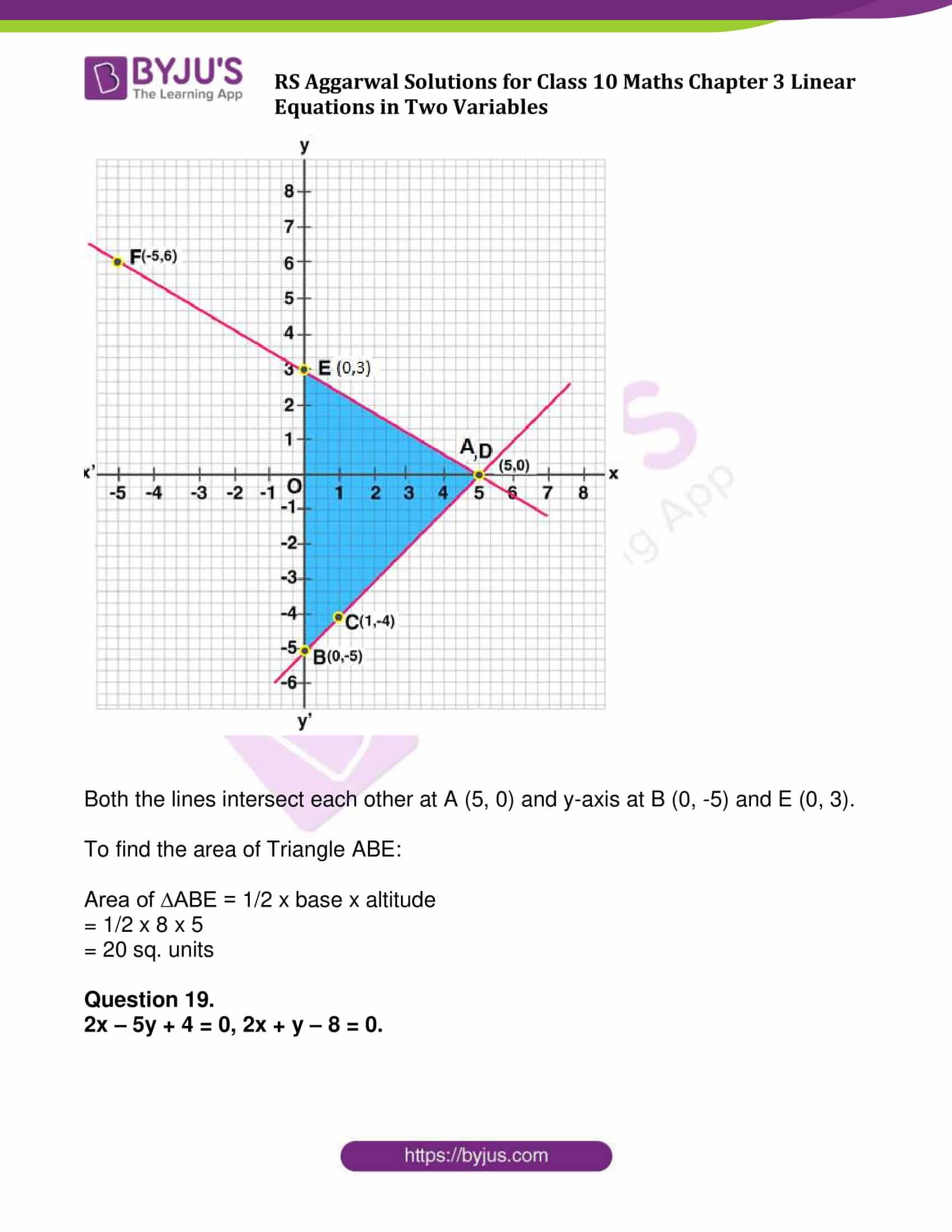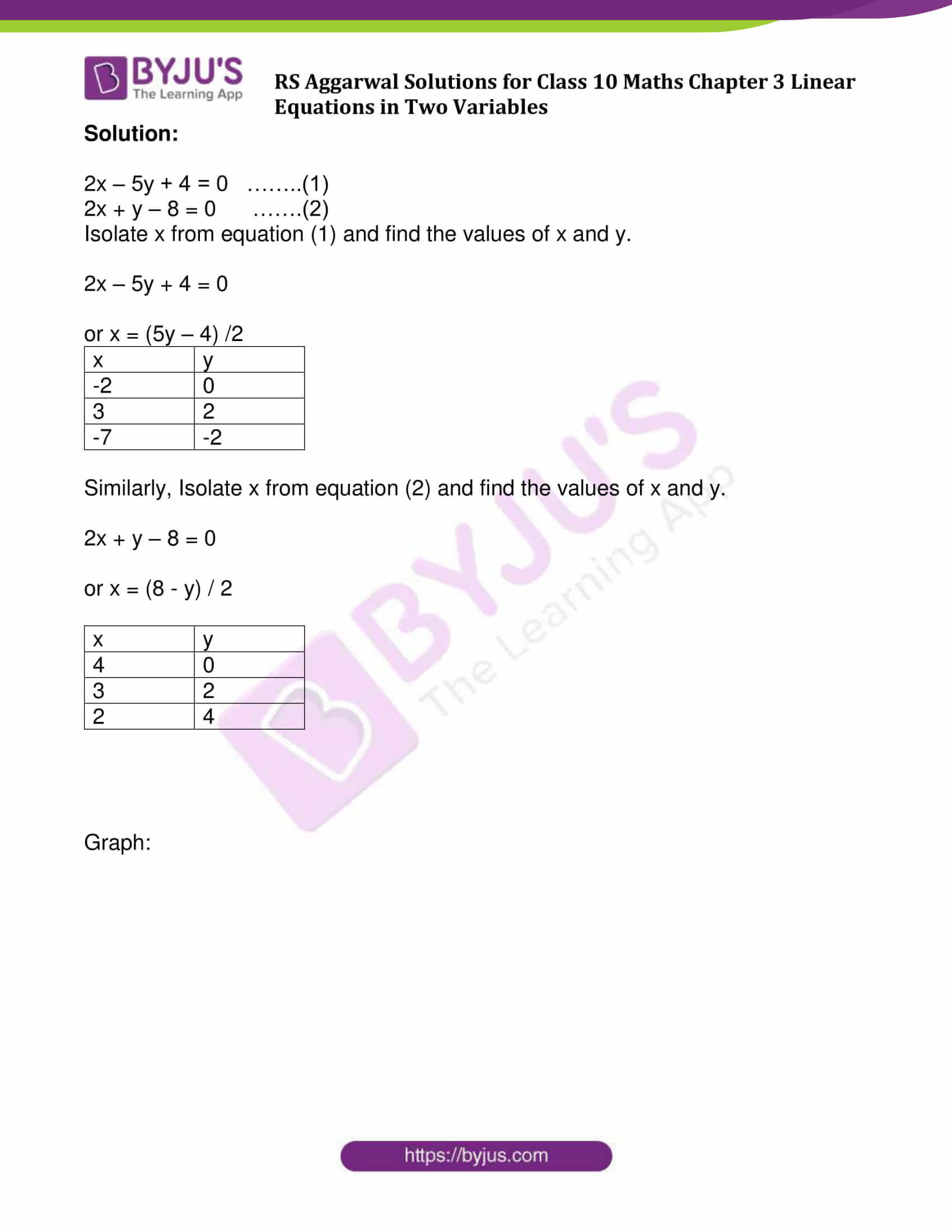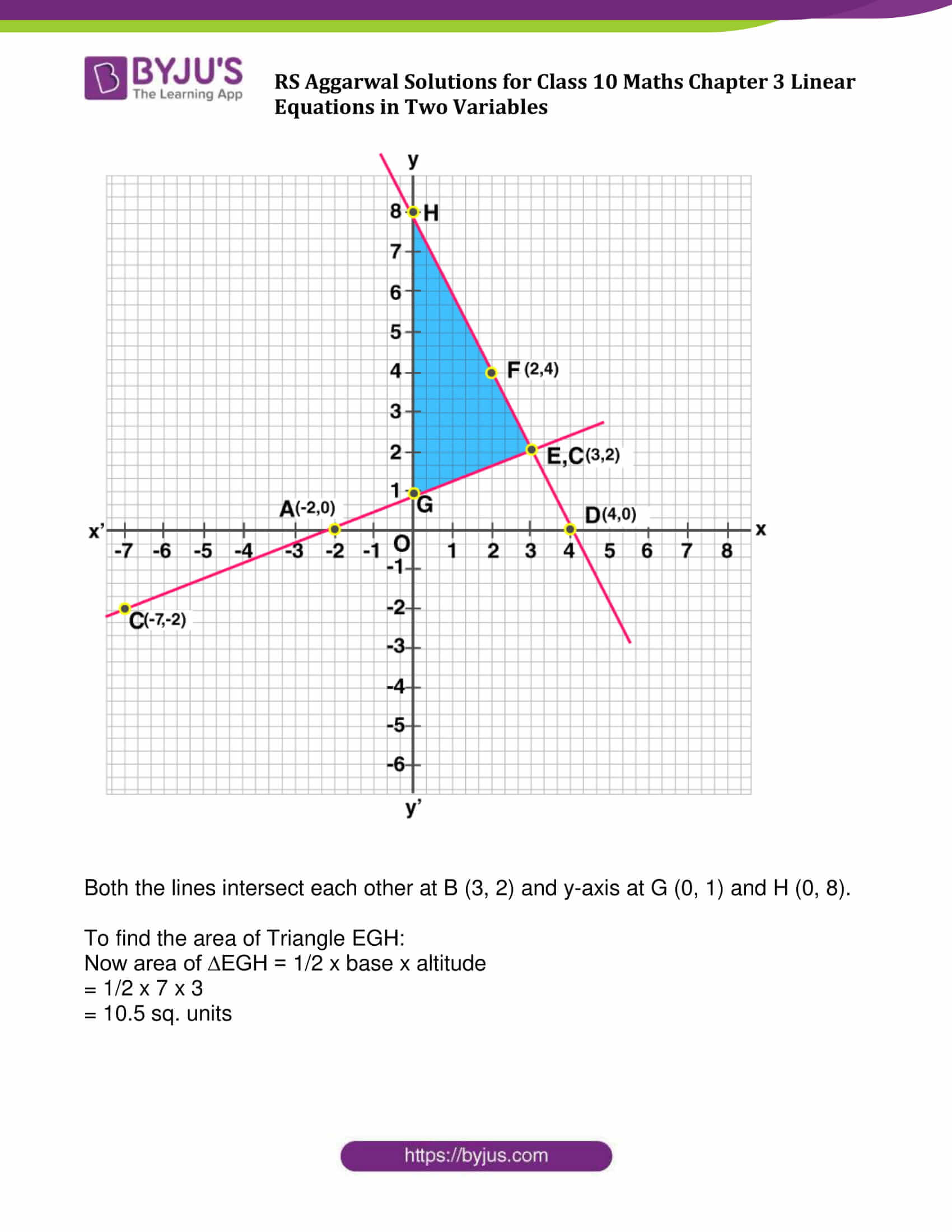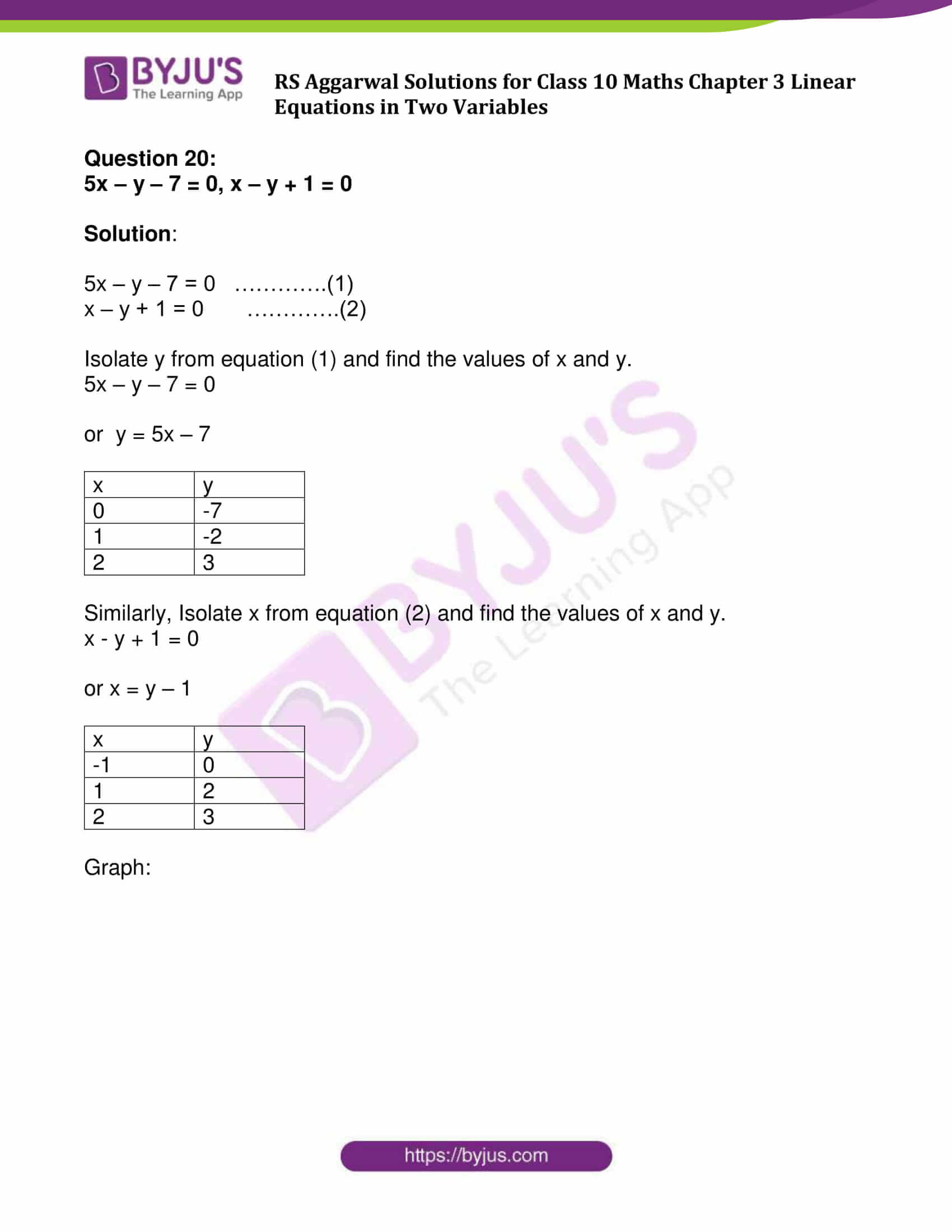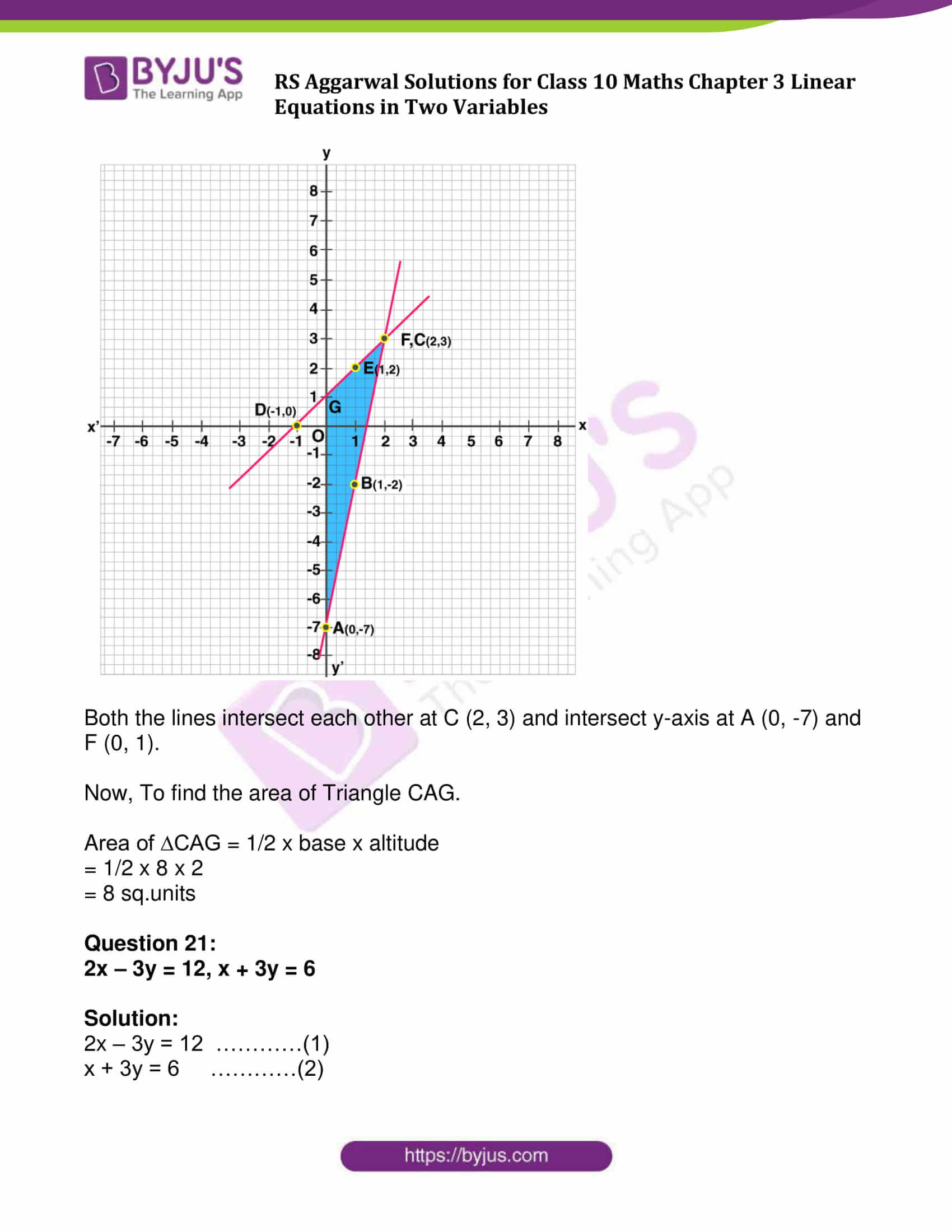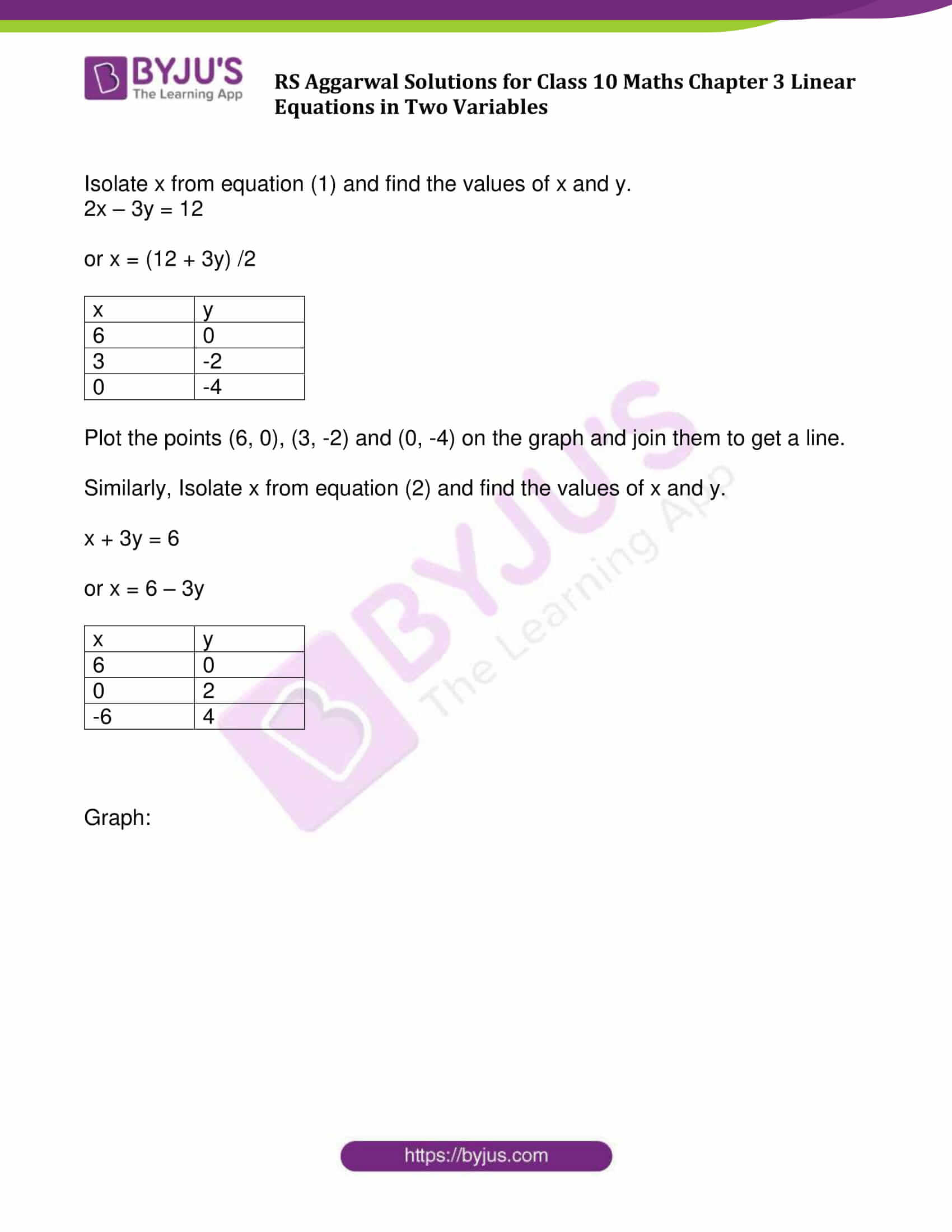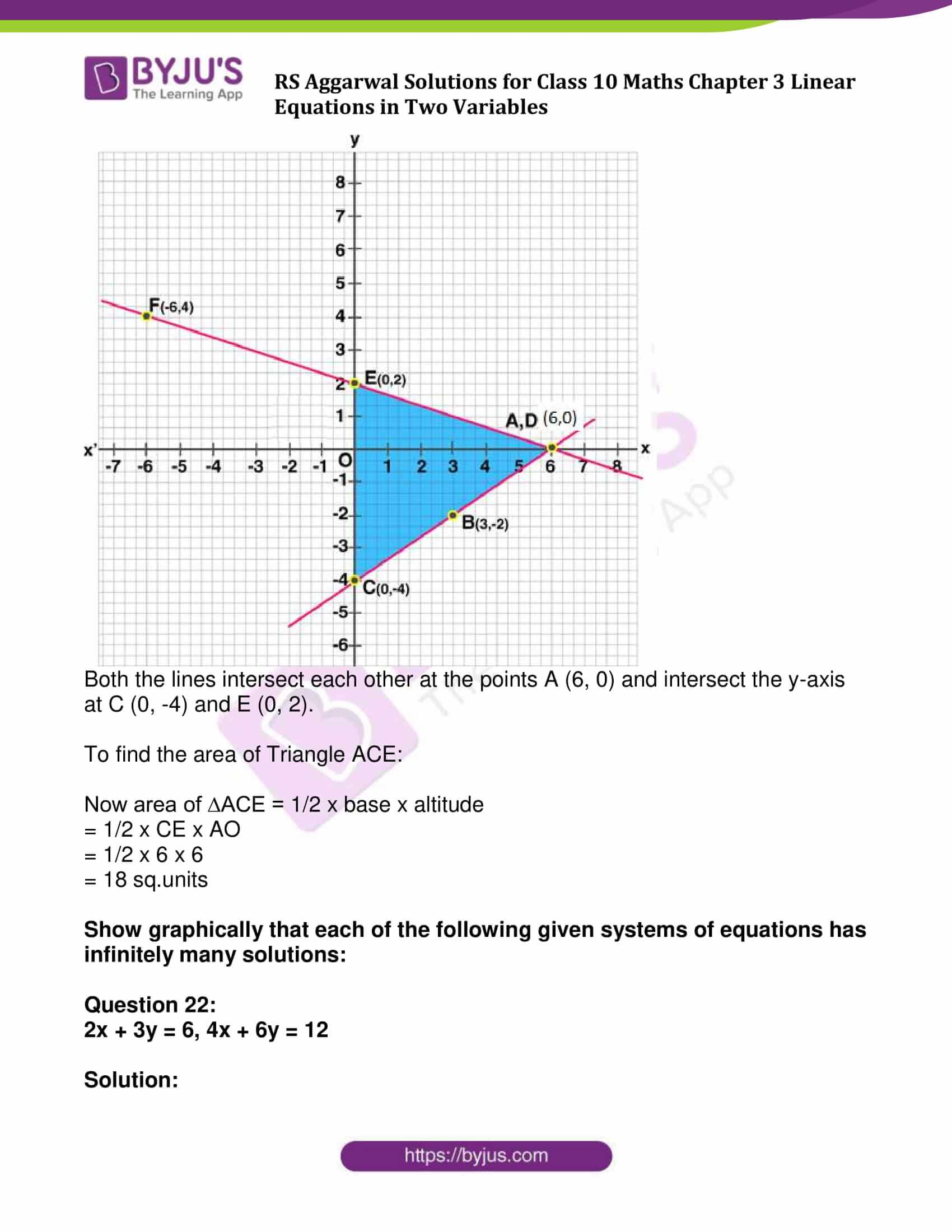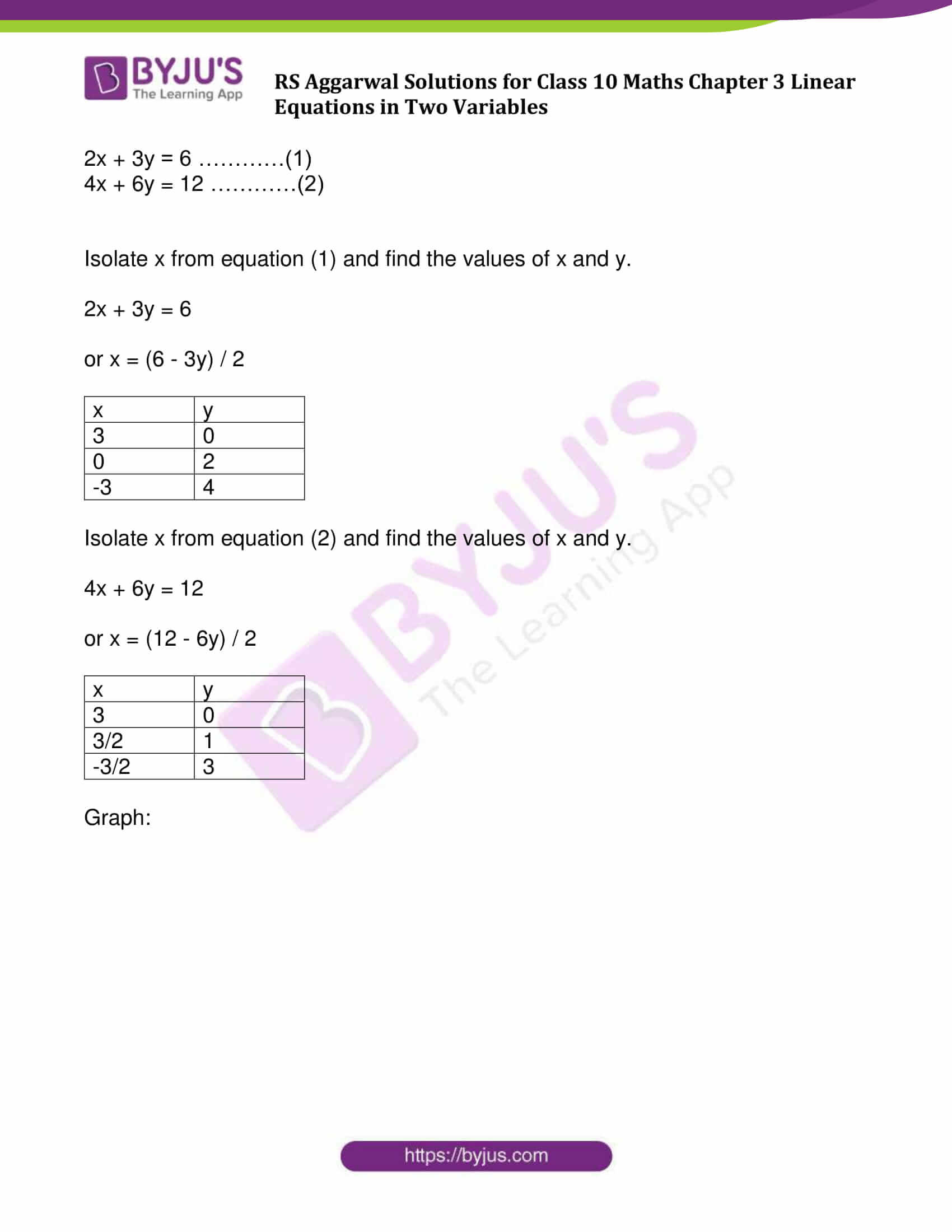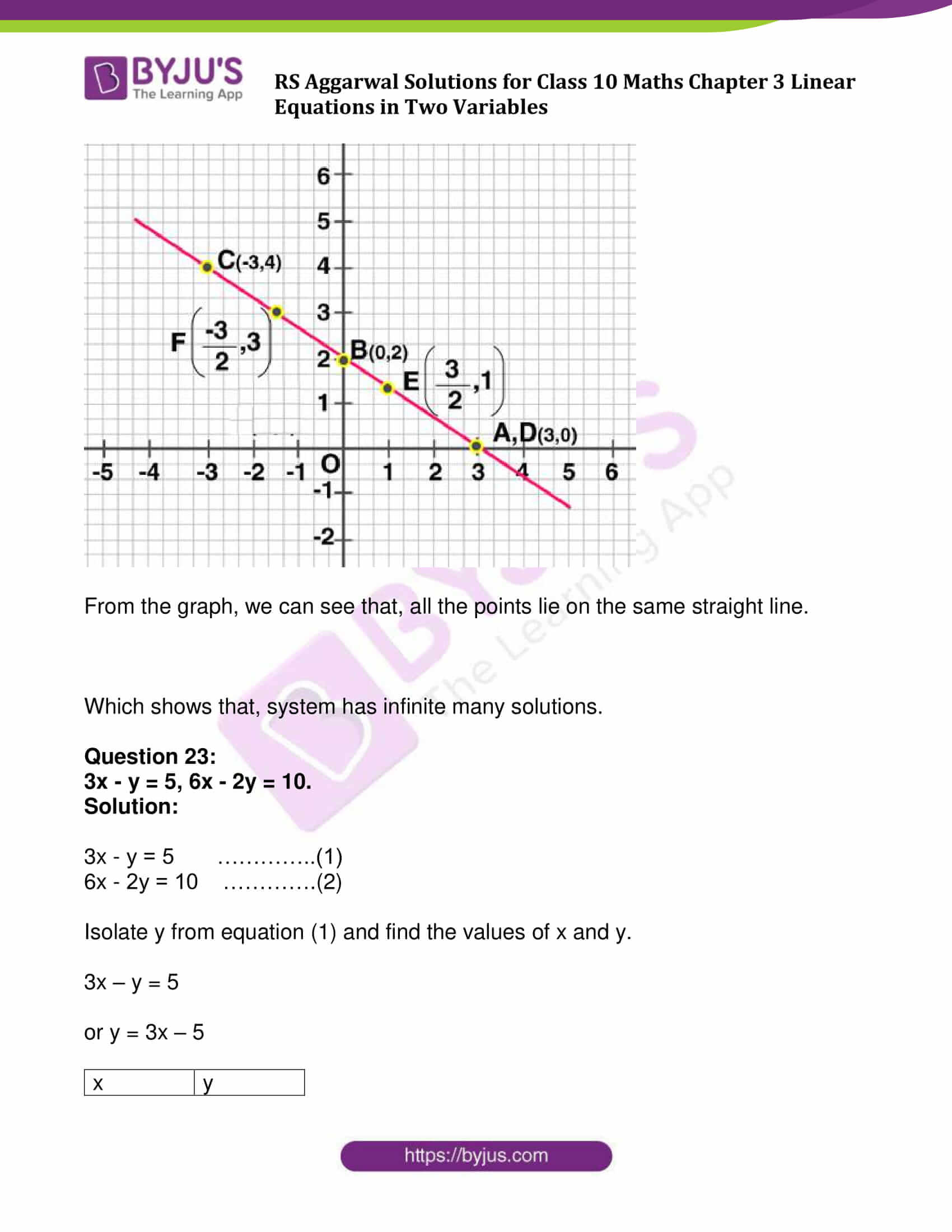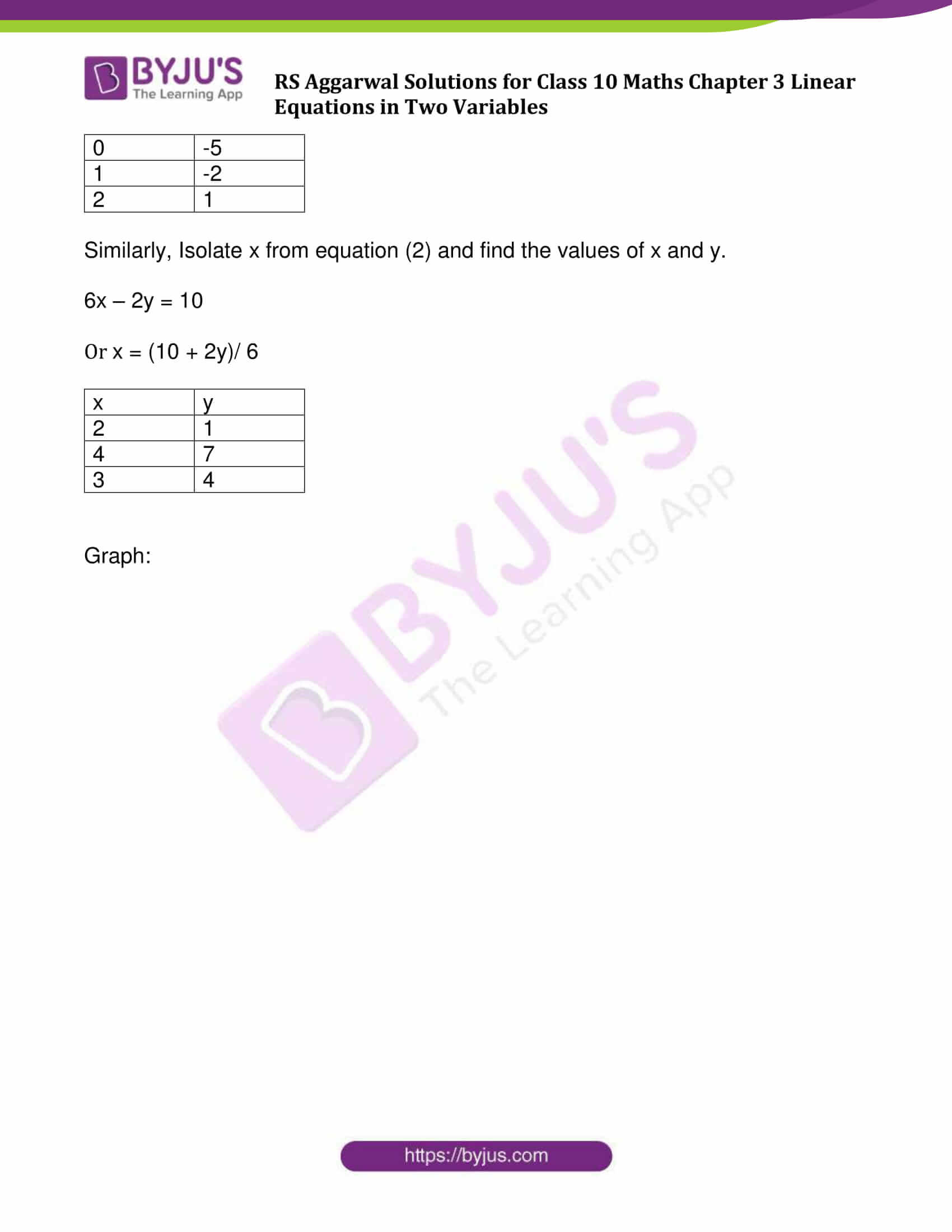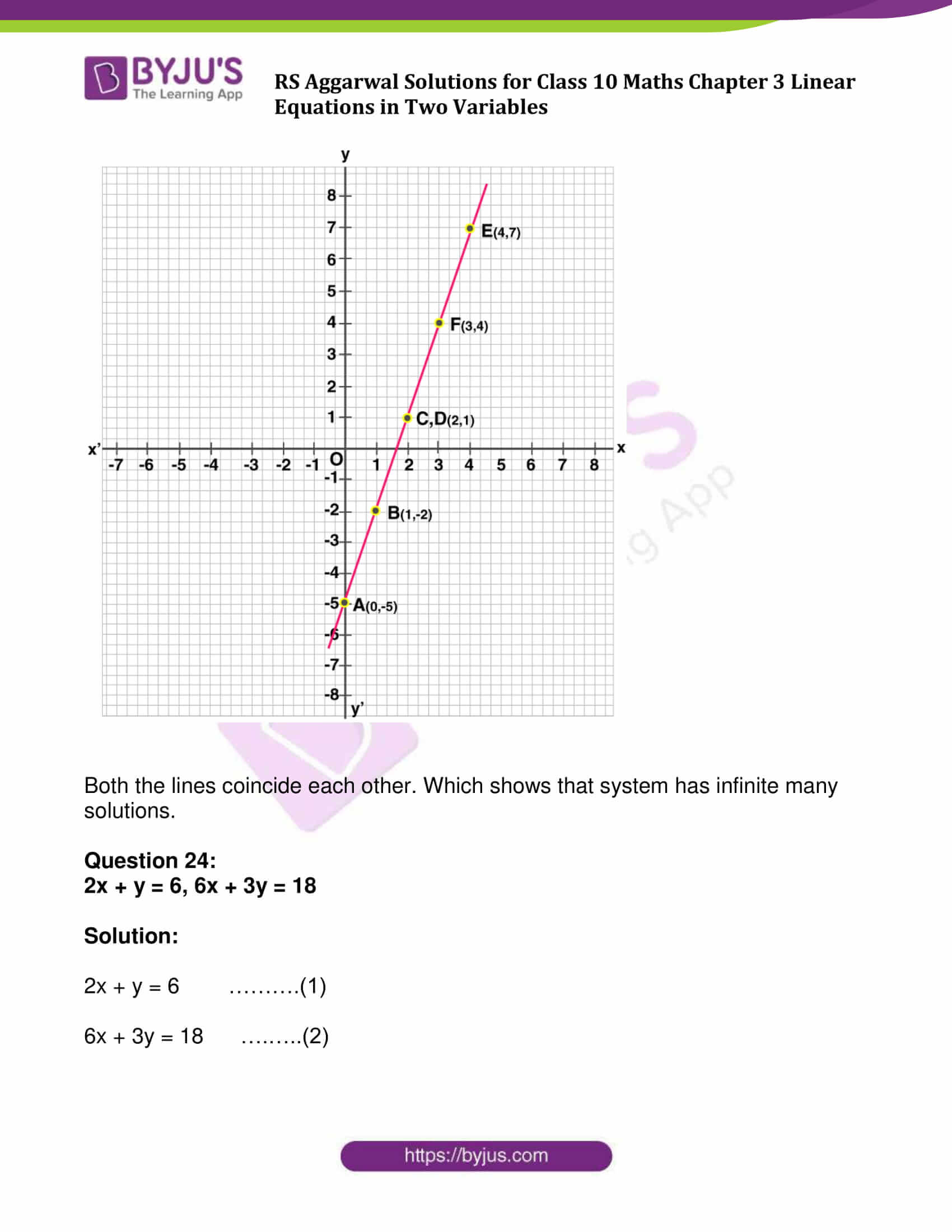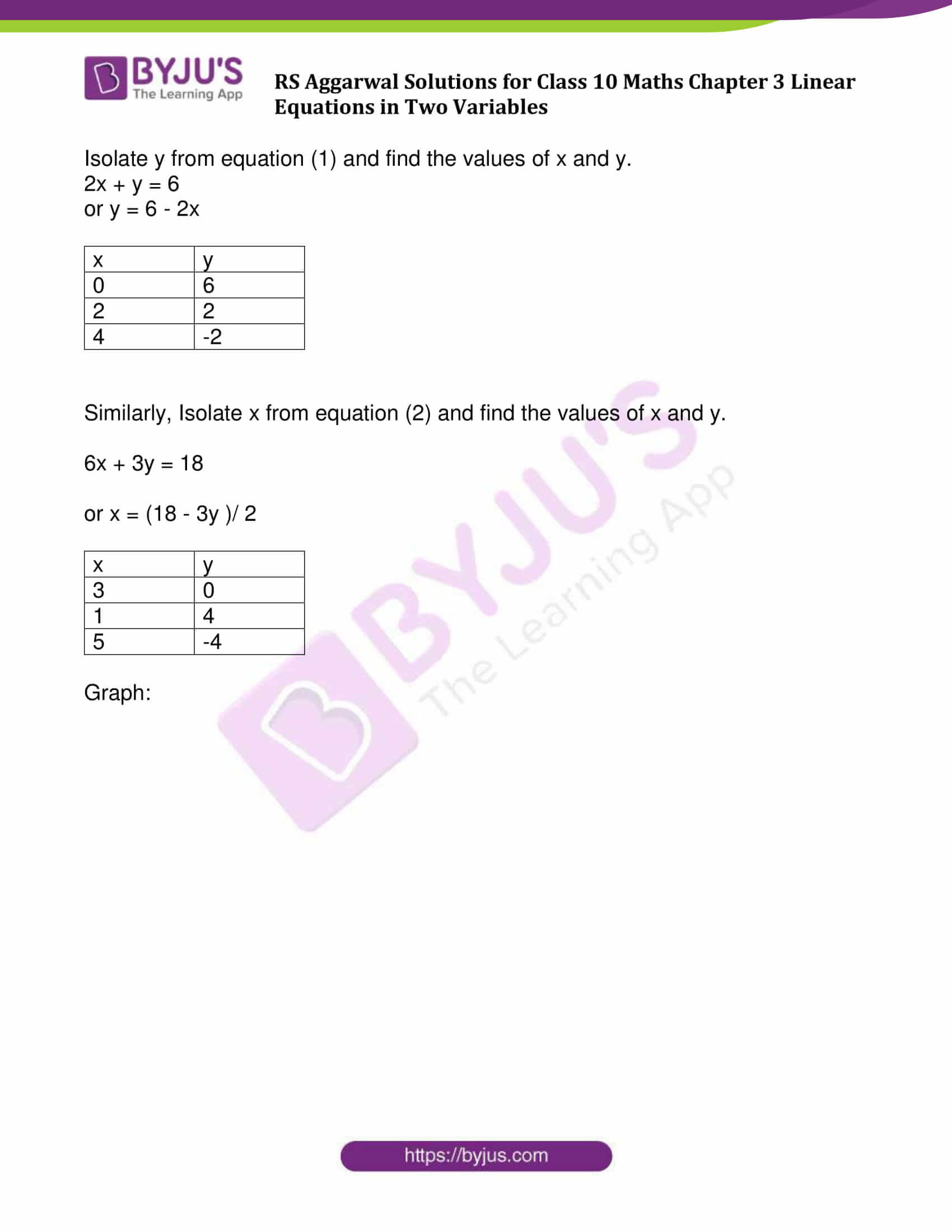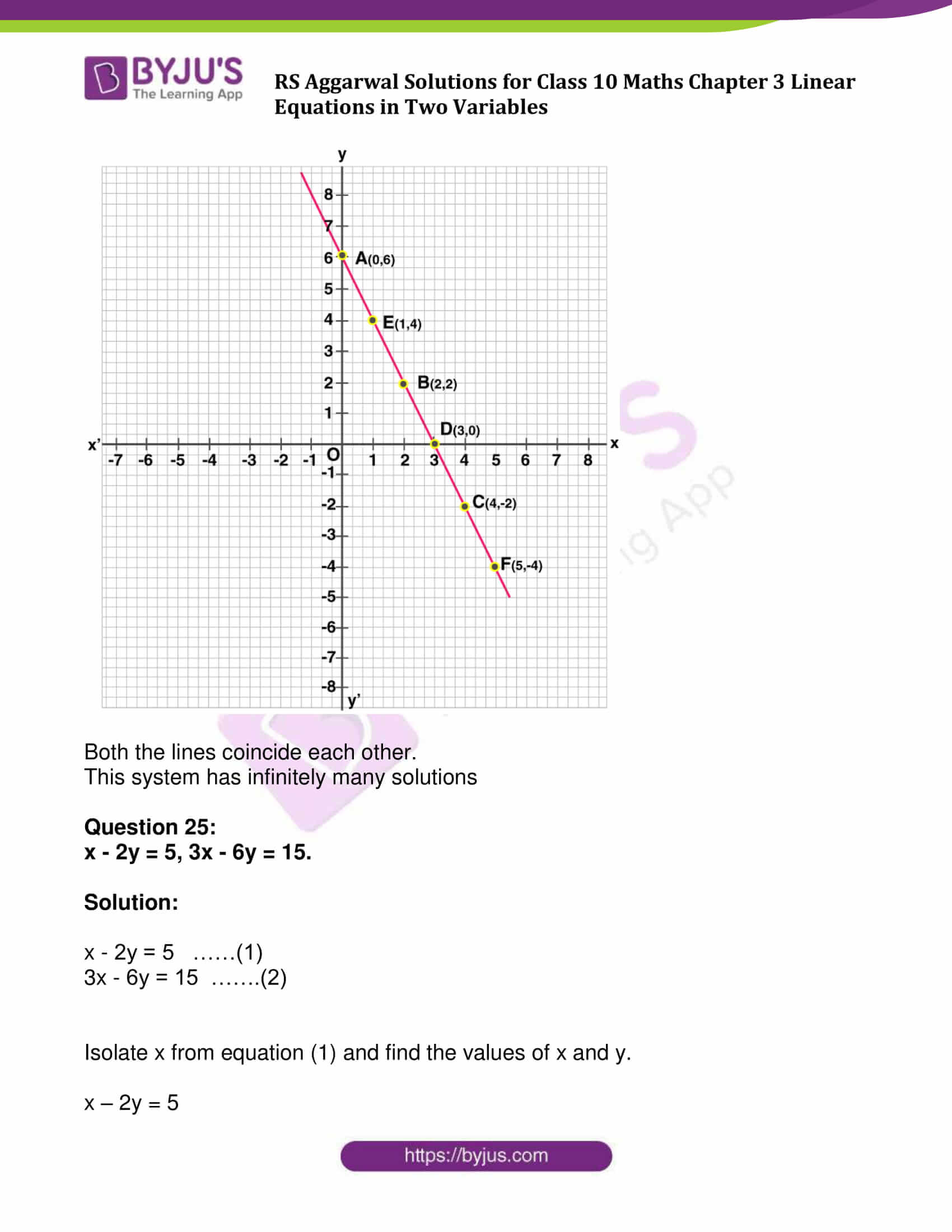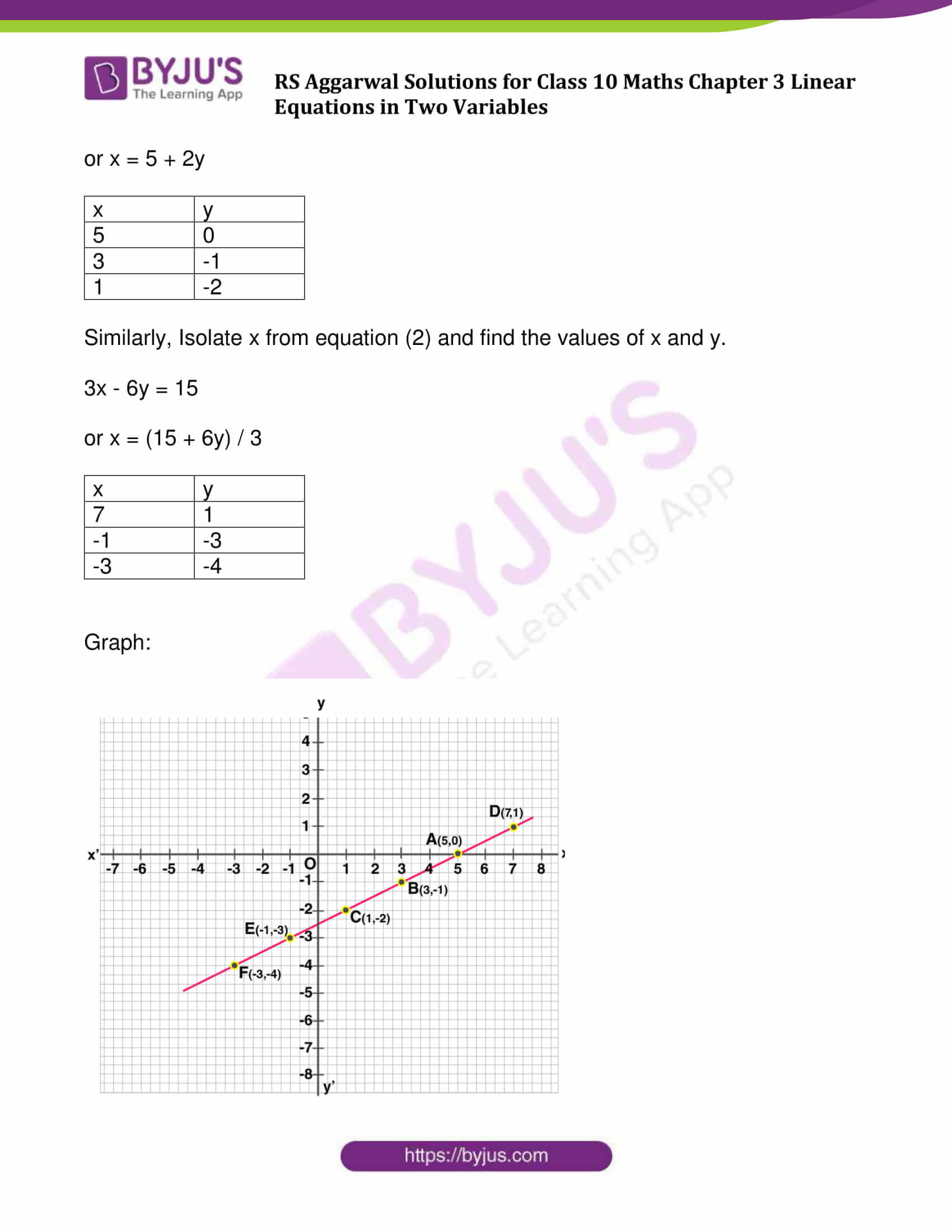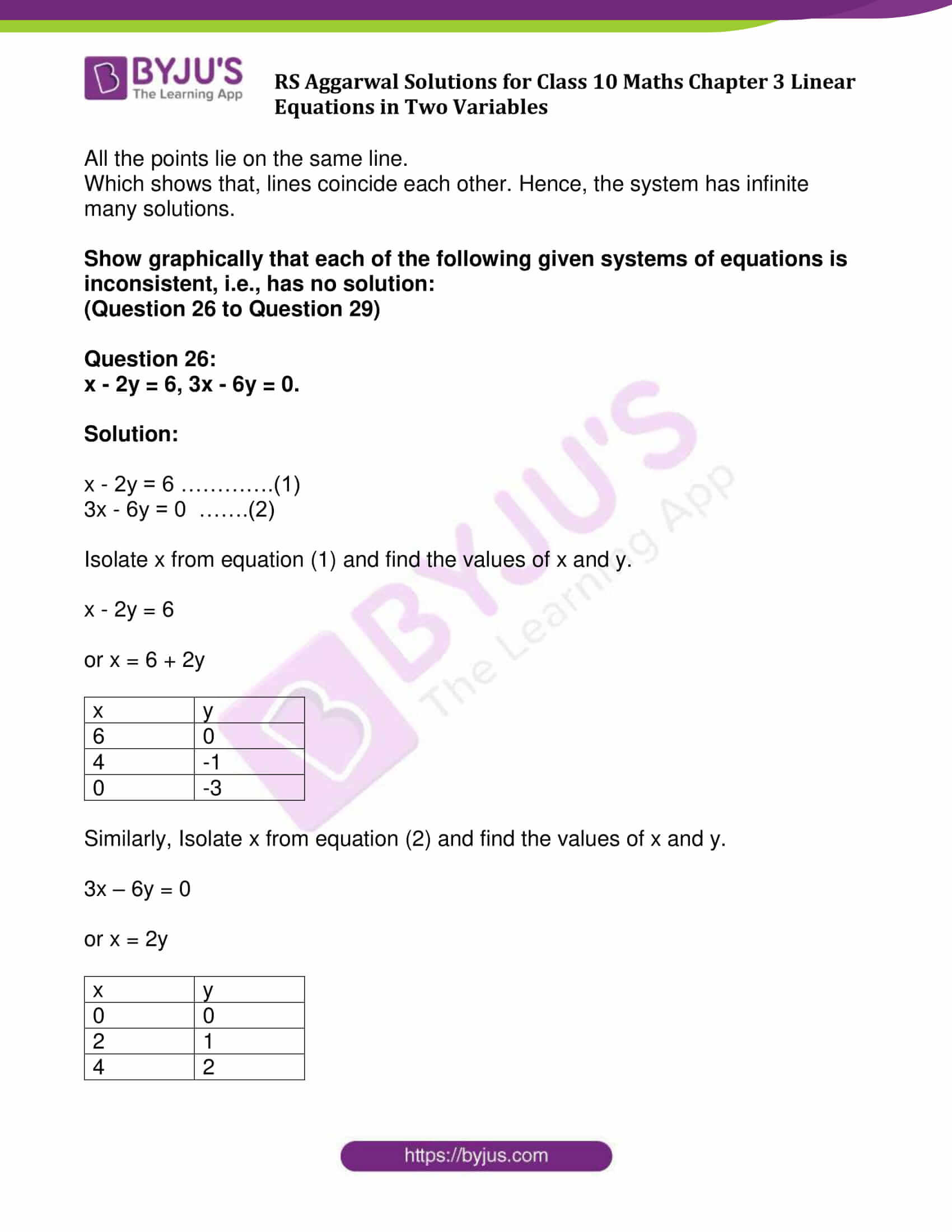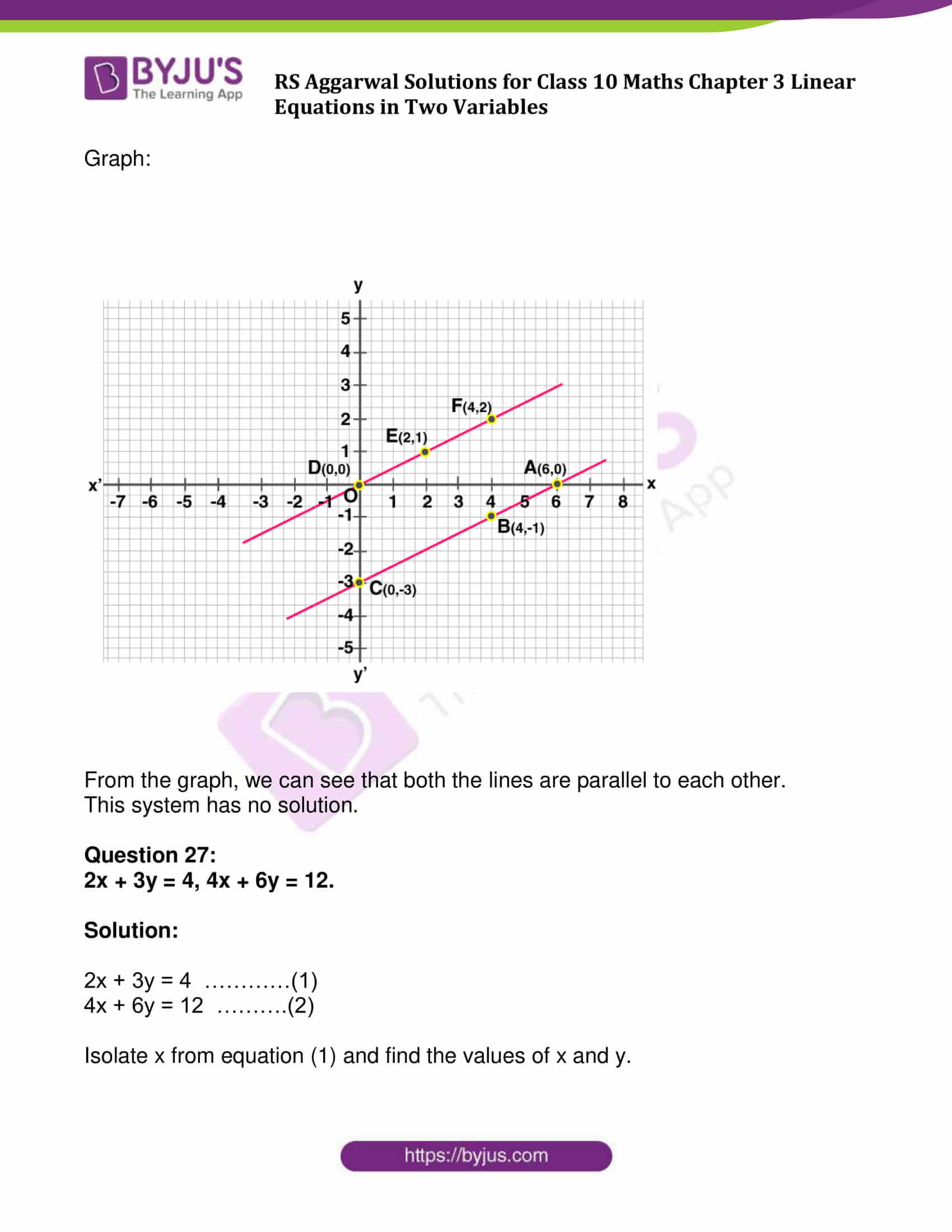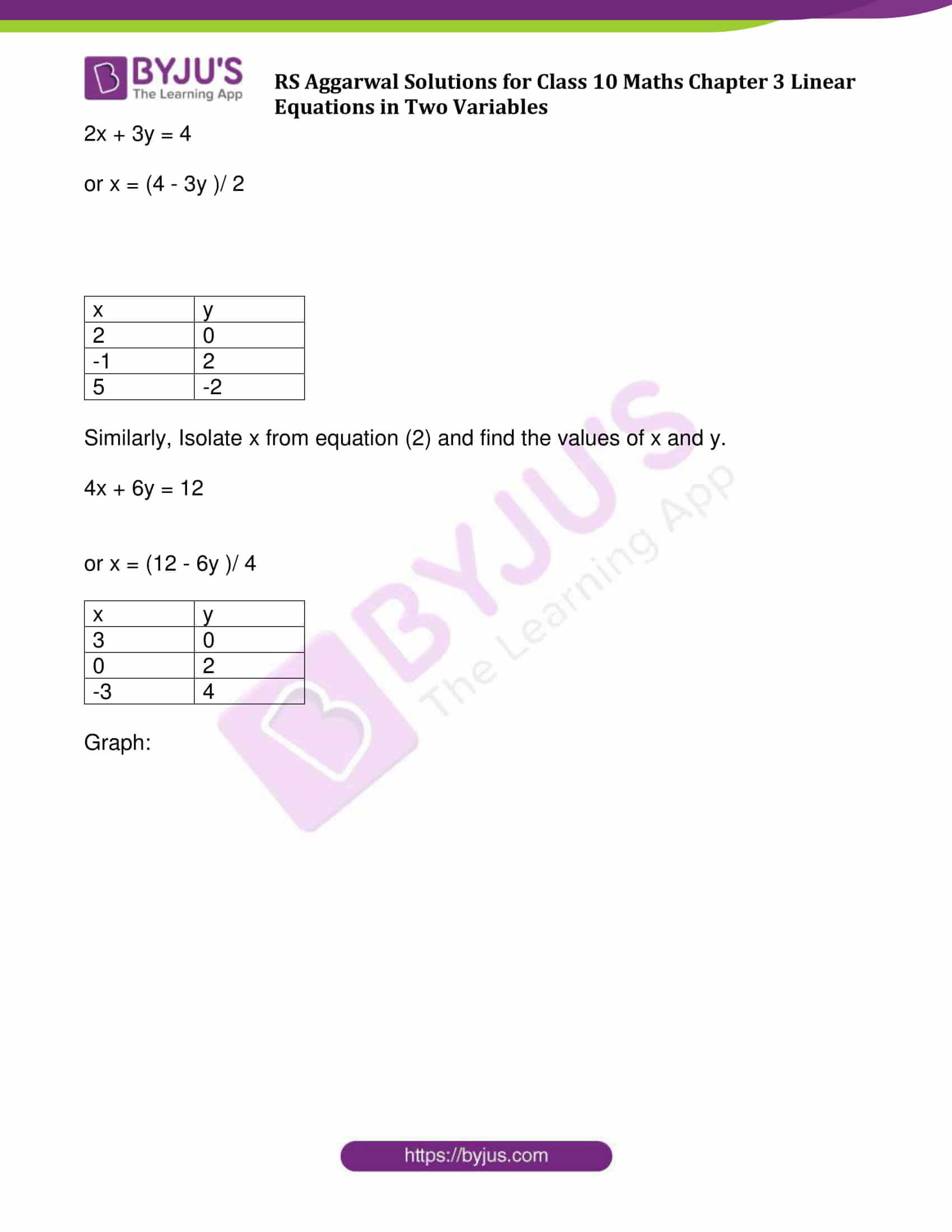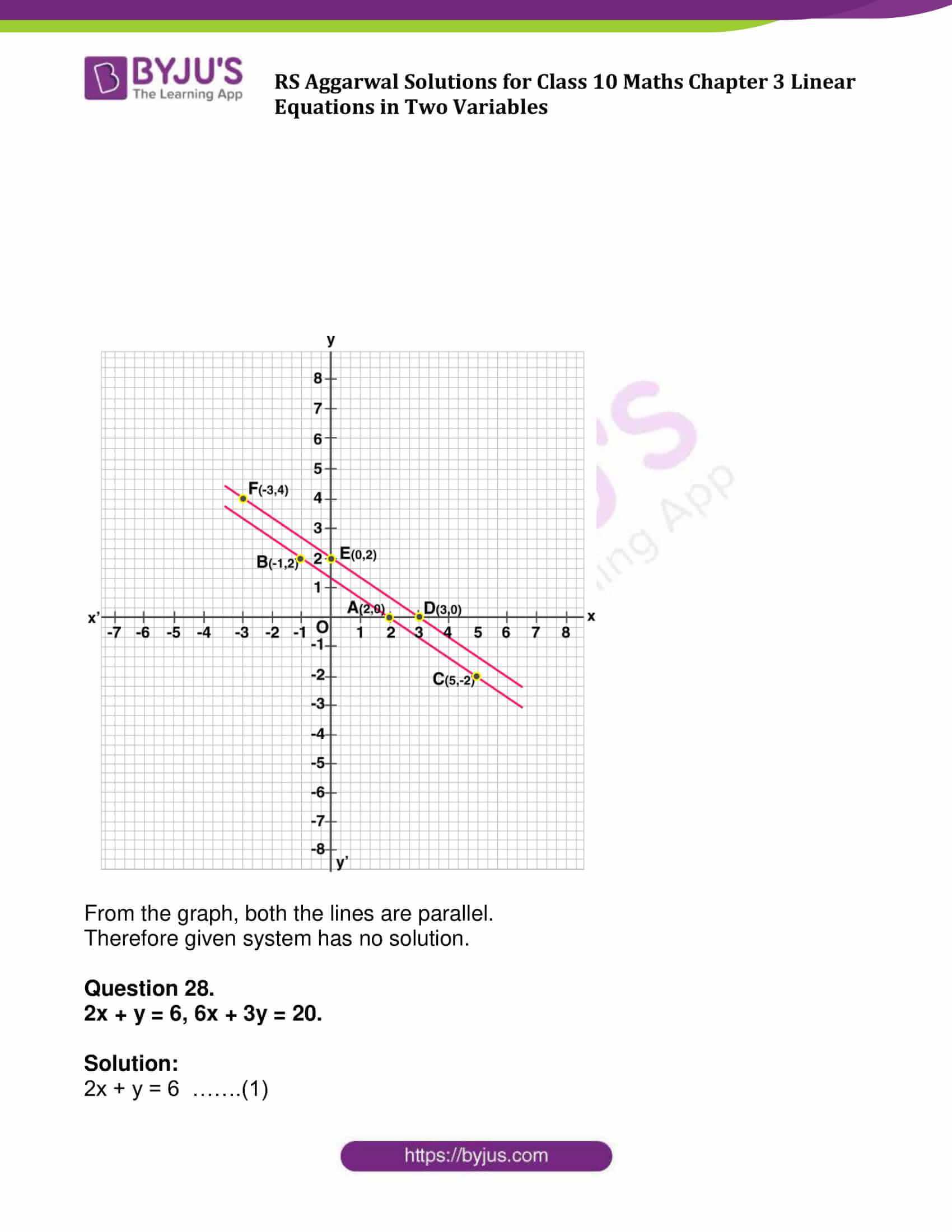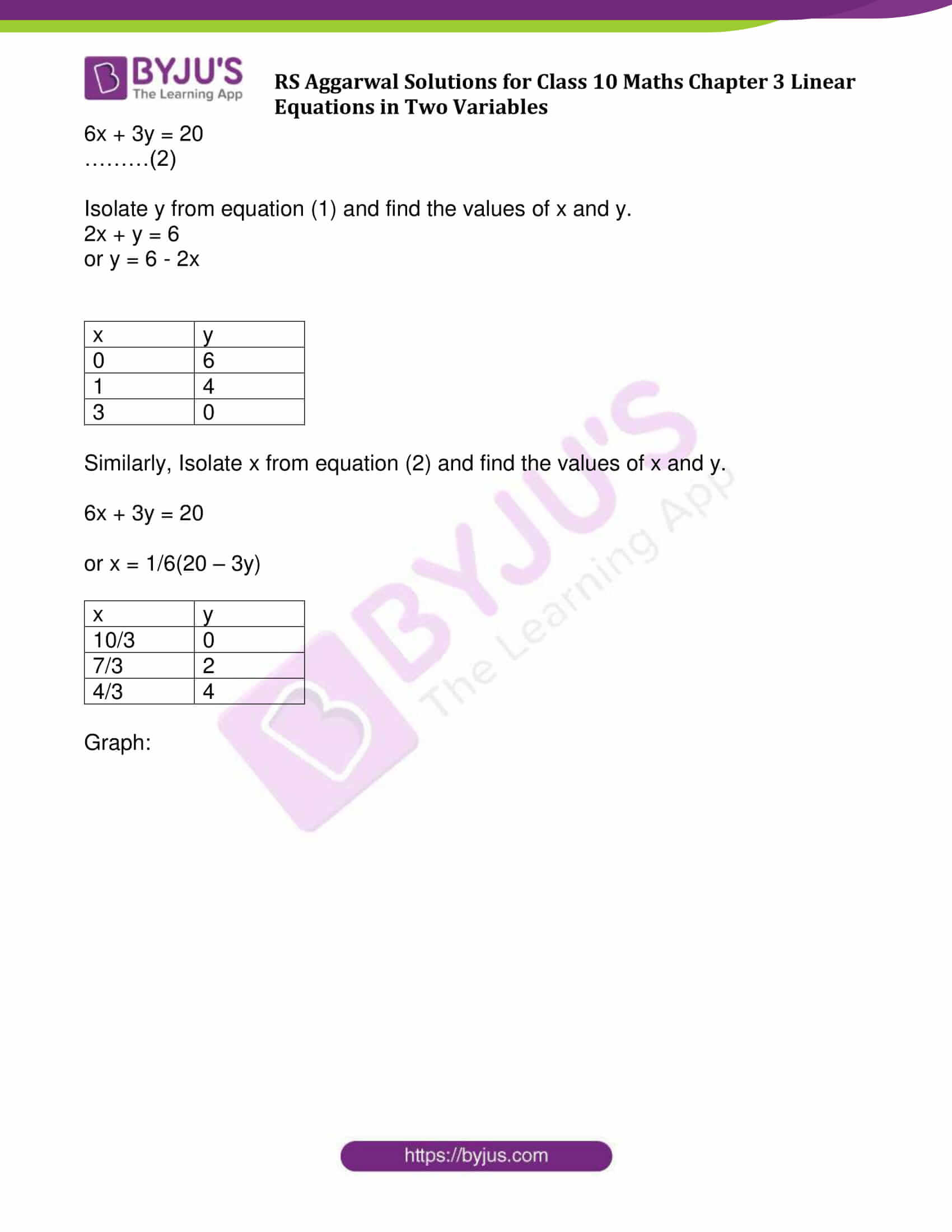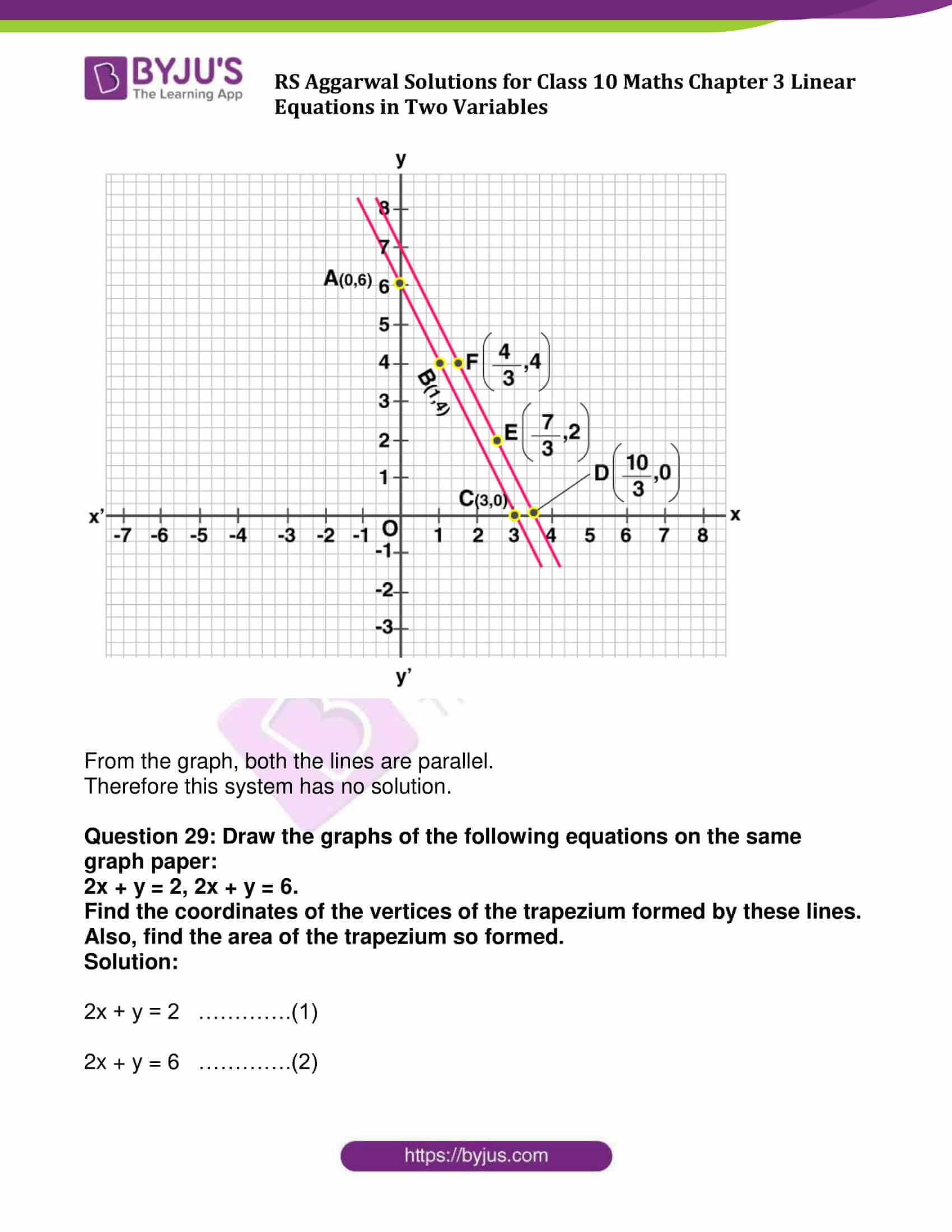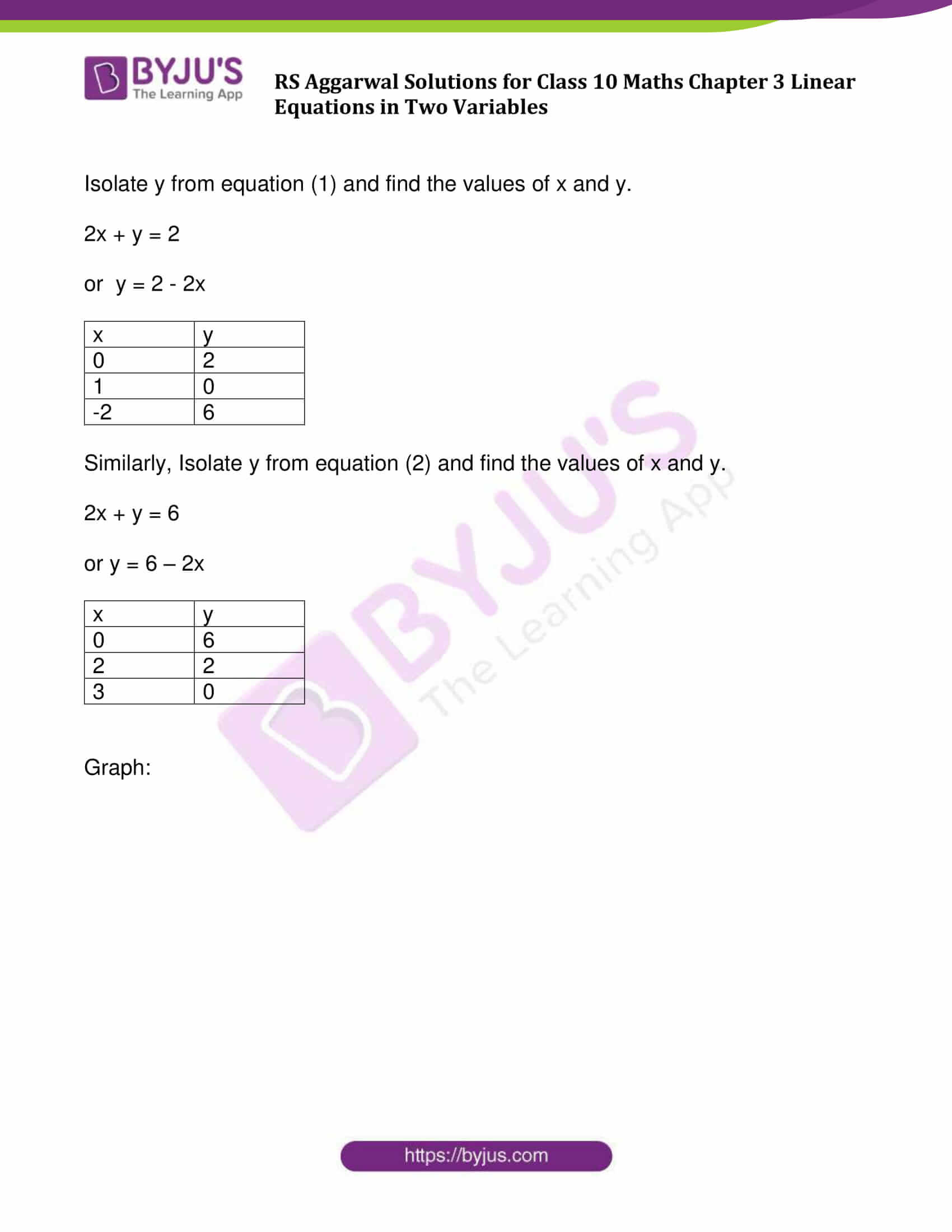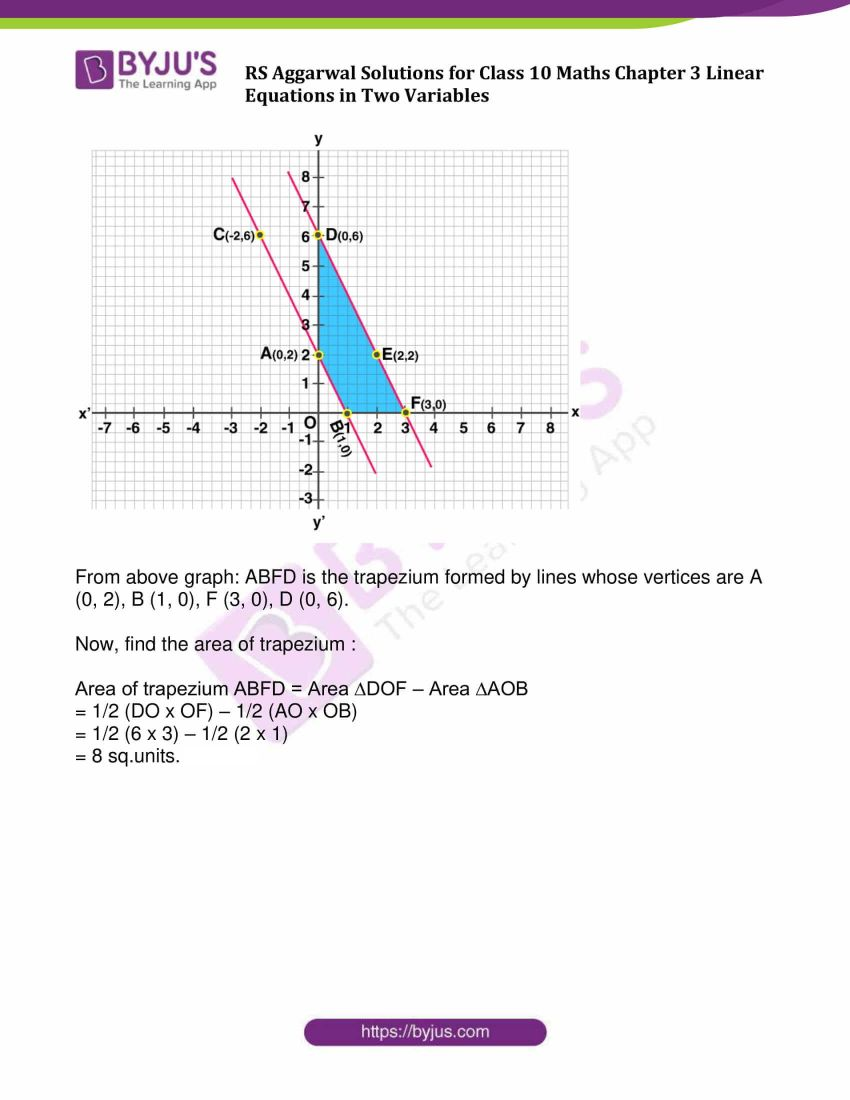### Access other exercise solutions of Class 10 Maths Chapter 3 Linear Equations in Two Variables

Exercise 3B Solutions: 20 Questions (Short Answers)

Exercise 3C Solutions: 13 Questions (Long Answers)

Exercise 3D Solutions: 14 Questions (Short Answers)

Exercise 3E Solutions: 20 Questions (Long Answers)

Exercise 3F Solutions: 10 Questions (6 Short Answers and 4 Long Answers)

## Exercise 3A Page No: 87

Solve each of the following systems of equations graphically:

(Question 1 to Question 10)

Question 1:

2x + 3y = 2

x – 2y = 8

Solution:

2x + 3y = 2 …………(1)

x – 2y = 8 ..………(2)

Step 1: From Equation (1), isolate x

2x = 2 – 3y

Or x = (2 – 3y)/ 2

Choose any values for y and get corresponding values of x:

 x 1 -2 4 y 0 2 -2

Plot above points on the graph and join them.

Step 2: From equation (2)

x – 2y = 8

or x = 8 + 2y

Choose any values for y and get corresponding values of x:

 x 6 4 2 y -1 -2 -3

Plot above points on the graph and join them.

Step 3:

Graph: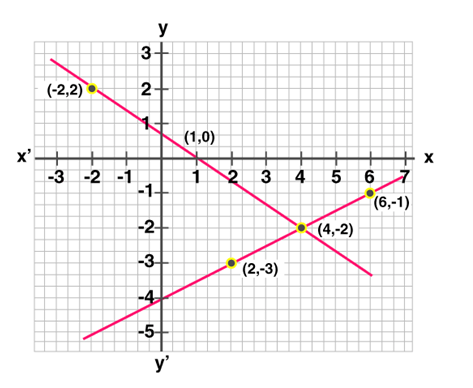Step 4: Both the lines intersect each other at point (4, -2).

So, x = 4, y = -2

Question 2:

3x + 2y = 4,

2x – 3y = 7.

Solution:

3x + 2y = 4 …..(1)

2x – 3y = 7 ……(2)

Isolate x from equation (1) and find the values of x and y.

3x + 2y = 4

or x = (4 – 2y)/ 3

 x 0 2 4 y 2 -1 -4

Similarly, Isolate x from equation (2) and find the values of x and y.

2x – 3y = 7

x = (3y+ 7) / 2

 x 5 2 -1 y 1 -1 -3

Graph: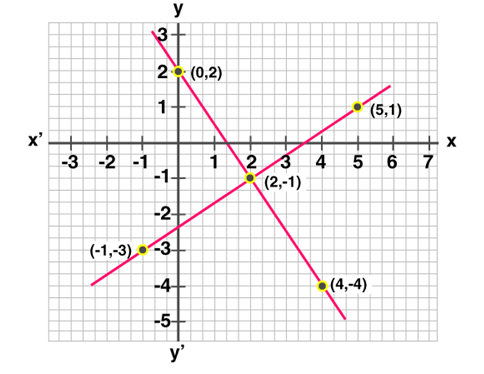Both the lines intersect each other at point (2, -1).

So, x = 2, y = -1

Question 3:

2x + 3y = 8,

x – 2y + 3 = 0.

Solution:

2x + 3y = 8 …(1)

x – 2y + 3 = 0 …(2)

Isolate x from equation (1) and find the values of x and y.

2x + 3y = 8

2x = 8 – 3y

x = ( 8 – 3y )/2

 x 4 1 -2 y 0 2 4

Similarly, Isolate x from equation (2) and find the values of x and y.

x – 2y + 3 = 0

or x = 2y – 3

 x -3 -1 1 y 0 1 2

Graph: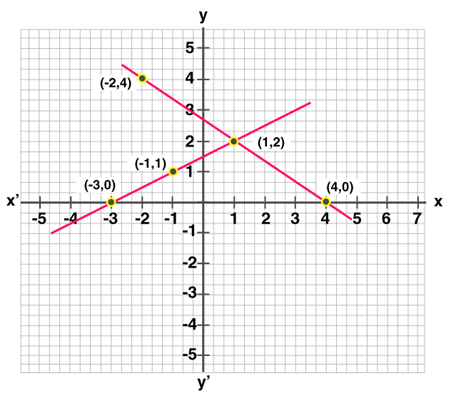Both the lines intersect each other at the point (1, 2).

So, x = 1, y = 2

Question 4:

2x – 5y + 4 = 0,

2x + y – 8 = 0.

Solution:

2x – 5y + 4 = 0 …..(1)

2x + y – 8 = 0 …..(2)

Isolate x from equation (1) and find the values of x and y.

2x – 5y + 4 = 0

or 2x = 5y – 4

x = (5y – 4)/ 2

 x -2 3 8 y 0 2 4

Similarly, Isolate x from equation (2) and find the values of x and y.

2x + y – 8 = 0

or y = 8 – 2x

 x 1 2 3 y 6 1 2

Graph: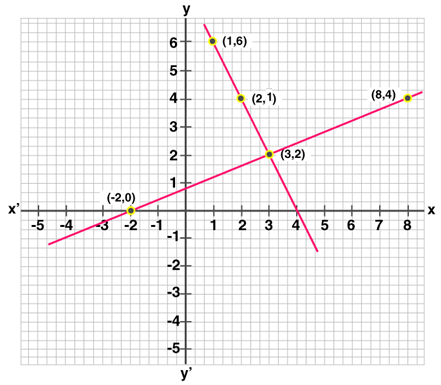Both the lines intersect each other at the point (3, 2).

So, x = 3, y = 2

Question 5:

3x + 2y = 12,

5x – 2y = 4.

Solution:

3x + 2y = 12 …..(1)

5x – 2y = 4 ….(2)

Isolate x from equation (1) and find the values of x and y.

3x + 2y = 12

3x = 12 – 2y

Or x = (12 – 2y) / 2

 x 4 2 0 y 0 3 6

Similarly, Isolate x from equation (2) and find the values of x and y.

5x – 2y = 4

5x = 4 + 2 y

or x = (4 + 2 y) / 5

 x 0 2 4 y -2 3 8

Graph: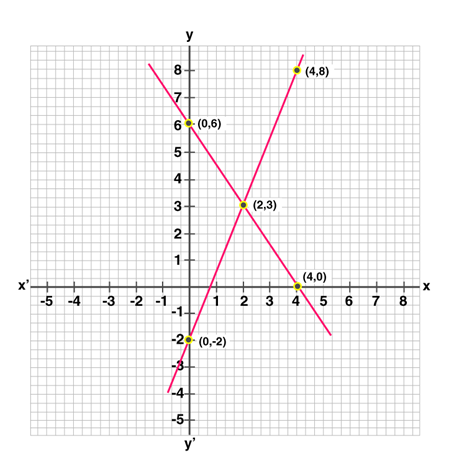Both the lines intersect each other at the point (2, 3).

So, x = 2, y = 3

Question 6:

3x + y + 1 = 0

2x – 3y + 8 = 0

Solution:

3x + y + 1 = 0 ….(1)

2x – 3y + 8 = 0 ……(2)

Isolate x from equation (1) and find the values of x and y.

3x + y + 1 = 0

or y = -3x – 1

 x 0 -1 -2 y -1 2 5

Similarly, Isolate x from equation (2) and find the values of x and y.

2x – 3y + 8 = 0

x = (3y – 8 ) / 2

 x -4 -1 2 y 0 2 4

Graph: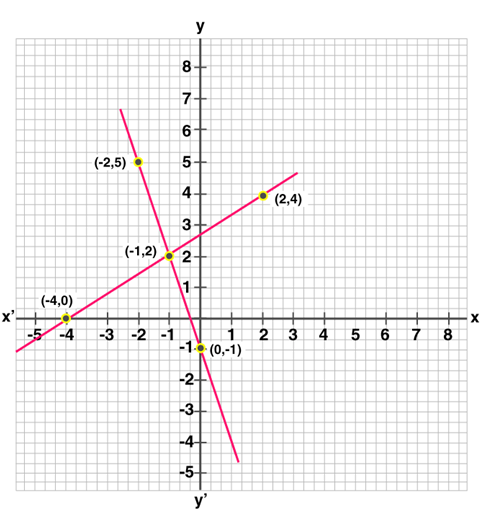Both the lines intersect each other at the points (-1, 2).

So, x = -1, y = 2

Question 7:

2x + 3y + 5 = 0

3x – 2y – 12 = 0

Solution:

2x + 3y + 5 = 0 …(1)

3x – 2y – 12 = 0 …(2)

Isolate x from equation (1) and find the values of x and y.

2x + 3y + 5 = 0

2x = -3y – 5

x = (-3y – 5) / 2

 x -4 -1 2 y 1 -1 -3

Similarly, Isolate x from equation (2) and find the values of x and y.

3x – 2y – 12 = 0

or 3x = 2y + 12

or x = (2y + 12 )/ 3

 x 4 0 2 y 0 -6 -3

Graph: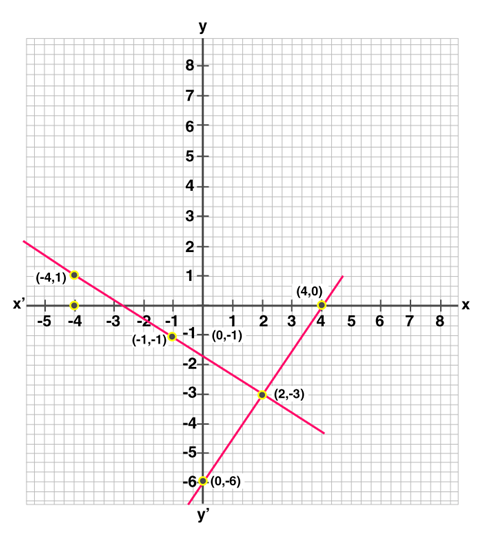Both the lines intersect each other at the point (2, -3).

So, x = 2, y = -3

Question 8:

2x – 3y + 13 = 0

3x – 2y + 12 = 0

Solution:

2x – 3y + 13 = 0 ……………(1)

3x – 2y + 12 = 0 …………..(2)

Isolate x from equation (1) and find the values of x and y.

2x – 3y + 13 = 0

or 2x = 3y – 13

or x = (3y – 13) / 2

 x -5 -2 1 y 1 3 5

Similarly, Isolate x from equation (2) and find the values of x and y.

3x – 2y + 12 = 0

or x = (2y – 12 ) / 3

 x -4 -2 0 y 0 3 6

Graph: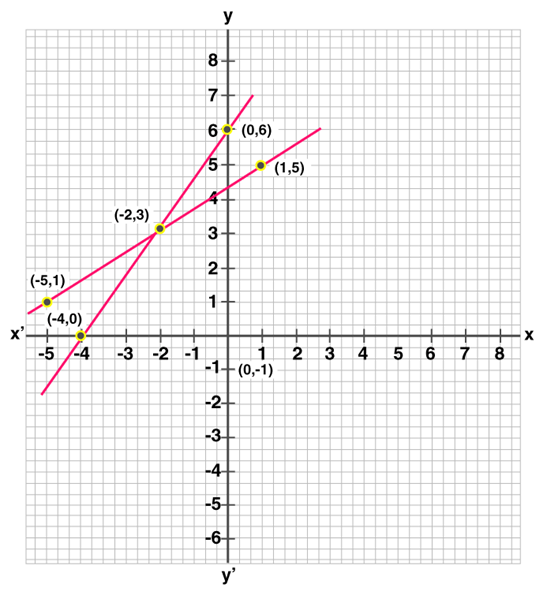Both the lines intersect each other at the point (-2, 3).

x = -2, y = 3

Question 9:

2x + 3y – 4 = 0

3x – y + 5 = 0

Solution:

2x + 3y – 4 = 0 ……(1)

3x – y + 5 = 0 …….(2)

Isolate x from equation (1) and find the values of x and y.

2x + 3y – 4 = 0

or x = (4 – 3y) / 2

 x 2 -1 5 y 0 2 -2

Similarly, Isolate x from equation (2) and find the values of x and y.

3x – y + 5 = 0

or y = 5 + 3x

 x 0 -1 -2 y 5 2 -1

Graph: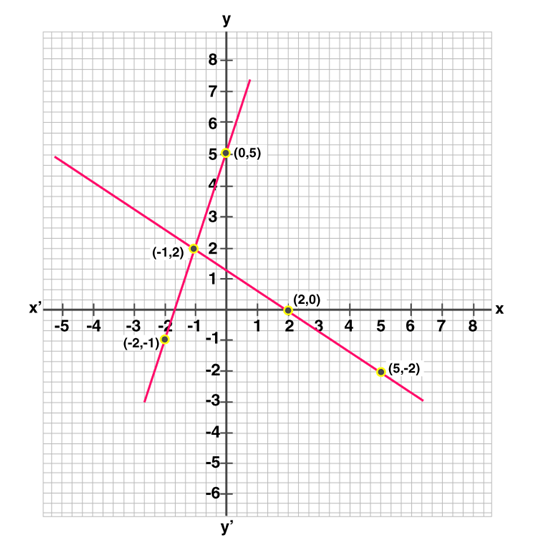Both the lines intersect each other at the point (-1, 2).

So, x = -1, y = 2

Question 10:

x + 2y + 2 = 0

3x + 2y – 2 = 0

Solution:

x + 2y + 2 = 0 …………(1)

3x + 2y – 2 = 0 …………(2)

Isolate x from equation (1) and find the values of x and y.

x + 2y + 2 = 0

or x = -2y – 2

 x -2 0 2 y 0 -1 -2

Similarly, Isolate x from equation (2) and find the values of x and y.

3x + 2y – 2 = 0

or x = (2 – 2y) / 3

 x 0 2 -2 y 1 -2 4

Graph: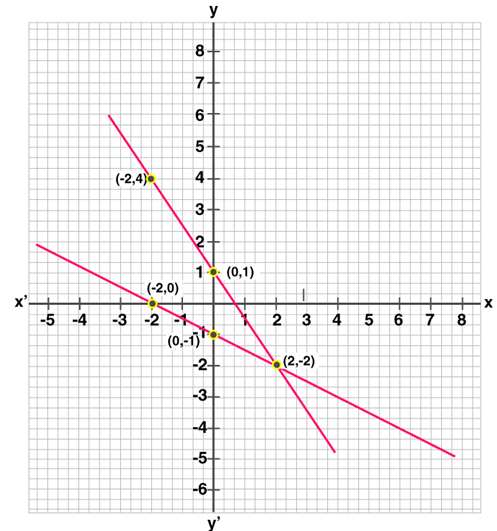Both the lines intersect each other at the point (2, -2).

So, x = 2, y = -2

Solve each of the following given systems of equations graphically and find the vertices and area of the triangle formed by these lines and the x-axis:

(Question 11 to Question 15)

Question 11:

x – y + 3 = 0, 2x + 3y – 4 = 0.

Solution:

x – y + 3 = 0 ………..(1)

2x + 3y – 4 = 0 ………(2)

Isolate x from equation (1) and find the values of x and y.

x – y + 3 = 0

or x = y – 3

 x y -3 0 -1 2 0 3

Similarly, Isolate x from equation (2) and find the values of x and y.

2x + 3y – 4 = 0

or x = (4 – 3y) / 2

 x y 2 0 -1 2 5 -2

Graph: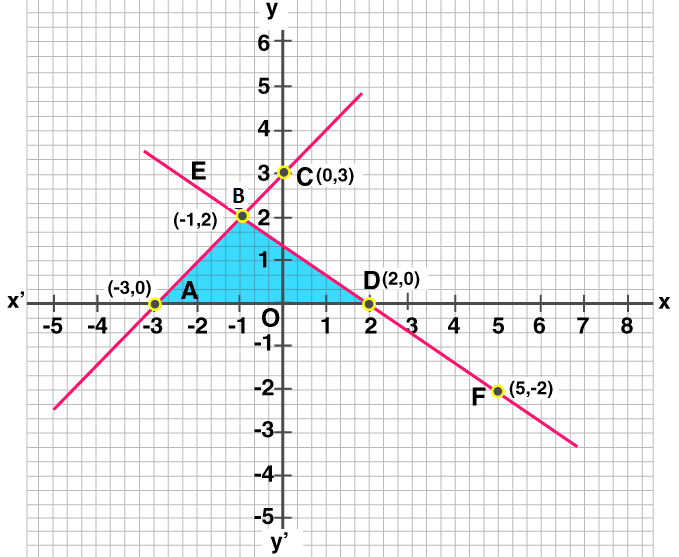Both the lines intersect each other at (-1, 2) and x-axis at A (-3, 0) and D (2, 0).

Now,

Find the area of triangle BAD:

Area of ∆BAD = 1/ 2 (base x altitude)

= 1/ 2 x 5 x 2

= 5 sq.units.

Question 12:

2x – 3y + 4 = 0, x + 2y – 5 = 0.

Solution:

2x – 3y + 4 = 0 …………(1)

x + 2y – 5 = 0 ……….(2)

Isolate x from equation (1) and find the values of x and y.

2x – 3y + 4 = 0

or x = (3y – 4) / 2

 x y -2 0 1 2 4 4

Similarly, Isolate x from equation (2) and find the values of x and y.

x + 2y – 5 = 0

or x = 5 – 2y

 x y 5 0 3 1 1 2

Graph: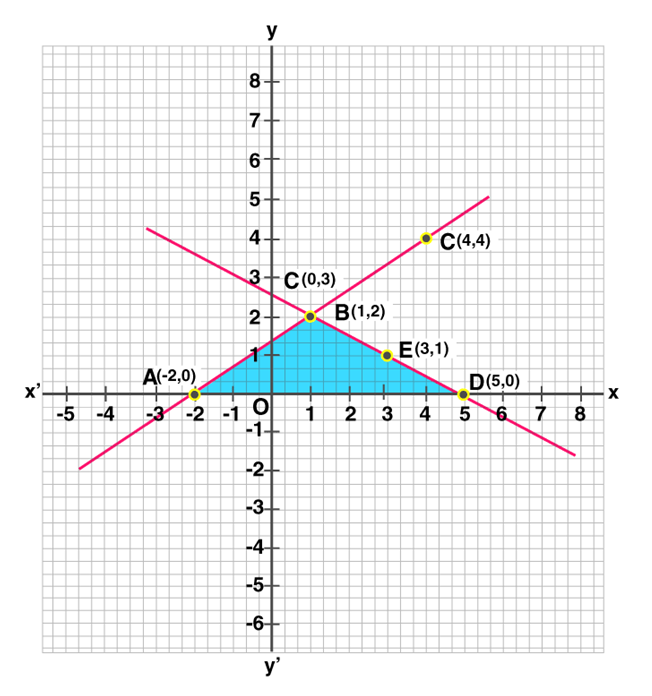Now,

Find the area of triangle BAD:

Area of ∆BAD = 1/2 x base x altitude

= 1/2 x 7 x 2

= 7 sq. units

Question 13:

4x – 3y + 4 = 0, 4x + 3y – 20 = 0.

Solution:

4x – 3y + 4 = 0 ……..(1)

4x + 3y – 20 = 0 ………(2)

Isolate x from equation (1) and find the values of x and y.

4x – 3y + 4 = 0

or x = (3y – 4) / 4

 x y -1 0 2 4 -4 -4

Similarly, Isolate x from equation (2) and find the values of x and y.

4x + 3y – 20 = 0

or x = (20 – 3y) / 4

 x y 5 0 2 4 -1 8

Graph: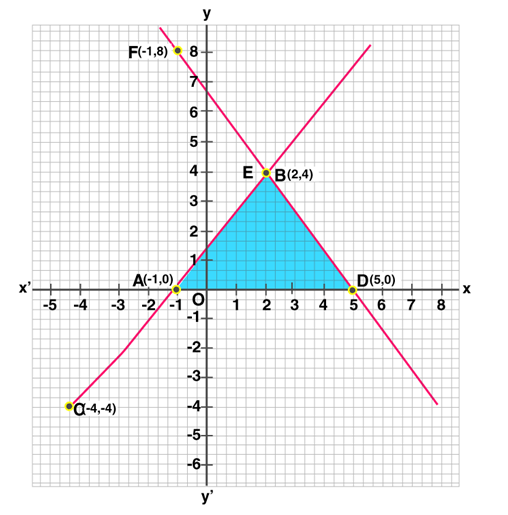Both the lines intersect each other at the point B (2, 4) and intersect x-axis at A (-1, 0) and D (5, 0).

To find the area of Triangle BAD:

Area ∆BAD = 1/2 x base x altitude

= ½ x 6 x 4

= 12 sq. units

Question 14:

x – y + 1 = 0, 3x + 2y – 12 = 0

Solution:

x – y + 1 = 0 ………….(1)

3x + 2y – 12 = 0 ………….(2)

Isolate x from equation (1) and find the values of x and y.

x – y + 1 = 0

or x = y – 1

 x y -1 0 0 1 1 2

Similarly, Isolate x from equation (2) and find the values of x and y.

3x + 2y – 12 = 0

or x = (12 – 2y) / 3

 x y 4 0 2 3 0 6

Graph: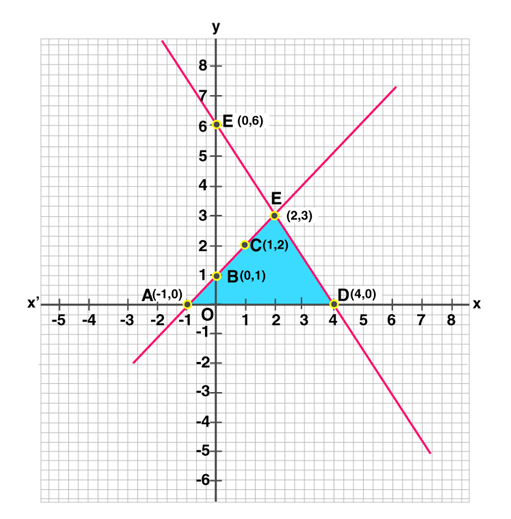Both the lines intersect each other at the point E (2, 3) and intersect x- axis at A (-1, 0) and D (4, 0).

To find the area of Triangle EAD:

Area of ∆EAD = 1/2 x base x altitude 1

= ½ x 5 x 3

= 7.5 sq. units

Question 15:

x – 2y + 2 = 0, 2x + y – 6 = 0.

Solution:

x – 2y + 2 = 0 ………..(1)

2x + y – 6 = 0 ………..(2)

Isolate x from equation (1) and find the values of x and y.

x – 2y + 2 = 0

or x = 2y – 2

 x y -2 0 0 1 2 2

Similarly, Isolate y from equation (2) and find the values of x and y.

2x + y – 6 = 0

Or y = 6 – 2x

 x y 0 6 2 2 3 0

Graph: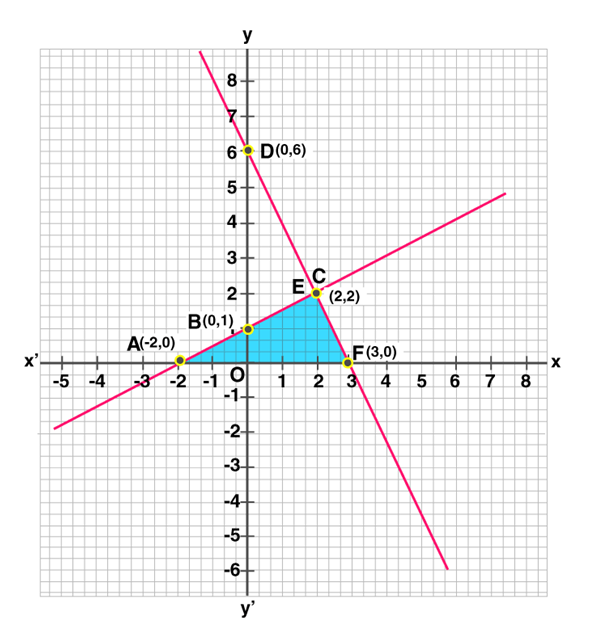Both the lines intersect each other at the point C (2, 2) and intersect the x-axis at A (-2, 0) and F (3, 0).

Now, To find the area of Triangle CAF:

Area of ∆CAF = 1/2 x base x altitude

= 1/2 x 5 x 2

= 5 sq. units

Solve each of the following given systems of equations graphically and find the vertices and area of the triangle formed by these lines and the y-axis:

(Question 16 to Question 21)

Question 16:

2x – 3y + 6 = 0, 2x + 3y – 18 = 0.

Solution:

2x – 3y + 6 = 0 ……….(1)

2x + 3y – 18 = 0 ……….(2)

Isolate x from equation (1) and find the values of x and y.

2x – 3y + 6 = 0

or x = (3y – 6) / 2

 x y -3 0 0 2 3 4

Similarly, Isolate x from equation (2) and find the values of x and y.

2x + 3y – 18 = 0

or x = (18 – 3y) / 2

 x y 0 6 3 4 6 2

Graph: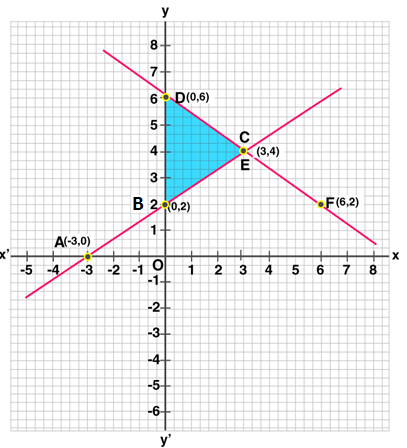Both the lines intersect each other at C (3, 4) and intersect y-axis at B (0, 2) and D (0, 6).

Now, To find the area of Triangle CBD:

Area of ∆CBD = 1/2 x base x altitude

= 1/2 x 4 x 3 sq. units

= 6 sq. units

Question 17:

4x – y – 4 = 0, 3x + 2y – 14 = 0.

Solution:

4x – y – 4 = 0 ………..(1)

3x + 2y – 14 = 0 …………(2)

Isolate x from equation (1) and find the values of x and y.

4x – y – 4 = 0

or x = (y + 4) /2

 x y 1 0 0 -4 2 4

Similarly, Isolate y from equation (2) and find the values of x and y.

3x + 2y – 14 = 0

or y = (14 – 3x) / 2

 x y 0 7 2 4 4 1

Graph: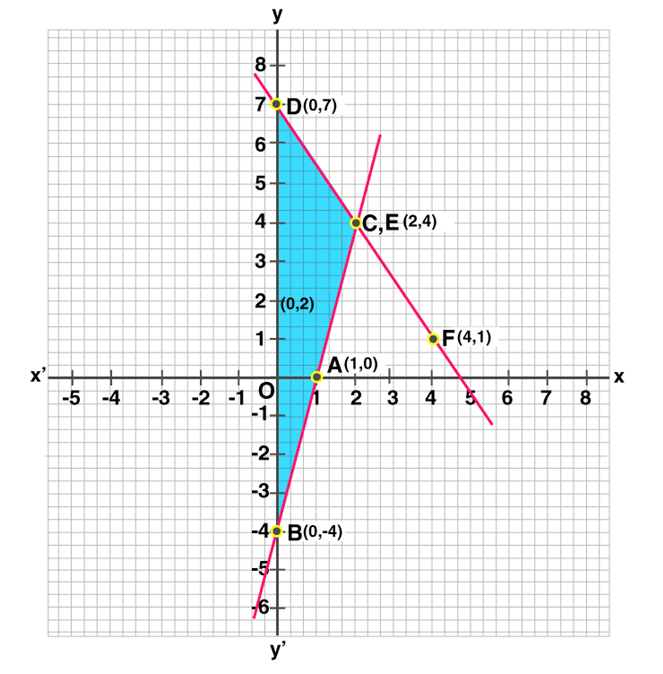Both the lines intersect each other at C (2, 4) and y-axis at B (0, -4) and D (0, 7).

To find the area of Triangle CBD:

Area of ∆CBD = 1/2 x base x altitude

= 1/2 x 11 x 2

= 11 sq. units

Question 18:

x – y – 5 = 0, 3x + 5y – 15 = 0

Solution:

x – y – 5 = 0 …….(1)

3x + 5y – 15 = 0 ….(2)

Isolate x from equation (1) and find the values of x and y.

x – y – 5 = 0

or x = y + 5

 x y 5 0 0 -5 1 -4

Similarly, Isolate x from equation (2) and find the values of x and y.

3x + 5y – 15 = 0

or x = (15 – 5y ) /3

 x y 5 0 0 3 -5 6

Graph: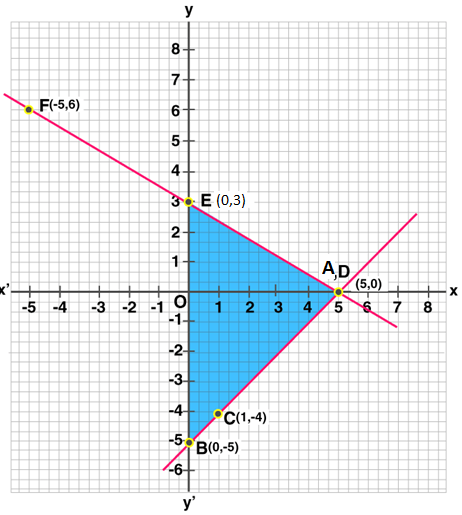Both the lines intersect each other at A (5, 0) and y-axis at B (0, -5) and E (0, 3).

To find the area of Triangle ABE:

Area of ∆ABE = 1/2 x base x altitude

= 1/2 x 8 x 5

= 20 sq. units

Question 19.

2x – 5y + 4 = 0, 2x + y – 8 = 0.

Solution:

2x – 5y + 4 = 0 ……..(1)

2x + y – 8 = 0 …….(2)

Isolate x from equation (1) and find the values of x and y.

2x – 5y + 4 = 0

or x = (5y – 4) /2

 x y -2 0 3 2 -7 -2

Similarly, Isolate x from equation (2) and find the values of x and y.

2x + y – 8 = 0

or x = (8 – y) / 2

 x y 4 0 3 2 2 4

Graph: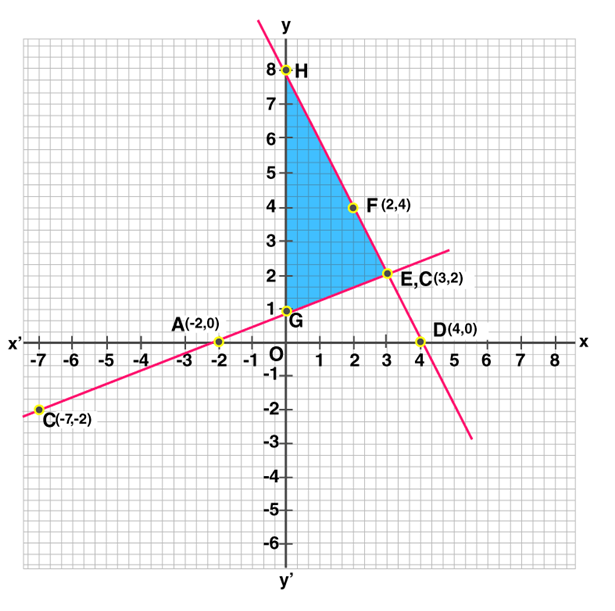Both the lines intersect each other at B (3, 2) and y-axis at G (0, 1) and H (0, 8).

To find the area of Triangle EGH:

Now area of ∆EGH = 1/2 x base x altitude

= 1/2 x 7 x 3

= 10.5 sq. units

Question 20:

5x – y – 7 = 0, x – y + 1 = 0

Solution:

5x – y – 7 = 0 ………….(1)

x – y + 1 = 0 ………….(2)

Isolate y from equation (1) and find the values of x and y.

5x – y – 7 = 0

or y = 5x – 7

 x y 0 -7 1 -2 2 3

Similarly, Isolate x from equation (2) and find the values of x and y.

x – y + 1 = 0

or x = y – 1

 x y -1 0 1 2 2 3

Graph: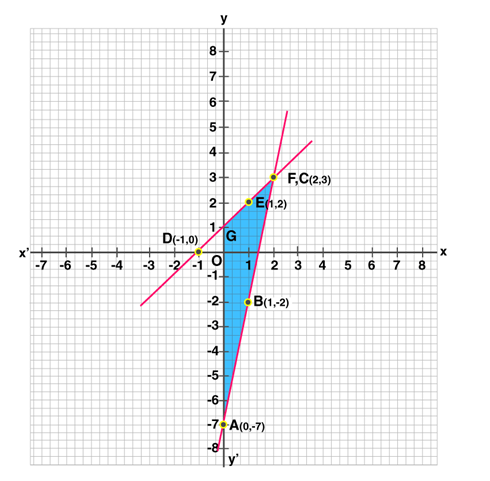Both the lines intersect each other at C (2, 3) and intersect y-axis at A (0, -7) and F (0, 1).

Now, To find the area of Triangle CAG.

Area of ∆CAG = 1/2 x base x altitude

= 1/2 x 8 x 2

= 8 sq.units

Question 21:

2x – 3y = 12, x + 3y = 6

Solution:

2x – 3y = 12 …………(1)

x + 3y = 6 …………(2)

Isolate x from equation (1) and find the values of x and y.

2x – 3y = 12

or x = (12 + 3y) /2

 x y 6 0 3 -2 0 -4

Plot the points (6, 0), (3, -2) and (0, -4) on the graph and join them to get a line.

Similarly, Isolate x from equation (2) and find the values of x and y.

x + 3y = 6

or x = 6 – 3y

 x y 6 0 0 2 -6 4

Graph: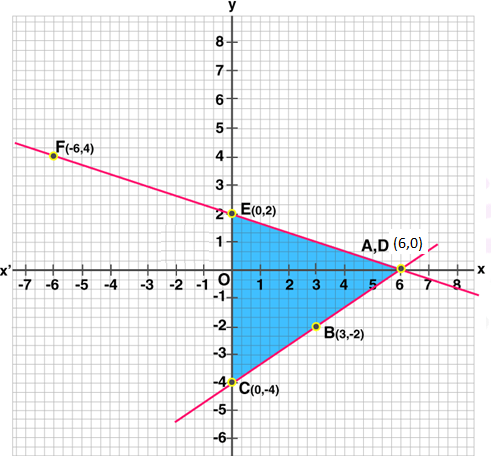Both the lines intersect each other at the points A (6, 0) and intersect the y-axis at C (0, -4) and E (0, 2).

To find the area of Triangle ACE:

Now area of ∆ACE = 1/2 x base x altitude

= 1/2 x CE x AO

= 1/2 x 6 x 6

= 18 sq.units

Show graphically that each of the following given systems of equations has infinitely many solutions:

Question 22:

2x + 3y = 6, 4x + 6y = 12

Solution:

2x + 3y = 6 …………(1)

4x + 6y = 12 …………(2)

Isolate x from equation (1) and find the values of x and y.

2x + 3y = 6

or x = (6 – 3y) / 2

 x y 3 0 0 2 -3 4

Isolate x from equation (2) and find the values of x and y.

4x + 6y = 12

or x = (12 – 6y) / 2

 x y 3 0 3/2 1 -3/2 3

Graph: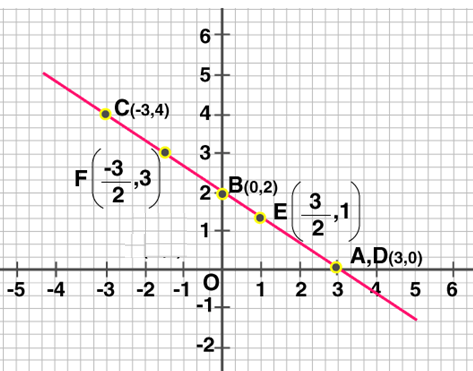From the graph, we can see that, all the points lie on the same straight line.

Which shows that, system has infinite many solutions.

Question 23:

3x – y = 5, 6x – 2y = 10.

Solution:

3x – y = 5 …………..(1)

6x – 2y = 10 ………….(2)

Isolate y from equation (1) and find the values of x and y.

3x – y = 5

or y = 3x – 5

 x y 0 -5 1 -2 2 1

Similarly, Isolate x from equation (2) and find the values of x and y.

6x – 2y = 10

Or x = (10 + 2y)/ 6

 x y 2 1 4 7 3 4

Graph: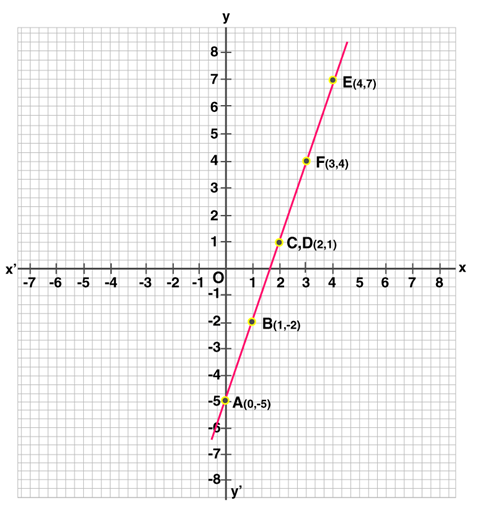Both the lines coincide each other. Which shows that system has infinite many solutions.

Question 24:

2x + y = 6, 6x + 3y = 18

Solution:

2x + y = 6 ……….(1)

6x + 3y = 18 ….…..(2)

Isolate y from equation (1) and find the values of x and y.

2x + y = 6

or y = 6 – 2x

 x y 0 6 2 2 4 -2

Similarly, Isolate x from equation (2) and find the values of x and y.

6x + 3y = 18

or x = (18 – 3y )/ 2

 x y 3 0 1 4 5 -4

Graph: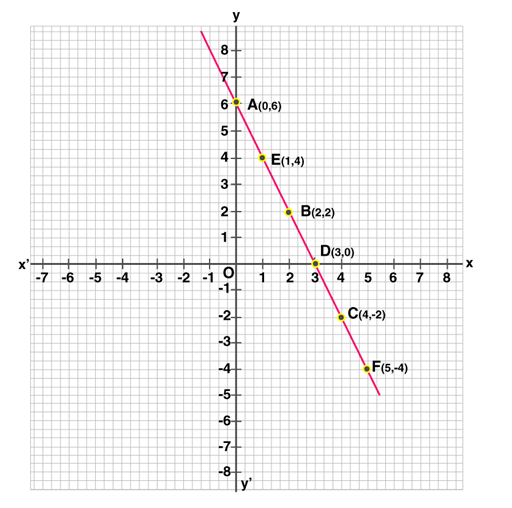Both the lines coincide each other.

This system has infinitely many solutions

Question 25:

x – 2y = 5, 3x – 6y = 15.

Solution:

x – 2y = 5 ……(1)

3x – 6y = 15 …….(2)

Isolate x from equation (1) and find the values of x and y.

x – 2y = 5

or x = 5 + 2y

 x y 5 0 3 -1 1 -2

Similarly, Isolate x from equation (2) and find the values of x and y.

3x – 6y = 15

or x = (15 + 6y) / 3

 x y 7 1 -1 -3 -3 -4

Graph: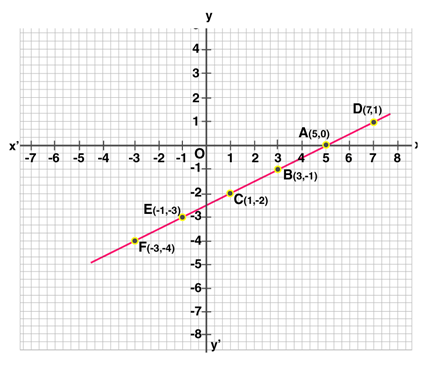All the points lie on the same line.

Which shows that, lines coincide each other. Hence, the system has infinite many solutions.

Show graphically that each of the following given systems of equations is inconsistent, i.e., has no solution:

(Question 26 to Question 29)

Question 26:

x – 2y = 6, 3x – 6y = 0.

Solution:

x – 2y = 6 ………….(1)

3x – 6y = 0 …….(2)

Isolate x from equation (1) and find the values of x and y.

x – 2y = 6

or x = 6 + 2y

 x y 6 0 4 -1 0 -3

Similarly, Isolate x from equation (2) and find the values of x and y.

3x – 6y = 0

or x = 2y

 x y 0 0 2 1 4 2

Graph: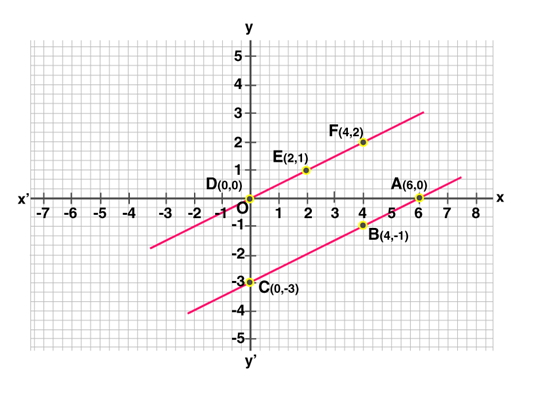From the graph, we can see that both the lines are parallel to each other.

This system has no solution.

Question 27:

2x + 3y = 4, 4x + 6y = 12.

Solution:

2x + 3y = 4 …………(1)

4x + 6y = 12 ……….(2)

Isolate x from equation (1) and find the values of x and y.

2x + 3y = 4

or x = (4 – 3y )/ 2

 x y 2 0 -1 2 5 -2

Similarly, Isolate x from equation (2) and find the values of x and y.

4x + 6y = 12

or x = (12 – 6y )/ 4

 x y 3 0 0 2 -3 4

Graph: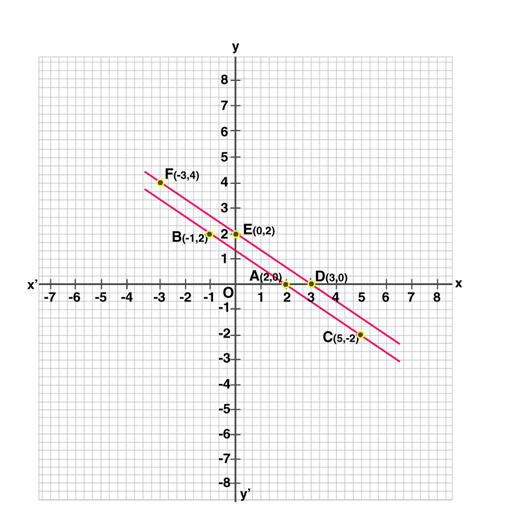From the graph, both the lines are parallel.

Therefore given system has no solution.

Question 28.

2x + y = 6, 6x + 3y = 20.

Solution:

2x + y = 6 …….(1)

6x + 3y = 20 ………(2)

Isolate y from equation (1) and find the values of x and y.

2x + y = 6

or y = 6 – 2x

 x y 0 6 1 4 3 0

Similarly, Isolate x from equation (2) and find the values of x and y.

6x + 3y = 20

or x = 1/6(20 – 3y)

 x y 10/3 0 7/3 2 4/3 4

Graph: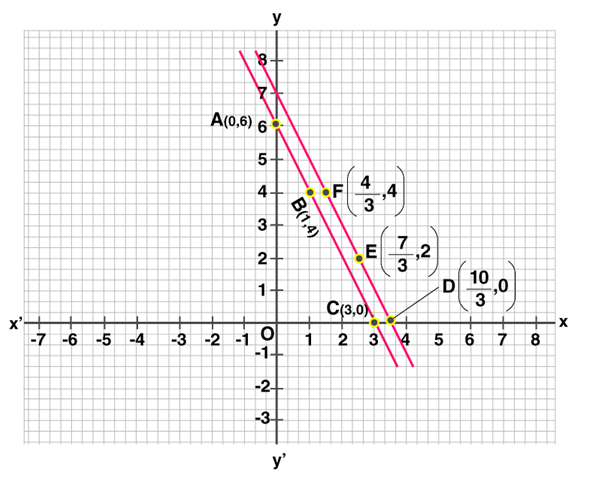From the graph, both the lines are parallel.

Therefore this system has no solution.

Question 29: Draw the graphs of the following equations on the same graph paper:

2x + y = 2, 2x + y = 6.

Find the coordinates of the vertices of the trapezium formed by these lines. Also, find the area of the trapezium so formed.

Solution:

2x + y = 2 ………….(1)

2x + y = 6 ………….(2)

Isolate y from equation (1) and find the values of x and y.

2x + y = 2

or y = 2 – 2x

 x y 0 2 1 0 -2 6

Similarly, Isolate y from equation (2) and find the values of x and y.

2x + y = 6

or y = 6 – 2x

 x y 0 6 2 2 3 0

Graph: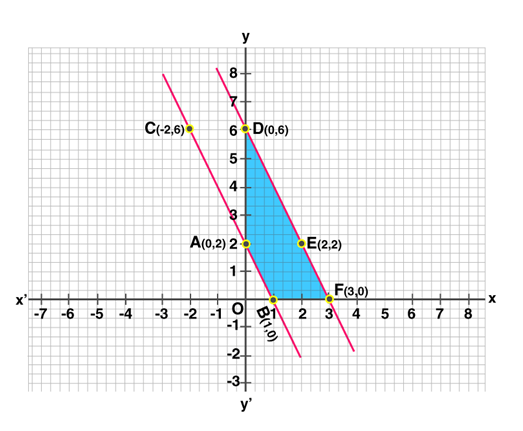From above graph: ABFD is the trapezium formed by lines whose vertices are A (0, 2), B (1, 0), F (3, 0), D (0, 6).

Now, find the area of trapezium :

Area of trapezium ABFD = Area ∆DOF – Area ∆AOB

= 1/2 (DO x OF) – 1/2 (AO x OB)

= 1/2 (6 x 3) – 1/2 (2 x 1)

= 8 sq.units.

## RS Aggarwal Solutions for Class 10 Maths Chapter 3 Linear Equations in Two Variables Exercise 3A

Class 10 Maths Chapter 3 Linear Equations in Two Variables Exercise 3A is based on the topic: Solving simultaneous linear equations using graphical method.